<15v>

## How to find ye axes vertices Diamiters, \Centers,/ or Asymptotes of any Crooked Line supposeing it have them.

 Definitions. If kgach is a crooked line, & ef touch it at ye point a . & if ye line lines cg hk & all other lines wch being par{illeg}|a|lle{illeg}|l|l to one another & extended from one si{illeg}de of ye \{illeg}/ croked line to ye other and also bisected by ye streight line ai . Then is ai a Diameter, & cbg one of those lines wch are ordinately applyed to it, & if ye angles {illeg}, cbd , &c are right ones Then is abd t{illeg}|he| axis, & cbg one of those lines wch are ordinately applyed to ye axis.

 3 The Vertex of a crooked line is yt point where ye crooked line intersect the diameter or axis as at $\left(a\right)$

 4 An {illeg} The \The/ Asymptote{illeg} of {illeg} crooked lines are such lines wch being produced both ways infinitely have noe least distance twixt ym & ye crooked line & yet {doe} noe where intersect it. \or touch it/ as dδ , dθ .

 5 Those lines wch are limited on all sides \& have axes but/ as acxkλ are Ellipses if ye of ye first, 2d, 3d, 4th kind &c

6 Those wch are not ellipses & have \axes but/ noe Asyptotes are {illeg} Parabolas of ye first, 2d, 3d, 4th kind {illeg}|&|c. as zkah .

7 Those wch have {illeg} Asymptotes, are Hyperbolas of ye 1st, 2d, 3d 4th kind, &c as (upon) whose asymptotes are βd , γd .

8 There are some lines of a middle nature twixt {illeg}|a| Parab: & hyperb: have an asymto{te} haveing an Asymptote for one of its s{illeg}|i|d{illeg}|e|s wch are but none for ye other as βαγe , one side αγ haveing ye asymptoe {sic} δe , ye other side αβ haveing none.

10 If an Ellipsis have 2 axes (a{illeg}|s| am & xλ ) ye longer is ye transverse axis {illeg}|(|as am ) ye shorter is ye right axis (as xλ ).

If all those lines wch are parallell to one of ye diameters of ye crooked line & are terminated by ye crooked line be bisected

9 If two diameters of ye same Ellipsis be ordinately applyed {illeg}|ye| one to ye other ye shortest of them is called ye right diameter, ye longest ye transverse one. ({illeg} {illeg}) (as am & xλ ).

If {illeg} all ye parallells wch are terminated by \ye same or by/ 2 divers figures, are bisected by ye same streight line, yn is yt line \called/ a \right/ diameter. But if That yt diameter wch is ordin{illeg}|a|tely applied to it (i.e. wch is par{illeg}|a|llell to these parallells) is a transverse diameter.

1 If all ye parallell lines wch are terminated by {illeg}|t|he same or by 2 divers figures, bee bisected by a streight line; yt bisecting line is a diameter, & those paralle{l} lines, are lines ordinately a{illeg}|p|plied to yt diameter.

2 If those parallell lines intersect ye diameter at right angles yt|e| diameter is {illeg} an axis

A line is said to bee of ye first kind wh

In an Ellipsis ye point where 2 diameters intersect, is {illeg} \ye/ center.

The center of an{illeg} figure \Ellipsis/ is yt point where {illeg} two \of {illeg} its/ diameters intersect.

{illeg} The center of two di{illeg}ers figure{illeg}|s| of t \opposite/ /Hyperbol{illeg}|a|s\ is yt point where two of their diameters intersect one another or else where there {sic} Asymptotes intersect.

<16r>

Propositions. The lines ordinately ap{illeg}|p|lie{illeg}|d| to ye axis of a crooked line are parallell to ye tangent of ye crooked line at its vertex.

 Demonstr. Suppose \ chad a Parab &/ dc (being ordinately applied to ye axis ab ) not parallell to ye tan{illeg}|g|ent an but to some oth{illeg}|e|r line like ah. If dc bee understood to move {illeg}|to|wards a db continually decreas|i||ng| \{illeg}/ un{illeg}|t|ill it vanish into nothing at ye conjucti{illeg}|o|n of ye points a & d . but cb sinc & since cb mus{illeg}|t| always be equall to ah at ye conju{illeg}|n|tion of ye point {sic} a & d . it followeth yt cb cannot decrease so as to vanish into nothing at ye same time wch bd doth & therefore cannot allways be = to bd .

 Otherwise. if dc is not parallell to ye tangent an but to some other line as ah . Then ab doth not bisec{illeg}|t| all ye parallell li{illeg}|n|es (as oe ) wch are terminated by ye crooked line cad . & therefore cannot bee its diam:

 |2dly.| If ad is ye axis of a crooked line & $cb=y$, is ordinately a{illeg}|p|plied to ad . yt is if $bc=ce=y$ . Then y {illeg} must be found noe where of odd dimensions in ye Equation \expressing ye nature of ye line cod/. For (suppose{in}g $y=bc=ce$ to be ye unknowne quantity) y hath 2 valors bc & cd equall {illeg}|to| one anot{illeg}|h|er excepting yt ye one bc is affirmative, ye other ce is negative. wch two valors canot {sic} bee exprest by an equation in wch y is of od {sic} dimensions for suppose $yy=aa$ . yn i{illeg}|s| is {sic} $y=+a$, or $y=-a$ $y=$ $\sqrt{aa}=a$, since $a×a=aa$. & $\sqrt{aa}=-a$, since $-a×-a=aa$. & $y=\sqrt{aa}$ therefore is $y=+a$, or $y=-a$. soe if ${y}^{4}={a}^{4}$. yn is $yy=aa$ , & $y=a$ or $y=-a$ but if ${y}^{3}={a}^{3}$. yn $y=\sqrt{c:{a}^{3}}=a$ . but not $y=\sqrt{c:-{a}^{3}}=-a$. soe i{illeg}|f| ${y}^{5}={a}^{5}$. yn $y=a=\sqrt{qc:{a}^{5}}$ but not $y=-5=\sqrt{qc:-{a}^{5}}$. The same reason is cogent in compound equations. as if $yy-2xy+xx=ax$. Then, $y=x\phantom{\rule{0.5em}{0ex}}\stackrel{\cup }{O}\phantom{\rule{0.5em}{0ex}}\sqrt{ax}$. where though ye root $a+\sqrt{ax}$ is affirmative & ye roote $a-\sqrt{ax}$ may bee negative yet they can never be equall in length, & though ye 2 roots of an equation wch differ in signes should bee equally long y{illeg}|e|t yt is w{illeg}|h|en there is but one quantity considere ye Equation is fully determined.

 Prop: 4th. If x is of more dimensions in a quantity not multiplied by x yn in one multiplied by it (as in ${y}^{2}=${illeg}$xy+aa$) yn y is not parallel to one of ye lines Asymptotes. & e contra. Otherwise x & y are parallel to ye Asymptotes of ye line. et e contra.

Prop 3d. If ag is ye Asymptote of ye crooked line dcf , & $ab=x$ is coincident wth it, then & $bc=y$. then in ye Equation (expressing ye relation twixt x & y ,) x must be of more dimensions in some \one/ quantity \onely/ where it is multiplyed by y or $yy$: &c in any other quan{illeg}|t|ity in ye Equation \& y of as many/. & if ae is an Asymptote to ye same line dcf , & $bc=y$ bee parallell to it then y must noe where be of soe many dimensions as in if \one/ quantity \onely/ in wch {illeg}|t|is drawne into x , $xx$ , \or/ ${x}^{3}$ &c. Demonstraco. If this be false yn suppose ye equation expressing ye nature relation twixt x & y to be $xyy+ayy$ {illeg} $-bxy-{d}^{3}=0$ . first if x is coincident wth ye Asymptote ag yn, if y | x | $=\frac{ee}{o}$ (yt is if x being infinite in length) i{illeg}|t| is $y=0$, if $x=\frac{ee}{o}$ (yt {illeg}|i|s y vanisheth into nothing if x be infinite. {illeg}|)| therefore tansposeing {sic} $\frac{ee}{o}$ into ye place of x , I find $\frac{eeyy}{o}+\genfrac{}{}{0}{}{ayy}{\phantom{o}}-\frac{beey}{o}-\genfrac{}{}{0}{}{{d}^{3}}{\phantom{o}}=0$. Or $eeyy+o×ayy-beey-o×{d}^{3}=0$ that is $eeyy=beey$ /+||{$ee$}\, or $y=b$. Now since y vanishe{illeg}|th| not it followeth y{illeg}|t| x is not coin
cident wth ye Asymptote. But if I blot out {one} of ye terme{illeg} {illeg} as $-bxy$ So: if ye equation be $xx+xy=aa$ . yn by substituteing $\frac{ee}{o}$ into ye place of x I have $\frac{{e}^{4}}{oo}+\frac{eey}{o}=aa$, or $y=\frac{{o}^{2}aa-{e}^{4}}{oee}=\frac{ee}{o}$ soe yt y not vanishing but being infinite x cannot be asymptote. But if ye equation {illeg} was $ayy-bxy={d}^{3}$ . yn by writeing $\frac{ee}{o}$ for x , there results, $\frac{eeyy}{0}+ayy={d}^{3}$. or $yy=\frac{o{d}^{3}}{oa+ee}=0$ . & therefore in this case x is coincident ye asymptote ag . Soe if $ayy-bxy-{d}^{3}=0$ , by making $\frac{ee}{o}=x$ . it is $ayy-\frac{beey}{o}$ {illeg} $-{d}^{3}=0$ . Or by extracting ye roote $\frac{bee}{2ao}\phantom{\rule{0.5em}{0ex}}\stackrel{\cup }{O}\phantom{\rule{0.5em}{0ex}}\sqrt{\frac{{e}^{4}bb-4{d}^{3}ooa}{4aaoo}}$ . Soe yt y hath 2 roots ye one infinitly \grate {sic}/ wch is $\frac{bee}{2ao}+\frac{\sqrt{{e}^{4}bb-4{d}^{3}aoo}}{2ao}=\frac{bee}{ao}=y$ . ye other is infinitely little wch is $\frac{bee}{2ao}-\sqrt{\frac{{e}^{4}bb-o}{4aaoo}}=\frac{bee-bee}{2ao}=o=y$ .
bee multiplied by y wherever i{illeg}|t| is of its greatest dimensions. & if ae is an asymptote to ye line dcf , & $bc=y$ be parallel to it, ye & $ab=x$ terminated by it at y{illeg}|e| point a , {illeg}|th|en must y be multiplied by x wherever it is of its greatest dimensions. Example: Suppose $axx+yxx={b}^{3}$. because in these 2 termes $axx+yxx$ x is of it {sic} greatest dimensions; but in one of ym (viz: $axx$ ) it is not multiplyed by y therefore x is not coincident wth ag ye asymptote. If $yyxx+ayxx$ + /\ ${a}^{3}x-{a}^{4}=0$ . then since x is of its greate{illeg}|s|t dimens: in $yyxx$ & $ayxx$ onely & is drawne into y in both of ym therefore x is coincident wth ye Asymptote{;} I Also since y is of its greatest dimension{illeg}|s| in $xxyy$ onely, {illeg}d (wch {illeg} {b}{l} multiplied {illeg} by x ) therefore y is parallel to {illeg} x {illeg} {terminated} by an Asymptote. &c.

Demonstr: If x is coincident wth ye Asymptote yn { $\frac{ee}{o}=x$ } when $o=y$ . i:e: x is infinite when {illeg} {wisheth}. Now suppose $yyxx+ayxx-$ ${a}^{3}x$ $={a}^{4}$. yn i{illeg}|f| $y=o$ is $x=\frac{aa}{\sqrt{oo+ao}}$ . i:e: x is {illeg} but if $yyxx+aaxx={a}^{4}$. yn if $y=o$ it is {$x=\frac{aa}{\sqrt{oo+aa}}=\phantom{\rule{0.5em}{0ex}}\stackrel{\cup }{O}\phantom{\rule{0.5em}{0ex}}a$ }. soe {yt} x is finite & therefor{e}{illeg} coincident wth ye {illeg} de{illeg}{illeg} of {illeg} is like{illeg}

<16v>

##  Haveing {an} the nature of a crooked line expresed in al{illeg}|g|ebraicall termes to find its axi|e|s if {i {sic}}{i}{shave}{ anq }

 Draw a line infinitely both ways fix upon some point (as b ) for ye begining of one of ye unknowne quantitys (wch I call x . Then reduce ye Equation to such an order (if it bee not already so) yt x may be always found in ye line bc . wth one end fixed at b , & having y moving making right angles wth it at ye other end: yt end of y wch is remote from x , describing ye crooked line. wch may bee {illeg}|al|ways done wthout any great difficulty. As may be percei{illeg}|v|ed by these examples.

 Suppose ye given Equation was ${y}^{3}=axx$ . x & y {illeg} soe yt $bg=x$ . $gd=y$ . dc being perpendicular to bc & ye angle dgc being given, ye proportion twixt dg & dc is given, wch I supose as d to e . yn is $d:e\colon\colon y:\frac{ey}{d}$ {illeg}c {illeg} $\begin{array}{c}{gd}^{2}-{gc}^{2}={dc}^{2}\\ yy-\frac{eeyy}{dd}={w}^{2}\end{array}$. $\frac{dw}{\sqrt{dd-ee}}=y$. $\begin{array}{c}bg+gc\\ x+\frac{ey}{d}\end{array}$ $d:e\colon\colon y:dc=\frac{ey}{d}=w$ . & $\frac{dw}{e}=y$ . ${gc}^{2}\phantom{\rule{0.5em}{0ex}}\begin{array}{l}={gd}^{2}-{dc}^{2}\\ =yy-ww\end{array}\phantom{\rule{0.5em}{0ex}}=\frac{ddww-eeww}{ee}$ . or $\frac{g}{c}\sqrt{dd-ee}$ . & $bc=v=\frac{w\sqrt{dd-ee}}{e}$ . Or $\frac{ev-w\sqrt{dd-ee}}{e}=x$ . Therefore I write $\frac{{d}^{3}{w}^{3}}{{e}^{3}}$ for ${y}^{3}$ , {illeg}|&| $\frac{eevv-2evw\sqrt{dd-ee}+ddww-eeww}{ee}$ for $xx$ in ye equation ${y}^{3}=axx$ , & soe I have this equation $\frac{{d}^{3}{w}^{3}}{{e}^{3}}=\frac{aeevv-2aevw\sqrt{dd-ee}+add{w}^{2}-aee{w}^{2}}{ee}$ . wch expresseth ye relation twixt w & v , yt is twixt dc & bc , {illeg}|w|riteing therefore y for dc , & x for bc : I have this equation ${d}^{3}{y}^{3}-aeeexx=$ \{o}{ 0 }/ ${d}^{3}{y}^{3}+a{e}^{3}yy-aeddyy+2aeexy\sqrt{dd-ee}-a{e}^{3}xx$

 Soe if x \ $=bd$ / turned about ye pole b & y \$=dg$/ about ye pole g j{illeg} describing ye croo{illeg}|k|ed line ad by ye conjunction at ye extremitys. & ye equation expresing ye relation wch they bea{illeg}|r|e to one another is $xx$ {illeg}$xy$ $xx=ay$. ye distance of ye poles is given wch I call $b=bg$ . perpendic: to bg I draw $dc=w$ & make $bc=v$ . Then, is $\begin{array}{c}{dc}^{2}+{bc}^{2}={bd}^{2}\text{.}\\ {w}^{2}+{v}^{2}=xx\text{.}\end{array}$ $\begin{array}{r}{dc}^{2}+{cg}^{2}={dg}^{2}\text{.}\\ {w}^{2}+bb-2bv+vv=yy\text{.}\end{array}$ soe yt for $xx=ay$ {illeg} I write ${w}^{2}+{v}^{2}=a\sqrt{{w}^{2}+bb-2bv+vv}$. Or ${w}^{4}+2{w}^{2}{v}^{2}+{w}^{4}=aa{w}^{2}+aabb-2aabv+aavv$ . wch expresseth ye relation wch bc beareth to dc , & by makeing $bc=x$ , $dc=y$ , it is, $\begin{array}{lllllll}{y}^{4}& +& 2xxyy& +& {x}^{4}& =& 0\\ & -& aayy& +& 2aabx\\ & & & -& aaxx\\ & & & -& aabb\end{array}$.

Examp 3d If $bg=x$ {illeg}|b|e always in ye line bc . & fd turning about ye pole f {illeg}|&| passing by ye end of $bg=x$ wth its other end d describes ye crooked line bdh , soe yt calling gd y $x=y$ . yn drawing ef & dc perpendicular to bh . $be=a$ , & $ef=b$ are given. & I make $bc=v$ {.} $dc=w$ . yn is $eg=x-a$ . $gc=v-x$ . $\begin{array}{c}ef:eg\colon\colon gc:cd\text{.}\\ b:x-a\colon\colon v-x:w\text{.}\end{array}$ $bw=vx-av+ax-xx$. or by extracting ye roote $x=\frac{+a+v}{2}\phantom{\rule{0.5em}{0ex}}\stackrel{\cup }{O}\phantom{\rule{0.5em}{0ex}}\sqrt{\frac{aa+2av+vv-4bw}{4}}$ . againe $\begin{array}{c}{gc}^{2}+{dc}^{2}={gd}^{2}\\ vv-2vx+xx+{w}^{2}=yy=xx\text{.}\end{array}$ . Or $\frac{vv+{w}^{2}}{2w}=x=\frac{a+v}{2}\phantom{\rule{0.5em}{0ex}}\stackrel{\cup }{O}\phantom{\rule{0.5em}{0ex}}\sqrt{\frac{aa+2av+vv-4bw}{4}}$ . & by transposeing $\frac{a+v}{2}$ {illeg}|to| ye other side & so squareing both pts ${w}^{4}-2av{w}^{2}=2a{w}^{3}+{v}^{4}-4bw{v}^{2}$ . wch equation expresseth ye relation twixt $w=dc$ , & v {illeg} $=bc$ . & so by {illeg} calling dc y , & bc x , it is, ${y}^{4}-2axyy+4bxxy-{x}^{4}-2a{x}^{3}=0$ .

Example 4th. if $bd=x$ turnes about ye pole b , & gd (a given line $=a$) slides upon bg wth one end & intersecting bd at right angles at ye other end describes ye crooked line \ bde / by its intersection wth bd . then makeing $bc=v$ , $dc=w$ . $\begin{array}{c}{bc}^{2}+{dc}^{2}={bd}^{2}\text{.}\\ {v}^{2}+{w}^{2}={x}^{2}\text{.}\end{array}$ $\begin{array}{c}bc:bd\colon\colon dc:dg\text{.}\\ v:x\colon\colon w:a\text{.}\end{array}$ & $\frac{av}{w}=x$ therefore $vv+ww=\frac{aavv}{ww}$ or ${w}^{4}+vvww=aavv$ . & so by writing x for v & y for w , I have ye relation twixt $x=bc$ & $y=dc$ ex{illeg}|p|rest in this equation ${y}^{4}+xxyy-aaxx=0$ .

Or if ye relation twixt bd & dg {illeg} was exprest in this equation ({illeg}|m|a{illeg}|k|ing $dg=y$ . $bd=x$ ) $\begin{array}{c}xxy\\ +ayy\end{array}={a}^{3}$. then {illeg} as before $\begin{array}{c}{bc}^{2}+{dc}^{2}={bd}^{2}\text{.}\\ {v}^{2}+{w}^{2}={x}^{2}\text{.}\end{array}$ $\begin{array}{c}{bc}^{}:{bd}^{}\colon\colon {dc}^{}:{dg}^{}\text{.}\\ {v}^{}:\sqrt{vv+{w}^{2}}\colon\colon {w}^{}:y\text{.}\end{array}$ therefore $y=\frac{w\sqrt{vv+{w}^{2}}}{v}$ . first therefore I take away $xx$ by making $\frac{{a}^{3}-ayy}{y}=xx=vv+{w}^{2}$ . or by ordering it ${a}^{3}-aay-vvy-wwy=0$ . Then I take away y by substituteing it {sic} valor $\frac{w\sqrt{vv+{w}^{2}}}{v}$ into its r{illeg}|o|ome & it will be ${a}^{3}-\frac{a{w}^{2}{v}^{2}-a{w}^{4}}{vv}=\frac{vvw\sqrt{vv+{w}^{2}}+{w}^{3}\sqrt{vv+{w}^{2}}}{v}$ . & by □ing both pts. $\begin{array}{ccccccccccccccccc}{a}^{6}{v}^{4}& -& 2{a}^{4}{v}^{4}{w}^{2}& -& 2{a}^{4}vv{w}^{4}& +& aa{v}^{4}{w}^{4}& -& 2aavv{w}^{6}& +& aa{w}^{8}& -& {v}^{8}ww& -& 3{v}^{6}{w}^{4}& =& 0\\ & & & & & & & -& 3{v}^{4}{w}^{6}\hfill & -& vv{w}^{8}\hfill \end{array}$ . & by writeing x for v & y for w ye equation will be $\begin{array}{llllllllllll}& aa{y}^{8}& +& 2aaxx{y}^{6}& +& aa{x}^{4}{y}^{4}& -& {x}^{8}yy& +& {a}^{6}{x}^{4}& =& 0\\ -& xx{y}^{8}& -& 3{x}^{4}{y}^{6}& -& 3{x}^{6}{y}^{4}& -& 2{a}^{4}{x}^{4}yy\\ & & & & -& 2{a}^{4}xx{y}^{4}\end{array}$ .

The like may as easily be performed in any other case.

After ye equation is brought to this order observe yt if y is noe where of odd dimensions yn ye line bc in (wch {illeg} is coincident wth x ) is ꝑte of an axis of y{illeg} e crooked line, as in ye 2d Example. And if {illeg} x is noe \where/ of odd dimensions (as in this, ${a}^{4}+yyaa=aa{x}^{2}$ {illeg}|)| Then I draw bk from ye point b at ye begine|i|ng of x . I draw {illeg} \ bk / perpendicular to {illeg} \to {sic} bc / wch is coincident wth ye axis of ye crooked line. And if neither x nor y bee of unequall dimensions in any terme of ye equation then both bk & bc may bee taken for axes of ye crooked line or lin{e}s \whose nature are/ expressed by ye equation. As in ye 4th Example.

But if y is of odd dimension{s} in ye Equation then ordering ye Equation according to y see if y is of eaven dimensions in ye first te{r}me {illeg} { x } not found in ye 2nd. if so take away ye 2nd terme of ye equation & if there result an Equation in wch y is noe where of {odd} dim{en}sions. Then I draw ce perpendicular to bc & equall to yt quantity wch added or substracted from y yt m{illeg}|i|ght take away ye {illeg}|2|d terme; through ye point {illeg} P{illeg} f{illeg}{illeg}{illeg}bee {illeg}.

<17r>

 As in this Example, $yy+$\$2$/$ay$$-\frac{{x}^{3}}{a}=0$. Then to take away ye {illeg}|2|d terme I make $z-a=y$ . & soe I have, $zz\ast -aa-\frac{{x}^{3}}{a}=0$ . in wch z is not of odd dimensions. Then drawing bc for x , dc for y , {illeg} & de for z : or wch is ye same (since $z-a=y$ ) I make {illeg} $ce=-a$ that is I draw dc & ce on 2 contrary sides of ye line bc . & through ye point e I draw ae parallell to bc & make it ye axis of ye line dag

 Example ye 2d. ${y}^{4}-8ayyy+24aa{y}^{2}-3ax{y}^{2}-32{a}^{3}y$ \ $+16{a}^{4}$ / $+12aaxy=0$ . yt ye first terme may bee of eaven dimensions I multiply ye {illeg} Then by making $z+2a=y$ I take away the 2d terme. & ye Equation ${z}^{4}\ast -3axzz\ast +12{a}^{3}x=0$ . in wch z is onely of eaven dimensions. Then I draw bc for x . dc for y de for, or wch is ye same (sinc {sic} $z+$ \ $2$/ $a=y$ ) I make $ec=2a$ , yt is I draw ec & dc on ye same side of bc then through ye point e para{illeg}|l|lell to bc I draw ea for ye axis of ye lines dac d khg .

 In like manner, if x is of odd dimensions in some terme of ye Equation, ye Axis bk perpendicular to $x=bc$ may bee found. As for Example. $xx+$ {{illeg}}{{illeg}| $2$ |} $ax-\frac{{a}^{3}}{a}=0$ . by makei{ng} $z-\frac{a}{2}=x$ , I take away ye 2d terme and soe have {illeg}|this| equation $zz\ast -\frac{{y}^{3}}{a}-\frac{aa}{4}=0$ . therefore I draw $bc=x$ from ye fixed point b , & $ce=z$, or wch is ye same (since $z-\frac{a}{2}=x$ ) I draw $eb=-\frac{1}{2}a$ , yt is I draw eb & bc on two contrary sides of ye line k b yn throug{h} ye point e , parallell to bk I draw ea ye axis of ye line

 Example 2d. ${x}^{4}-4a{x}^{3}+4aaxx$$-4{a}^{3}x$$-aayy-aaby=0$. by makeing $x=z+a$ I have this Equat ${x}^{4}-2aazz-aayy-aaby+{a}^{4}=0$. In wch z is noe where of odd dimensions. therefore assumeing b for ye begining of x & making $b c=x$, & $ce=${illeg} z , or wch is ye same if {illeg} $bc=+x$ I I make $be=+a$, since $x=z+a$; that is if bc is affirmative I take be & bc on ye same side of ye line kb . otherwise I describe ym on contrary sides of it. then through ye point e parallell to bk I draw eg {{illeg}|a|n} axis of ye lines dbmd & nhr . Againe I order ye Equation according to y & it is $\begin{array}{lllllll}{a}^{2}yy& +& aaby& -& {z}^{4}& =& 0\\ & & & +2aazz\\ & & & -& {a}^{4}\end{array}$. {illeg}|&| soe \since x is not in ye 2d terme/ makeing $v-\frac{b}{2}=y$ . I take away ye 2d terme, & it is $\begin{array}{llllllll}aavv& \ast & -& \frac{aabb}{4}& -& {z}^{4}& =& 0\\ & & & & +2aazz\\ & & & & -& {a}^{4}\end{array}$. Therefore I draw $ec=z$ , $dc=y$ , & $df=v$ . or wch differs not {illeg} (since $v-\frac{b}{2}=y$ ) I make $cf=-\frac{b}{2}$ & through ye point f parallell to ce I draw { fg } for another axis of ye lines dbmd , & ndhdr .

 But if {illeg} but if {sic} {illeg} {illeg} ye unknowne quantity ( x or y ) is of odd dimensions in ye first terme or if {illeg}|b|oth ye unknowne quantitys are in ye 2d terme, or {illeg}|if| by this {sic} meanes ye equation is irreducible to such a forme yt x , or, y , or both of ym bee of odd dimensions noewhere in ye Equ{illeg}|a|t: Then try to find ye axes by ye following method. Observing by ye way yt

If $+x$ begins at ye {illeg} point b {illeg}|&| extends to{illeg}|w|ards c in ye line sr yn $-x$ is taken ye contrary way towards s , & all ye \affirmative/ lines parallell to sr are drawne ye same way wch $+x$ is but ye negative lines parallell t{illeg}|o| sr are drawn ye same way wth $-x$ as if from ye point m I must draw a line $=a$, I draw it towards n but if from ye sme point m I must draw a line $=-a$ I draw it towards l . soe if from ye point {illeg}| d | I must draw $d\lambda =+b$ , yn I draw it towards θ , but if $d\lambda =-b$ then I draw it towards γ . A{illeg}|g|aine if $+y$ is drawne toward p from ye line sr , yn $-y$ is drawne from ye same line sr ye contrary way towards o , & those lines wch are affected wth an affirmative signe {illeg}|&| are paralell {sic} to y they are {illeg}|d|rawne ye same way wch y is but those lines wch are negative are drawn ye contrary way. as if $a=a\pi$ then I draw a{illeg} aπ towards e but if $-a=a\pi$ yn I draw it towards t . soe if $v\mu =+d$ yn I draw it from v towards δ, if $v\mu =-d$ , I draw it towards w.

## A generall rule to find ye axes of any line.

  Suppose $bc=x$. $cd=y$. & kg to be ye axis. yn parallel to y from ye point b to ye axis kg draw $bf=c$ . from d ye end of y , d{illeg}d perpendicular to kg draw $dh=ϩ$ . & make $fh=\varrho$ & suppose $fe:fg\colon\colon d:e$ . yn is $fg=\frac{ex}{d}$ . & $d:e\colon\colon dh=ϩ:\frac{eϩ}{d}=dg$ . $\begin{array}{c}{dg}^{2}-{dh}^{2}={gh}^{2}\\ \frac{eeϩϩ}{dd}-ϩϩ={gh}^{2}\end{array}$. $gh=\frac{ϩ\sqrt{ee-dd}}{d}$ $gh=\begin{array}{cc}-fh& +fg\\ -\varrho & +\frac{ex}{d}\end{array}=\frac{ϩ\sqrt{ee-dd}}{d}$ . therefore $\frac{d\varrho +ϩ\sqrt{ee-dd}}{e}=x$ . Againe $ge+ec-dg=dc$ , that is $\sqrt{\frac{eexx}{d}-xx}+c-\frac{eϩ}{d}=y$. or for x writeing its valor, $y=\frac{ce-dϩ+\varrho \sqrt{ee-dd}}{e}$ . Now assumeing any quantity for e , yt I may find ye valors of c & d . I substitute these valors of x & y into theire roome in ye Equation. as if ye equation be ${x}^{2}-2xy+ay+yy=0$ . by making $e=a$ . ye valor of x is $\frac{d\varrho +ϩ\sqrt{aa-dd}}{a}$ & ye valor of y is $\frac{ac-dϩ+\varrho \sqrt{aa-dd}}{a}$ . wch 2 valors substituting into their roome in ye equation, {illeg} their {sic} results { $\begin{array}{ccccccc}aaϩϩ& +& 2dϩϩ\sqrt{{a}^{2}-{d}^{2}}& +& 4dd\varrho ϩ& -& 2d\varrho \varrho \sqrt{aa-dd}\\ & & & -& 2aa\varrho ϩ& +& 2ac\varrho \sqrt{aa-dd}\\ & & & -& {a}^{2}dϩ& +& aa\varrho \sqrt{aa-dd}\\ & & & -& 2acdϩ& +& aa\varrho \varrho \\ & & & -& 2acϩ\sqrt{{a}^{2}-{d}^{2}}& -& 2dac\varrho \\ & & & & & +& {a}^{3}c\\ & & & & & +& aacc\end{array}\right\}=0$} Now yt ϩ I may have an equation in wch {illeg}| ϩ | is of \{illeg} {2}/ eaven dimensions o{ne}ly I suppose ye 2d terme $=0$ & soe have this equation $4dd\varrho ϩ-2aaϩ\varrho$ {illeg} $-aadϩ-2acdϩ-2acϩ\sqrt{aa-dd}=0$ & yt ye teres in this {feigned} equation may destiny one another I order it according to { ϱ } & soe suppose each terme $=0$ . & so I have these equations $4dd\varrho ϩ-2aa\varrho ϩ=0$ & $-aadϩ-2acdϩ-2acϩ\sqrt{aa-dd}=0$ . by ye first I find $2dd=aa$, or $d=\frac{aa}{\sqrt{2}}$. by ye 2d $-aad-2acd-2ac\sqrt{aa-dd}=0$ . & by substituteing ye valor of d into its {roome} I find $\frac{{a}^{3}-4aac}{\sqrt{2}}=0$ . or $c=\frac{-a}{4}$ therefore from { b } perpendic: to bc I draw $bf=\frac{-a}{4}$ . through ye point f paralell {sic} to bc I draw $fe=\frac{a}{\sqrt{2}}$ & since $\mathrm{f}${illeg} $=a$ therefore I draw $ge=\sqrt{aa-\frac{aa}{2}}=\frac{a}{\sqrt{2}}$ . & lastly {illeg}{illeg}ye points f & g I draw fg ye axis of ye crooked line bah .

<17v>

## But since there is noe use of those termes in wchϩ is of eaven dimensions ye Calculation will bee much abre{illeg}|v|iated by this following table.

$x=\frac{ϩ\sqrt{ee-dd}}{e}$ . $y=-\frac{dϩ}{e}$ . $yy=-\frac{2cdϩ}{e}$ . ${y}^{3}=-\frac{3ccdϩ}{e}$ . ${y}^{4}=-\frac{4{c}^{3}dϩ}{e}$ . ${y}^{5}=-\frac{5{c}^{4}dϩ}{e}$ . &c $xy=\frac{cϩ\sqrt{ee-dd}}{e}$ . $xyy=\frac{ccϩ\sqrt{ee-dd}}{e}$ . $x{y}^{3}=\frac{{c}^{3}ϩ\sqrt{ee-dd}}{e}$ . $x{y}^{4}=\frac{{c}^{4}ϩ\sqrt{ee-dd}}{e}$ . $x{y}^{5}=\frac{{c}^{5}ϩ\sqrt{ee-dd}}{e}$ . &c.

 {illeg} $x=\sqrt{ee-dd}$ . $y=-d$ . $yy=-2cd$ . ${y}^{3}=-3ccd$ . ${y}^{4}=-4{c}^{3}d$ . ${y}^{5}=-5{c}^{4}d$ . ${y}^{6}=-6{c}^{5}d$ &c $xy=c\sqrt{ee-dd}$ . $xyy=cc\sqrt{ee-dd}$ . $x{y}^{3}={c}^{3}\sqrt{ee-dd}$ . $x{y}^{4}={c}^{4}\sqrt{ee-dd}$ . $x{y}^{5}={c}^{5}\sqrt{ee-dd}$ . &c.

 $xx=2d\sqrt{ee-dd}$ . $yy=\begin{array}{c}-2\\ -1×2\end{array}\right\}d\sqrt{ee-dd}$ . ${y}^{3}=\begin{array}{c}-6\\ -2×3\end{array}\right\}cd\sqrt{ee-dd}$ . ${y}^{4}=\begin{array}{c}-12\\ -3×4\end{array}\right\}ccd\sqrt{ee-dd}$ . ${y}^{5}=\begin{array}{c}-20\\ -4×5\end{array}\right\}{c}^{3}d\sqrt{ee-dd}$ . ${y}^{6}=\begin{array}{c}-30\\ -5×6\end{array}\right\}{c}^{4}d\sqrt{ee-dd}$ . $xy=-2dd+ee$ . $xyy=-4cdd+2cee$ . $x{y}^{3}=-6ccdd+3ccee$ . $x{y}^{4}=-8{c}^{3}dd+4{c}^{3}ee$ . $x{y}^{5}=5{c}^{4}ee-104dd$ . &c. $xxy=2cd\sqrt{ee-dd}$. $xxyy=2ccd\sqrt{ee-dd}$. $xx{y}^{3}=2{c}^{3}d\sqrt{ee-dd}$. $xx{y}^{4}=2{c}^{4}d\sqrt{ee-dd}$. $xx{y}^{5}=2{c}^{5}d\sqrt{ee-dd}$. &c.

 ${x}^{3}=3dd\sqrt{ee-dd}$. ${y}^{3}=-3dee+3{d}^{3}$ . ${y}^{4}=2×6c{d}^{3}-2×6cdee$. ${y}^{5}=3×10cc{d}^{3}-3×10ccdee$. ${y}^{6}=4×15{c}^{3}{d}^{3}-4×15{c}^{3}dee$. $xyy=-3dd\sqrt{ee-dd}+ee\sqrt{ee-dd}$. $x{y}^{3}=-3cee\sqrt{ee-dd}-9cdd\sqrt{ee-dd}$. $x{y}^{4}=6ccee\sqrt{ee-dd}-18ccdd\sqrt{ee-dd}$. $x{y}^{5}=10{c}^{3}ee\sqrt{ee-dd}-30{c}^{3}dd\sqrt{ee-dd}$. &c $xxy=2dee-3{d}^{3}$ . $xxyy=4cdee-6c{d}^{3}$ . $xx{y}^{3}=6ccdee-9cc{d}^{3}$ . $xx{y}^{4}=8{c}^{3}dee-12{c}^{3}{d}^{3}$ . ${x}^{3}y=3cdd\sqrt{ee-dd}$. ${x}^{3}yy=3ccdd\sqrt{ee-dd}$. ${x}^{3}{y}^{3}=3{c}^{3}{d}^{2}\sqrt{ee-dd}$. ${x}^{3}{y}^{4}=3{c}^{4}dd\sqrt{ee-dd}$.

 ${x}^{4}=4{d}^{3}\sqrt{ee-dd}$. ${y}^{4}=-1×4d\sqrt{-{e}^{6}-3{e}^{4}dd+3ee{d}^{4}-{d}^{6}}$. ${y}^{5}=-2×10cd\sqrt{-{e}^{6}-3{e}^{4}dd+3ee{d}^{4}-{d}^{6}}$. ${y}^{6}=-3×20ccd\sqrt{-{e}^{6}\phantom{\rule{1em}{0ex}}\text{&c}}$: ${y}^{7}=-4×35{c}^{3}d\sqrt{-{e}^{6}\phantom{\rule{1em}{0ex}}\text{&c}}$. $x{y}^{3}={e}^{4}\begin{array}{c}-2ddee+1{d}^{4}\\ -3ddee+3{d}^{4}\end{array}$. $x{y}^{4}=4c{e}^{4}\begin{array}{l}-8\\ -12\end{array}cddee\phantom{\rule{0.5em}{0ex}}\begin{array}{l}+4\\ +12\end{array}c{d}^{4}$ . $x{y}^{5}=10cc{e}^{4}\begin{array}{l}-20\\ -30\end{array}ccddee\phantom{\rule{0.5em}{0ex}}\begin{array}{l}+10\\ +30\end{array}cc{d}^{4}$ . $x{y}^{6}=20{c}^{3}{e}^{4}\begin{array}{l}-40\\ -60\end{array}{c}^{3}ddee\phantom{\rule{0.5em}{0ex}}\begin{array}{l}+20\\ +60\end{array}{c}^{3}{d}^{4}$ &c. $xxyy=\begin{array}{c}1×2dee\\ -4{d}^{3}\end{array}\sqrt{ee-dd}$ . $xx{y}^{3}=\begin{array}{c}2×3cdee\\ -12c{d}^{3}\end{array}\sqrt{ee-dd}$ . $xx{y}^{4}=\begin{array}{c}+12ccdee\\ -24cc{d}^{3}\end{array}\sqrt{ee-dd}$ . $xx{y}^{5}=\begin{array}{c}+20{c}^{3}dee\\ -40{c}^{3}{d}^{3}\end{array}\sqrt{ee-dd}$ . ${x}^{3}y=3ddee-4{d}^{4}$ . ${x}^{3}yy=6cddee-8c{d}^{4}$ . ${x}^{3}{y}^{3}=9ccddee-12cc{d}^{4}$ . ${x}^{3}{y}^{4}=12{c}^{3}ddee-16{c}^{3}{d}^{4}$ . ${x}^{4}y=4c{d}^{3}\sqrt{ee-dd}$. ${x}^{4}yy=4cc{d}^{3}\sqrt{ee-dd}$. ${x}^{4}{y}^{3}=4{c}^{3}{d}^{3}\sqrt{ee-dd}$. ${x}^{4}{y}^{4}=4{c}^{4}{d}^{3}\sqrt{ee-dd}$.

 ${x}^{5}=5{d}^{4}\sqrt{ee-dd}$ . ${y}^{5}=-1×5d{e}^{4}+10{d}^{3}ee-5{d}^{5}$ . ${y}^{6}=-2×15cd{e}^{4}+60c{d}^{3}ee-30c{d}^{5}$ . ${y}^{7}=-3×35ccd{e}^{4}+210cc{d}^{3}ee-105cc{d}^{5}$ . ${y}^{8}=-4×70{c}^{3}d{e}^{4}$ \$+560{c}^{3}{d}^{3}ee-280{c}^{3}{d}^{5}$ / &c $x{y}^{4}={e}^{4}\begin{array}{c}-2ddee+1{d}^{4}\\ -4ddee+4{d}^{4}\end{array}in\sqrt{ee-dd}$. $x{y}^{5}=5ec{e}^{4}\begin{array}{c}-10cddee+5c{d}^{4}\\ -20cddee+20c{d}^{4}\end{array}in\sqrt{ee-dd}$. $x{y}^{6}=15cc{e}^{4}\begin{array}{c}-30ccddee+15cc{d}^{4}\\ -60ccddee+60cc{d}^{4}\end{array}in\sqrt{ee-dd}$. $x{y}^{7}=35{c}^{3}{e}^{4}\begin{array}{c}-70{c}^{3}ddee+35{c}^{3}{d}^{4}\\ -140{c}^{3}ddee+140{c}^{3}{d}^{4}\end{array}in\sqrt{ee-dd}$. $xx{y}^{3}=2d{e}^{4}\begin{array}{c}-4{d}^{3}ee+2{d}^{5}\\ -3{d}^{3}ee+3{d}^{5}\end{array}$. $xx{y}^{4}=-2×4cd{e}^{4}\begin{array}{c}-2×8c{d}^{3}ee+8c{d}^{5}\\ -12c{d}^{3}ee+12c{d}^{5}\end{array}$. $xx{y}^{5}=20ccd{e}^{4}\begin{array}{c}-40cc{d}^{3}ee+2×10cc{d}^{5}\\ -30cc{d}^{3}ee+30cc{d}^{5}\end{array}$. $xx{y}^{6}=40{c}^{3}d{e}^{4}\begin{array}{c}-80{c}^{3}{d}^{3}ee+2×20{c}^{3}{d}^{5}\\ -60{c}^{3}{d}^{3}ee+60{c}^{3}{d}^{5}\end{array}$ ${x}^{3}yy=\begin{array}{c}1×3ddee\\ -5{d}^{4}\end{array}\sqrt{ee-dd}$. ${x}^{3}{y}^{3}=\begin{array}{c}3×3ddcee\\ -15c{d}^{4}\end{array}\sqrt{ee-dd}$. ${x}^{3}{y}^{4}=\begin{array}{c}6×3ccddee\\ -6×5cc{d}^{4}\end{array}\sqrt{ee-dd}$. ${x}^{3}{y}^{5}=\begin{array}{c}10×3{c}^{3}ddee\\ -50{c}^{3}{d}^{4}\end{array}\sqrt{ee-dd}$. ${x}^{3}{y}^{6}=\begin{array}{c}15×3{c}^{4}ddee\\ -75{c}^{4}{d}^{4}\end{array}\sqrt{ee-dd}$. ${x}^{4}y=1×4{d}^{3}ee-5{d}^{5}$ . / $-5{d}^{5}$ \ ${x}^{4}yy=2×4c{d}^{3}ee-10c{d}^{5}$ . ${x}^{4}{y}^{3}=3×4cc{d}^{3}ee-15cc{d}^{5}$ . ${x}^{4}{y}^{4}=4×4{c}^{3}{d}^{3}ee-20{c}^{3}{d}^{5}$ . ${x}^{4}{y}^{5}=5×4{c}^{4}{d}^{3}ee-25{c}^{4}{d}^{5}$ . ${x}^{5}y=5c{d}^{4}\sqrt{ee-dd}$ . ${x}^{5}yy=5cc{d}^{4}\sqrt{ee-dd}$ . ${x}^{5}{y}^{3}=5{c}^{3}{d}^{4}\sqrt{ee-dd}$ .

 ${x}^{6}=6{d}^{5}\sqrt{ee-dd}$. ${y}^{6}=-6×1d\sqrt{{e}^{10}-5{e}^{8}dd+10{e}^{6}{d}^{4}-10{e}^{4}{d}^{6}+5ee{d}^{8}-{d}^{10}}$. ${y}^{7}=-6×7dc\sqrt{{e}^{10}\phantom{\rule{1em}{0ex}}\text{&c}}$: ${y}^{8}=-6×28ccd\sqrt{{e}^{10}\phantom{\rule{1em}{0ex}}\text{&c}}$: ${y}^{9}=-6×84{c}^{3}d\sqrt{{e}^{10}\phantom{\rule{1em}{0ex}}\text{&c}}$: $x{y}^{5}=1×1{e}^{6}\begin{array}{c}-3dd{e}^{4}+3{d}^{4}ee-{d}^{6}\\ -5dd{e}^{4}+3{d}^{4}ee-5{d}^{6}\end{array}$. $x{y}^{6}=1×6c{e}^{6}\begin{array}{c}-18cdd{e}^{4}+18c{d}^{4}ee-6c{d}^{6}\\ -6×5cdd{e}^{4}+60c{d}^{4}ee-30c{d}^{6}\end{array}$. $x{y}^{7}=1×21cc{e}^{6}\begin{array}{c}-63cc{e}^{4}dd+63ccee{d}^{4}-21cc{d}^{6}\\ -21×5cc{e}^{4}dd+210ccee{d}^{4}-105cc{d}^{6}\end{array}$. $x{y}^{8}=1×53{c}^{3}{e}^{6}\phantom{\rule{1em}{0ex}}\text{&c}$ $xx{y}^{4}=2×1d{e}^{4}\begin{array}{c}-4{d}^{3}ee+2{d}^{5}\\ -4{d}^{3}ee+4{d}^{5}\end{array}\sqrt{ee-dd}$. $xx{y}^{5}=2×5cd{e}^{4}\begin{array}{c}-20c{d}^{3}ee+10c{d}^{5}\\ -5×4{d}^{3}ee+20c{d}^{5}\end{array}in\sqrt{ee-dd}$. $xx{y}^{6}=2×15ccd{e}^{4}\begin{array}{c}-60cc{d}^{3}ee+30cc{d}^{5}\\ -4×15cc{d}^{3}ee+60cc{d}^{5}\end{array}×\sqrt{ee-dd}$. ${x}^{3}{y}^{3}=3×1dd{e}^{4}\begin{array}{c}-6{d}^{4}ee+3{d}^{6}\\ -3{d}^{4}ee+3{d}^{6}\end{array}$. ${x}^{3}{y}^{4}=3×4cdd{e}^{4}\begin{array}{c}-24c{d}^{4}ee+12c{d}^{6}\\ -3×4c{d}^{4}ee+12c{d}^{6}\end{array}$. ${x}^{3}{y}^{5}=3×10ccdd{e}^{4}\begin{array}{c}-60cc{d}^{4}ee+30cc{d}^{6}\\ -30cc{d}^{4}ee+30cc{d}^{6}\end{array}$. ${x}^{4}yy=4×1dddee\begin{array}{c}-4{d}^{5}\\ -2{d}^{5}\end{array}in\sqrt{ee-dd}$. ${x}^{4}{y}^{3}=4×3{d}^{3}cee\begin{array}{c}-12c{d}^{5}\\ -6c{d}^{5}\end{array}in\sqrt{ee-dd}$. ${x}^{4}{y}^{4}=4×6cc{d}^{3}ee\begin{array}{c}-24cc{d}^{5}\\ -12cc{d}^{5}\end{array}in\sqrt{ee-dd}$. ${x}^{4}{y}^{5}=4×10{c}^{3}{d}^{3}ee\phantom{\rule{1em}{0ex}}\text{&c}$. ${x}^{5}y=1×5{d}^{4}ee\begin{array}{c}-5{d}^{6}\\ -1{d}^{6}\end{array}$. ${x}^{5}yy=5×2c{d}^{4}ee\begin{array}{c}-10c{d}^{6}\\ -2c{d}^{6}\end{array}$. ${x}^{5}{y}^{3}=5×3cc{d}^{4}ee\begin{array}{c}-15cc{d}^{6}\\ -3cc{d}^{6}\end{array}$. ${x}^{5}{y}^{4}=5×4{c}^{3}{d}^{4}ee\begin{array}{c}-20cc{d}^{6}\\ -4cc{d}^{6}\end{array}$. ${x}^{6}=1×6{d}^{5}\sqrt{ee-dd}$. ${x}^{6}y=6c{d}^{5}\sqrt{ee-dd}$. ${x}^{6}yy=6cc{d}^{5}\sqrt{ee-dd}$. ${x}^{6}{y}^{3}$−  {sic}$6{c}^{3}{d}^{5}\sqrt{ee-dd}$. &c.

 ${y}^{3}${illeg}$-{d}^{3}$. ${y}^{4}=-4c{d}^{3}$ . ${y}^{5}=-10cc{d}^{3}$ . ${y}^{6}=-20{c}^{3}{d}^{3}$. ${y}^{7}=-35{c}^{4}{d}^{3}$. ${y}^{8}=-56{c}^{5}{d}^{3}$. $xyy=dd\sqrt{ee-dd}$. $x{y}^{3}=cdd\sqrt{ee-dd}$. $x{y}^{4}=ccdd\sqrt{ee-dd}$. $x{y}^{5}={c}^{3}dd\sqrt{ee-dd}$. $xxy={d}^{3}-eed$. $xxyy=2c{d}^{3}-2ceed$. $xx{y}^{3}=3cc{d}^{3}-3cceed$. $xx{y}^{4}=4{c}^{3}{d}^{3}-4cceed$. ${x}^{3}=ee-ddin\sqrt{ee-dd}$ . ${x}^{3}y=cee-cdd\sqrt{ee-dd}$ . ${x}^{3}yy=ccee-ccddin\sqrt{ee-dd}$ . ${x}^{3}{y}^{3}={c}^{3}\sqrt{{e}^{6}-3{e}^{4}dd+3ee{d}^{4}-{d}^{6}}$.

 ${y}^{4}=${illeg} $-1×4{d}^{3}\sqrt{ee-dd}$ . ${y}^{5}=-5×4c{d}^{3}\sqrt{ee-dd}$ . ${y}^{6}=-15×4cc{d}^{3}\sqrt{ee-dd}$ . ${y}^{7}=-35×4{c}^{3}{d}^{3}\sqrt{ee-dd}$ . {illeg}$x{y}^{3}=1×3ddee\begin{array}{c}-3{d}^{4}\\ -1{d}^{4}\end{array}$. $x{y}^{4}=4×3cddee\begin{array}{c}-12c{d}^{4}\\ -4×1{d}^{4}\end{array}$. $x{y}^{5}=10×3ccddee\begin{array}{c}-30cc{d}^{4}\\ -10cc{d}^{4}\end{array}$. $x{y}^{6}=20×3{c}^{3}ddee\begin{array}{c}-60{c}^{3}{d}^{4}\\ -20{c}^{3}{d}^{4}\end{array}$. $xxyy=-1×2dee\begin{array}{c}+2{d}^{3}\\ +2{d}^{3}\end{array}in\sqrt{ee-dd}$. $xx{y}^{3}=-3×2cdee\begin{array}{c}+6c{d}^{3}\\ +6c{d}^{3}\end{array}$. $xx{y}^{4}=-6×2ccdee\begin{array}{c}+12cc{d}^{3}\\ +12cc{d}^{3}\end{array}$. $xx{y}^{5}=-10×2{c}^{3}dee\begin{array}{c}+20{c}^{3}{d}^{3}\\ +20{c}^{3}{d}^{3}\end{array}$. ${x}^{3}y=1×1{e}^{4}\begin{array}{c}-2ddee+{d}^{4}\\ -3ddee+3{d}^{4}\end{array}$. ${x}^{3}yy=1×2c{e}^{4}\begin{array}{c}-4ceedd+2{d}^{4}c\\ -6ceedd+6c{d}^{4}\end{array}$. ${x}^{3}{y}^{3}=3cc{e}^{4}\begin{array}{c}-6cceedd+3cc{d}^{4}\\ -9cceedd+9cc{d}^{4}\end{array}$. ${x}^{3}yy=4{c}^{3}{e}^{4}\phantom{\rule{1em}{0ex}}\text{&c.}$ ${x}^{4}=4eed-4{d}^{3}in\sqrt{ee-dd}$. ${x}^{4}y=4ceed-4c{d}^{3}in\sqrt{ee-dd}$. ${x}^{4}yy=-4ccd\sqrt{{e}^{6}-3{e}^{4}dd+3ee{d}^{4}-{d}^{6}}$. ${x}^{4}{y}^{3}=-4{c}^{3}d\sqrt{{e}^{6}\phantom{\rule{1em}{0ex}}\text{&c}}$ .

 ${y}^{5}=-1×10ee{d}^{3}+10{d}^{5}$ . ${y}^{6}=-6×10cee{d}^{3}+60c{d}^{5}$ . ${y}^{7}=-21×10ccee{d}^{3}+210cc{d}^{5}$ . ${y}^{8}=-56×10{c}^{3}ee{d}^{3}+560{c}^{3}{d}^{5}$ . $x{y}^{4}=-1×6eedd\begin{array}{c}-6{d}^{4}\\ -4{d}^{4}\end{array}in\sqrt{ee-dd}$. $x{y}^{5}=-5×6ceedd\begin{array}{c}-30c{d}^{4}\\ -20c{d}^{4}\end{array}in\sqrt{ee-dd}$. $x{y}^{6}=-15×6cceedd\begin{array}{c}-90cc{d}^{4}\\ -60cc{d}^{4}\end{array}in\sqrt{ee-dd}$. $\begin{array}{ccc}xx{y}^{3}=-1×3{e}^{4}d& +6ee{d}^{3}& -3{d}^{5}\\ & +6ee{d}^{3}& -6{d}^{5}\\ & & -1{d}^{5}\end{array}$. $\begin{array}{ccc}xx{y}^{4}=-4×3c{e}^{4}d& +24cee{d}^{3}& -12c{d}^{5}\\ & +24cee{d}^{3}& -24c{d}^{5}\\ & & -4c{d}^{5}\end{array}$. $\begin{array}{ccc}xx{y}^{5}=-10×3cc{e}^{4}d& +60ccee{d}^{3}& -30cc{d}^{5}\\ & +60ccee{d}^{3}& -60cc{d}^{5}\\ & & -10cc{d}^{5}\end{array}$. $\begin{array}{lll}{x}^{3}yy={e}^{4}& -2ddee& +{d}^{4}\\ & -6ddee& +6{d}^{4}\\ & & +3{d}^{4}\end{array}in\sqrt{ee-dd}$. $\begin{array}{lll}{x}^{3}{y}^{3}=3×1c{e}^{4}& -6cddee& +3c{d}^{4}\\ & -18cddee& +18c{d}^{4}\\ & & +9c{d}^{4}\end{array}in\sqrt{ee-dd}$. $\begin{array}{lll}{x}^{3}{y}^{4}=6cc{e}^{4}& -12ccddee& +6cc{d}^{4}\\ & -36ccddee& +30cc{d}^{4}\\ & & +18cc{d}^{4}\end{array}in\sqrt{ee-dd}$. $\begin{array}{lll}{x}^{4}y=4d{e}^{4}& -9{d}^{3}ee& +{d}^{5}\\ & -6{d}^{3}ee& +{d}^{5}\end{array}$. $\begin{array}{lll}{x}^{4}yy=2×4cd{e}^{4}& -16c{d}^{3}ee& +8c{d}^{5}\\ & -12c{d}^{3}ee& +12c{d}^{5}\end{array}$. $\begin{array}{lll}{x}^{4}{y}^{3}=3×4ccd{e}^{4}& -24cc{d}^{3}ee& +12cc{d}^{5}\\ & -18cc{d}^{3}ee& +18cc{d}^{5}\end{array}$. ${x}^{5}=10$ {illeg}$dd-10{d}^{4}$ {illeg}{$\sqrt{ee-dd}$}. $10c$ {illeg}$dd-10c{d}^{4}$ {illeg}$\sqrt{ee-dd}={x}^{5}y$ . ${x}^{5}yy=10$ {illeg}$cdd\sqrt{{e}^{6}-3{e}^{4}dd+3ee{d}^{4}-{d}^{6}}$

<18v>

## T{illeg}|h|e use of ye precedent table in fin{illeg}ding ye Axes of crooked Lines, declared by Examples.

 Suppose I had this Equation given, $xx-2xy+ay+yy$. That I may find ye axis of ye line signified {illeg}|b|y it, first I observe of how many dimensions one of ye unknowne quanties {sic} \or ye rectang of ym both/ is found at most in ye Equation, (as in this Example they have noe more yn 2) then I take every quantiy {sic} in wch one of ye unknowne quantitys is of or ye rectangle of ym both is of soe many dimensions (wch in this case are $xx-2xy+yy$.) Then lookeing in ye Table, (either amongst ye rules of ye first or 2d sort &c) for such a rule in wch ye \f{illeg}|i|rst/ quantity {illeg} is {illeg} of soe many dimensions {illeg} I substitute ye valors of ye unknowne quantitys, found by yt rule, into their place in ye Equation \selected quantitys/ & supposeing ye product $=0$, I find ye proportion of d to e thereby, that is I {illeg} find ye angle wch ye axis makes wth ye unknowne quantity called x . As in this case I take ye 2d Rule of ye first sort, & by it I find ye valors of $xx$ {illeg} $xx=2d\sqrt{ee-dd}$. $xy=ee-2dd$. $yy=-2d\sqrt{ee-dd}$. wch valors substituting into ye roome of ye unknown quantitys {illeg}in these selected termes $xx-2xy+yy$. I have this equation. $2d\sqrt{ee-dd}-2ee+4dd-2d\sqrt{ee-dd}=0$. or, $2dd=ee$. & $e=d\sqrt{2}$ so yt by assuming any quantity for $ee$ as a I have ye valor of d , for {illeg} $d=\frac{a}{\sqrt{2}}$ . therefore $d:d\sqrt{2}\colon\colon d:e\colon\colon \frac{a}{\sqrt{2}}:a\colon\colon a:a\sqrt{2}$ &c. In ye next place yt I may find ye length of ye line $bf=c$ . I take another rule whose first quantity is {illeg} not of soe many nor of fewer dimensions yn one of ye unknowne quantitys or ye rectangle ym both is any \some{illeg}/ where in ye Equa{illeg}|t|ion. Then select every quantity out of ye Equation, ye valor of whose unknowne quantity may be found by this rule, & substituting their valors, found thereby, into yr pl{illeg}|a|ces in this /these\ selected termes make ye p{illeg}|r|oduct $=0$. & find ye valor of c thereby. {illeg}|A|s in this example I must take ye first rule of ye 1st sort. By wch I find $xy=c\sqrt{ee-dd}$, $y=-d$, $yy=-2dc$: but ye valor of $xx$ ci|a|{illeg}|n|not be found by it. therefore I onely take ye termes $-2xy+ay+yy$, & by substituting ye valors of ye unknown quantitys into their roomes I have $-2c\sqrt{ee-dd}-ad-2dc=0$. Then by {illeg}|s|ubstituting ye \about found/ valors of $d=a$ & $e=\sqrt{2aa}$ into yr places, it is $\phantom{\rule{0.5em}{0ex}}\stackrel{\cup }{O}\phantom{\rule{0.5em}{0ex}}2ac+aa+2ac$. Or {illeg} $+2ac+aa+2ac$. or $c=-\frac{a}{4}$. Soe yt if I make b ye beginning of x , & $+x$ to tend towards c \in ye line bc /, & $+y$ towards k perpendicularly to bc . then must I draw $bf=-\frac{a}{4}$ from ye point b perpendicular to bc ; & $fe=a$ , & parallell to bc ; yn $eg=\sqrt{{gf}^{2}-{fe}^{2}}$ $eg=a$ , & parallell to bf . Lastly through ye points f & g draw gf ye axis of ye line sought. Otherwise suppose $\sqrt{ee-dd}$ : it may be done thus {illeg} $eg:ef\colon\colon -bf:bh$ . therefore I take $bh=\frac{-cd}{\sqrt{ee-dd}}=\frac{a}{4}$ , & through ye points f & h $\sqrt{ee-dd}:d\colon\colon -c:\frac{-cd}{\sqrt{ee-dd}}$ . I draw af ye axis sought.

 Example ye 2d. If ye Equation bee ${x}^{3}-axy+{y}^{3}=0$. ye Rule whose first quantity is of as many dimensions as either of ye {illeg} unknowne quantitys {illeg}|i|n {illeg} \this/ Equation, is ye 3d of ye first sort or ye first of ye 2d sort. Selecting therefore \onely/ ${x}^{3}+{y}^{3}$ out of ye Equation (since {illeg} \in/ neither of the{illeg}|se| \rules/ ye valor{illeg} of $xy$ {illeg}|i|s found) by ye 3d rule of ye first sort I find ${x}^{3}=3dd\sqrt{ee-dd}$ , ${y}^{3}=3{d}^{3}-3dee$ . therefore ye selected termes ${x}^{3}+{y}^{3}=3dd\sqrt{ee-dd}+3{d}^{3}-3dee=0$ . & $d\sqrt{ee-dd}$ {illeg}$ee-dd$. Or, $ee=2dd$ . In like manner by ye first rule of ye 2d sort tis found ${y}^{3}=-{d}^{3}$ . ${x}^{3}=ee-ddin\sqrt{ee-dd}$ . & therefore ${x}^{3}+{y}^{3}=$ $\sqrt{{e}^{6}-3{e}^{4}dd+3ee{d}^{4}-{d}^{6}}-{d}^{3}=0$ . & $\sqrt{c:\phantom{\rule{1em}{0ex}}{e}^{6}-3{e}^{4}dd+3ee{d}^{4}-{d}^{6}}=dd$. Or $ee=2dd$ as before. Soe yt $eg:fe\colon\colon \sqrt{dd-ee}:d\colon\colon d:d$ . therefore $eg=fe$ . Now yt I may find $bf=c$ I take ye 2d Rule of ye first sort (whose first quantity $yy$ is of fewer dimensions yn ${x}^{3}$ or ${y}^{3}$ but not of fewer $xy$ ,) The quatitys {sic} in ye Equation whose valors are expressed in this rule are $xy$ , & ${y}^{3}$ for $xy=ee-2dd$ . ${y}^{3}=-6cd\sqrt{ee-dd}$ . {illeg}|S|oe yt I write $-6cd\sqrt{ee-dd}-aee+2add$ instead of ${y}^{3}-axy$. soe yt $c=\frac{2add-aee}{6dd\sqrt{ee-dd}}$ . Or since $2dd-ee=0$ , it is, $c=\frac{0×a}{6dd}=0$ . Had I taken ye first rule of ye fir{illeg}st sort I had found $xy=c\sqrt{ee-dd}$. & ${y}^{3}=-3cdd$ . therefore ${y}^{3}-axy=-3ccd-ac\sqrt{ee-dd}=0$ wch is right since $c=0$ . but by this equation c hath other valors for $3cd-a\sqrt{ee-dd}=0$ or $3c\phantom{\rule{0.5em}{0ex}}\stackrel{\cup }{O}\phantom{\rule{0.5em}{0ex}}a=0$ , & $c=\frac{\phantom{\rule{0.5em}{0ex}}\stackrel{\cup }{O}\phantom{\rule{0.5em}{0ex}}a}{3}$ . &c. Whence obse{illeg}|r|ve yt {illeg}|fo|r ye most {pt} it will bee most convenient to find ye {illeg} c by yt rule whose 1st quantity hath one dimension lesse yn ye first quantity of yt rule by wch ye proportion twixt d & e were found.

<20r>

Example y{illeg}|e| 3d. If ye Equation be $\begin{array}{cccccccc}& xxyy& +& 4bxyy& +& 4bbyy& =& 0\\ -& 2axxy& -& 8abxy& -& 8abby\\ & & & & -& {a}^{4}\hfill \end{array}$. $xxyy$ being of 4 dimensions I take ye 4th rule of ye first sort, or ye 2d rule of ye 2d sort. By ye 4th rule of ye 1st sort I find $xxyy=2dee\sqrt{ee-dd}-4{e}^{3}\sqrt{ee-dd}$ & since by that rule I can find ye valor of noe other quantity in ye Equation I {illeg} {illeg}|m|ake $xxyy=\begin{array}{c}2dee\\ -4{d}^{3}\end{array}\sqrt{ee-dd}=0$. Or {illeg} ${e}^{6}-5dd{e}^{4}+8{d}^{4}ee-4{d}^{6}=0$. Whose rootes are $ee-dd$ , $ee-2dd$ , & $ee-2dd$ . therefore either $ee=dd$ , or $ee=2dd$ Which is divisible by d , & $ee-2dd$ , & by $\sqrt{ee-dd}$ . therefore either $d=0$ ; or, $ee-2dd=0$ ; or, $ee-dd=0$ . The operation is ye same if I make use of ye 2d rule of ye 2d sort. Againe I take ye 3d rule of ye 1st sort & by it I find, $xyy=ee\sqrt{ee-dd}-3dd\sqrt{ee-dd}$. $xxy=2dee-3{d}^{3}$ . therefore $4bxyy-2axxy\begin{array}{c}-4ee\\ -12dd\end{array}\sqrt{ee-dd}+6{d}^{3}-4dee=0$ . & if $d=0$ . then & $xxyy=4cdee-6c{d}^{3}$ . therefore $\begin{array}{c}xxyy\\ -2axxy\end{array}+4bxyy=\begin{array}{c}4cdee-4adee+4bee\\ \hfill -6c{d}^{3}+6a{d}^{3}-12bdd\end{array}\sqrt{ee-dd}=0$ . Or $c=a\phantom{\rule{1em}{0ex}}\frac{+2bee\sqrt{ee-dd}-bbdd\sqrt{ee-dd}}{3{d}^{3}-2dee}$. & if $d=0$, then $c=a+\frac{2bee\sqrt{ee-dd}}{0}$ . or c is infinitely long. but if $ee-2dd=0$ . then $c=a\phantom{\rule{0.5em}{0ex}}\stackrel{\cup }{O}\phantom{\rule{0.5em}{0ex}}4b\phantom{\rule{0.5em}{0ex}}\stackrel{\cup }{O}\phantom{\rule{0.5em}{0ex}}6b$ . & if $ee-dd=0$ , then $c=a$. Againe I take ye fir{illeg}|s|t rule of ye 2d sort & by it I find $xxyy=2c{d}^{3}-2cdee$. $xxy={d}^{3}-eed$. $xyy=dd\sqrt{ee-dd}$ . therefore $xxyy-2axxy+4bxyy=2c{d}^{3}-2cdee-2a{d}^{3}+2aedd+4bdd\sqrt{ee-dd}=0$ And $c=a+\frac{2bd}{\sqrt{ee-dd}}$ Now if $d=0$. then Or $c=a{d}^{3}+aedd$ Now if $d=0$. or if $ee-dd=0$, then ye termes \of this Equat/ destroy one another soe yt ye valor of c may not be found thereby. but if $ee-2dd=0$, then I find $2c{d}^{3}-4c{d}^{3}-2a{d}^{3}+4a{d}^{3}+4bdd\sqrt{dd}=0$. Or $2c{d}^{3}=2a{d}^{3}\phantom{\rule{0.5em}{0ex}}\stackrel{\cup }{O}\phantom{\rule{0.5em}{0ex}}4b{d}^{3}$. Or $c=a\phantom{\rule{0.5em}{0ex}}\stackrel{\cup }{O}\phantom{\rule{0.5em}{0ex}}2b$. Againe I take ye 2d rule of ye 1st sort & by it I find $\begin{array}{c}xxyy-2axxy+4bxyy\\ +4bbyy-8abxy\end{array}=2cdd\sqrt{ee-dd}-8bbd\sqrt{ee-dd}-4acd\sqrt{ee-dd}+16ddab-8abee-16cddb+8ceeb=0$. & if $d=0$ then $-8abee+8ceeb=0$, or /$cc=2ac\sqrt{ee}+\frac{ceeb}{0×\sqrt{ee}}$\ If $d=0$ then $cc=2ac\phantom{\rule{1em}{0ex}}\frac{-4ceeb}{0×\sqrt{ee}}+4bb+\frac{4abee}{0\sqrt{ee}}$. or c {illeg} $a\phantom{\rule{0.5em}{0ex}}\stackrel{\cup }{O}\phantom{\rule{0.5em}{0ex}}\frac{2eb}{0}\phantom{\rule{0.5em}{0ex}}\stackrel{\cup }{O}\phantom{\rule{0.5em}{0ex}}\sqrt{\frac{4eebb}{0×0}\phantom{\rule{0.5em}{0ex}}\frac{\begin{array}{c}+4aeb\\ \phantom{\rule{0.5em}{0ex}}\stackrel{\cup }{O}\phantom{\rule{0.5em}{0ex}}4aeb\end{array}}{0}\phantom{\rule{0.5em}{0ex}}\begin{array}{c}+aa\\ +4bb\end{array}}$. that is c is infinitely long as was found before. If $ee-dd=0$ also i{illeg}|t| may bee found to bee $8ceeb-8aeeb=0$ , or $c=a$ but upon this supposition $d=0$ it was not before found $c=a$ & therefore $c=a$ is false, when $d=0$ . If $ee-dd=0$ . then I find $8abdd-8cbdd=0$. or $c=a$ . &c. If $ee-2dd=0$ . then $cc\sqrt{dd}-4bb\sqrt{dd}-2ac\sqrt{dd}=0$. $c=a\phantom{\rule{0.5em}{0ex}}\stackrel{\cup }{O}\phantom{\rule{0.5em}{0ex}}\sqrt{aa-4bb}$. Which valor not being found before I conclude $ee-2dd=0$ to bee false. Lastly by useing ye first eq rule of ye first sort I find, $4bcc\sqrt{ee-dd}-8abc\sqrt{ee-dd}-8cdbb+8abbd=\begin{array}{c}4bxyy+4bbyy\\ -8abxy-8abby\end{array}=0$ . & by supposeing $d=0$ I have {{illeg} $c=$ {illeg} $2a$}. & $c=a$ . & if $ee=dd$ , then $c=a$ wch being always found upon ye supposition $dd=ee$. I conclude ye valor of $dd$ to be $ee$ & of c {illeg} to be a {illeg}. & so draw ye axis gf parallell to x & distant from it ye length of a . But here of|b|serve yt this might have beene better performed by taking away ye 2d terme of ye Equation $\begin{array}{cccccccc}& xxyy& +& 4bxyy& +& 4bbyy& =& 0\\ -& 2axxy& -& 8abxy& -& 8abby\\ & & & & -& {a}^{4}\end{array}$. Or $xx+4bx+4bb\phantom{\rule{1em}{0ex}}\frac{-{a}^{4}}{yy-2ay}=0$ as was {illeg} observed before.

<21r>

To find ye Diameter or axis of any crooked line which hath it

 Suppose ye \crooked/ line to bee $\left(lgc\right)$ , ye \diameter or/ Axis $\left(kd\right)$ , ye unde{illeg}|t|ermined quantitys describing ye line to be $ab=x$, $bc=y$. \to/ from ye begining point a (ye begining of x), perpendicular to kb draw $ah=pb=c$, {illeg} cutting ye axis kd in h . paralell {sic} to kb draw $hp=x$. & produce cb {illeg} soe yt it intersect ye axis in ye point d . & suppose yt hp is to hd . as d to e : or yt $hd=\frac{ex}{d}$ . & therefore $dp=\frac{x\sqrt{ee-dd}}{d}$. le{illeg}|t| ec bee one $=el=ϩ$ be one of those lines wch are ordinately applied to ye diameter $he=ϩ$ . & lastly suppose that {illeg} ec is to ef , {illeg}|as| {illeg}| d | to f : yn is $ef=\frac{fϩ}{d}$ . & $fc=\frac{ϩ\sqrt{ee-ff}}{f}$. then $hp:pd\colon\colon fc:fd${illeg}|.| /$x:\frac{x\sqrt{ee-dd}}{d}\colon\colon \frac{ϩ\sqrt{dd-ff}}{f}:fd$.\ therefore $fd=\frac{ϩ\sqrt{eedd-eeff-dddd+ddff}}{df}$ . & $hd=\frac{ex}{d}=\frac{df\varrho +ϩ\sqrt{{e}^{4}-eeff-eedd+ddff}}{df}$ draw cf perpendicular to hd ye Axis &
{sic} by ordering ye Equation it will bee & $hd=\frac{ex}{d}=$ {illeg} $\varrho -\frac{fϩ}{d}+\frac{ϩ\sqrt{eedd-eeff-{d}^{4}+ddff}}{df}$ & by ordering ye equation
lastly suppose yt ec is to ef as e is to f : yn is $ef=fϩe$ ; & $fc=$ {illeg} $\frac{ϩ\sqrt{ee-ff}}{e}$ then $hp:pd\colon\colon fc:fd$.
$x:\frac{x\sqrt{ee-dd}}{d}\colon\colon \frac{ϩ\sqrt{ee-ff}}{f}:\frac{ϩ\sqrt{{e}^{4}-eeff-eedd+ddff}}{de}=fd$ & $hd=\varrho -\frac{fϩ}{e}+\frac{ϩ\sqrt{{e}^{4}-eeff-eedd+ddff}}{de}=\frac{ex}{d}$. & by ordering ye Equation it will be, $x=$ $dd$ {illeg} $\frac{ed\varrho -dfϩ+ϩ\sqrt{{e}^{4}-eeff-eedd+ddff}}{ee}$ . Againe, $d:e\colon\colon fc:cd=\frac{ϩ\sqrt{ee-ff}}{d}$ . & $dp=\frac{x\sqrt{ee-dd}}{d}$, or by substituteing {illeg}|t|he valor of x into its ro $dp+pb-dc=y=\frac{x\sqrt{ee-dd}+dc-ϩ\sqrt{ee-ff}}{d}$ or by substituteing ye valor of x into its place it is $y=\frac{e\varrho \sqrt{ee-dd}+fϩ\sqrt{ee-dd}-dϩ\sqrt{ee-ff}+eec}{ee}$ . {illeg}|A|nd yt I may abbreviate ye termes I make $\sqrt{ee-dd}=t$ ; & $\frac{+f\sqrt{ee-dd}+d\sqrt{ee-ff}}{e}=v$ ; & so ye Equation is $y=\frac{t\varrho -vϩ+ec}{e}$ . Also by supposeing $s=\frac{\sqrt{{e}^{4}-eeff+ddff-eedd}-df}{e}$, I lesson {sic} ye termes of ye Equation $x=\frac{ed\varrho -dfϩ+ϩ\sqrt{{e}^{4}-eeff-eedd+ddff}}{ee}$ , by writeing instead $\frac{d\varrho +sϩ}{e}=x$.

Now therefore by meanes of t substituting these valors of x & y into yr stead I take ym out of ye Equation expressing ye {illeg} reltion {sic} twixt ym soe yt yn I have an equation expressing ye relation twixt ϱ & ϩ . And to that end it will bee convenient to have a table of ye squares, cube, squaresquares, square=cubs, rectangles \&c/ of ye valors of x & y , {illeg} After ye manner of yt wch follows.

$x=\frac{d\varrho +sϩ}{e}$ . $xx=\frac{ssϩϩ+2ds\varrho ϩ+dd\varrho \varrho }{ee}$ . ${x}^{3}=\frac{{s}^{3}{ϩ}^{3}+3d\varrho ssϩϩ+3dd\varrho \varrho sϩ+{d}^{3}{\varrho }^{3}}{{e}^{3}}$. ${x}^{4}=\frac{{s}^{4}{ϩ}^{4}+4d\varrho {s}^{3}{ϩ}^{3}+6dd\varrho \varrho ssϩϩ+4{d}^{3}{\varrho }^{3}sϩ+{d}^{4}{\varrho }^{4}}{{e}^{4}}$. ${x}^{5}=\frac{{s}^{5}{ϩ}^{5}+5d\varrho {s}^{4}{ϩ}^{4}+10dd\varrho \varrho {s}^{3}{ϩ}^{3}+10{d}^{3}{\varrho }^{3}ssϩϩ+5{d}^{4}{\varrho }^{4}sϩ+{d}^{5}{\varrho }^{5}}{{e}^{5}}$. \&c./ $y=\frac{t\varrho -vϩ+ec}{e}$. {illeg} $yy=\frac{\begin{array}{ccccc}vvϩϩ& -& 2t\varrho vϩ& +& tt\varrho \varrho \\ & -& 2ecvϩ& +& 2ect\varrho \\ & & & +& eecc\end{array}}{ee}$. ${y}^{3}=\frac{\begin{array}{cccccccc}-& {v}^{3}{ϩ}^{3}& +& 3t\varrho vvϩϩ& -& 3tt\varrho \varrho vϩ& +& {t}^{3}{\varrho }^{3}\\ & & +& 3ecvv\hfill & -& 6ect\varrho v\hfill & +& 3tt\varrho \varrho ec\hfill \\ & & & & -& 3eeccv& +& 3t\varrho eecc\\ & & & & & & +& {e}^{3}{c}^{3}\end{array}}{{e}^{3}}$. $\begin{array}{lllllllllll}{y}^{4}& =& {v}^{4}{ϩ}^{4}& -& 4t\varrho {v}^{3}{ϩ}^{3}& +& 6tt\varrho \varrho vvϩϩ& -& 4{t}^{3}{\varrho }^{3}vϩ& +& {t}^{4}{\varrho }^{4}\\ & & & -& 4ec& +& 12cet\varrho & -& 12tt\varrho \varrho cev& +& 4ce{t}^{3}{\varrho }^{3}\\ & & & & & +& 6ccee& -& 12cceet\varrho v& +& 4{c}^{3}{e}^{3}t\varrho \\ & & & & & & & -& 4{c}^{3}{e}^{3}v& +& {c}^{4}{e}^{4}\end{array}$. $\begin{array}{llllllllllllll}{y}^{5}& =& -& {v}^{5}{ϩ}^{5}& +& 5t\varrho {v}^{4}{ϩ}^{4}& -& 10tt\varrho \varrho {v}^{3}{ϩ}^{3}& +& 10{t}^{3}{\varrho }^{3}vvϩϩ& -& 5{t}^{4}{\varrho }^{4}vϩ& +& {t}^{5}{\varrho }^{5}\\ & & & & +& 5ce& -& 20cet\varrho & +& 30tt\varrho \varrho ce& -& 20ce{t}^{3}{\varrho }^{3}& +& 5ce{t}^{4}{\varrho }^{4}\\ & & & & & & -& 10ccee& +& 30cceet\varrho & -& 30cceett\varrho \varrho & +& 10ccee{t}^{3}{\varrho }^{3}\\ & & & & & & & & +& 10{c}^{3}{e}^{3}& -& 20{c}^{3}{e}^{3}t\varrho & +& 10{c}^{3}{e}^{3}{t}^{2}{\varrho }^{2}\\ & & & & & & & & & & -& 5{c}^{4}{\varrho }^{4}& +& 5{c}^{4}{e}^{4}t\varrho \\ & & & & & & & & & & & & +& {c}^{5}{e}^{5}\end{array}$. \&c/ $xy=\frac{\begin{array}{cccccc}-& svϩϩ& +& e\varrho sϩ& +& dt\varrho \varrho \\ & & +& ecs& +& dec\varrho \\ & & -& dv\varrho \end{array}}{ee}$. $xxy=\frac{\begin{array}{cccccccc}-& ssv{ϩ}^{3}& +& sst\varrho ϩϩ& +& 2ecds\varrho & +& ecdd\varrho \varrho \\ & & +& ssec& +& 2tds\varrho \varrho & +& tdd{\varrho }^{3}\\ & & -& 2dsv\varrho & -& ddv\end{array}}{eee}$. ${x}^{3}y=\frac{\begin{array}{cccccccccc}-& v{s}^{3}{ϩ}^{4}& +& 2t\varrho {s}^{3}{ϩ}^{3}& +& 3ecdss\varrho {ϩ}^{2}& +& 3ecdds\varrho \varrho ϩ& +& ec{d}^{3}{\varrho }^{3}\\ & & +& ec{s}^{3}& +& 3tds{\varrho }^{2}{ϩ}^{2}& +& 3tdds{\varrho }^{3}& +& t{d}^{3}{\varrho }^{4}\\ & & -& 3d\varrho ssv& -& 3ddsv\varrho \varrho & -& {d}^{3}v{\varrho }^{3}\end{array}}{{e}^{4}}$. &c. $xyy=\frac{\begin{array}{ccccccc}vvs{ϩ}^{3}& -& 2t\varrho vsϩϩ& +& stt\varrho \varrho ϩ& +& ttd{ϩ}^{3}\\ & -& 2ecvs& +& 2stce\varrho & +& 2ectd{\varrho }^{2}\\ & +& dvv\varrho & +& sccee& +& eeccd\varrho \\ & & & -& 2dtv\varrho \varrho \\ & & & -& 2d\varrho ecv\end{array}}{{e}^{3}}$. $xxyy=\frac{\begin{array}{ccccccccc}ssvv{ϩ}^{4}& -& 2sstv\varrho {ϩ}^{3}& +& sstt\varrho \varrho {ϩ}^{2}& +& 2ttds{\varrho }^{3}ϩ& +& ddtt{\varrho }^{4}\\ & -& 2ssecv& +& 2ssect\varrho & +& 4ectds{\varrho }^{2}& +& 2ddect{\varrho }^{3}\\ & +& 2ds\varrho vv& +& sseecc& +& 2eeccds\varrho & +& ddeecc\varrho \varrho \\ & & & -& 4ds\varrho \varrho tv& -& 2ddtv{\varrho }^{3}\\ & & & -& 4ds\varrho ecv& -& 2ddecv\varrho \varrho \\ & & & +& ddvv\end{array}}{{e}^{4}}$. $x{y}^{3}$
$x{y}^{3}=\frac{\begin{array}{llllllllll}-& s{v}^{3}{ϩ}^{4}& +& 3st\varrho vv{ϩ}^{3}& -& 3stt\varrho \varrho v{ϩ}^{2}& +& s{t}^{3}{\varrho }^{3}\phantom{\rule{0.5em}{0ex}}ϩ& +& d{t}^{3}{\varrho }^{4}\\ & & +& 3secvv& -& 6sect\varrho v& +& 3stt\varrho \varrho ec& +& 3dtt{\varrho }^{3}ce\\ & & -& d\varrho {v}^{3}& -& 3seeccv& +& 3st\varrho eecc& +& 3dt\varrho \varrho ccee\\ & & & & -& 3dt\varrho \varrho vv& -& 3dtt{\varrho }^{3}v\\ & & & & +& 3decvv& -& 6dect\varrho \varrho v\\ & & & & & & -& 3deeccv\end{array}}{{e}^{4}}$. &c $\begin{array}{c}\text{If there bee occasions to doe}\\ \text{these opera}\stackrel{_}{co}\text{ns in Equations}\\ \text{of 5 or 6 or more dimensions}\\ \text{this table may be easily enlarged.}\end{array}$

As for example. If ye relation twixt x & y bee exprest in this Equation, $xx+ax-2xy+yy=0$ . then into ye place of $xx$ , x , $xy$ , $yy$ , I substitute their valors found by this table, & there results $\begin{array}{llllllll}+& ssϩϩ& +& 2ds\varrho ϩ& +& dd\varrho \varrho & =& 0\\ +& 2sv& +& aes& +& ade\varrho \\ +& vv& -& 2ts\varrho & -& 2dt\varrho \varrho \\ & & -& 2eds& -& 2dec\varrho \\ & & +& 2dv\varrho & +& tt\varrho \varrho \\ & & -& 2t\varrho v& +& 2ect\varrho \\ & & -& 2ecv& +& eec\end{array}$. Which Equation expresseth ye relation twixt ϱ & ϩ . yt is twixt ge & le or ec . Now yt $ge=\varrho$ be ye diameter of ye line & {illeg} & $le=ec=\phantom{\rule{0.5em}{0ex}}\stackrel{\cup }{O}\phantom{\rule{0.5em}{0ex}}ϩ$ be ordinately applied to it, it is required (by Prop 2d) yt in this Equation ϩ be not of odd dimensions. & that may bee soe {illeg} ye quan\ti/tys in th{illeg}|e| 2d terme \wch ϩ is {illeg} of {illeg} / must {illeg} one another wch canot {sic} be unlesse thes{illeg}|e| {illeg} quantitys destroy one another in wch ye unknowne quantitys ϱ & ϩ destroy one /{illeg}are of ye {same}\ <21v> dimensions. Which things being considered it will appeare yt I must divide ye 2d terme into two pts, makeing, $\begin{array}{ccccccc}2ds\varrho ϩ& -& 2ts\varrho ϩ& +& 2dv\varrho ϩ& =& 0\\ & -& 2tv\varrho ϩ\end{array}$; &, $aesϩ-2ecsϩ-2evcϩ=0$ . & divideing ye first by $2\varrho ϩ$ & ye 2d by $eϩ$ they will be, $ds-ts-tv+dv=0$ . {illeg} Hitherto useing useing ye letters s , t , & v for b{illeg}|r|evitys sake, I must now write their valors in theire stead (yt I may \find/ ye length of c , & ye proportion of d to e wch determine ye position of ye axis, & also ye proportion of e to f wch determines ye position of ye lines applyed to ye axis.) & soe {instead} of ye Equation $ds-ts-tv+dv=0$ ; there results, $\begin{array}{r}+d\\ -e\end{array}\sqrt{{e}^{4}-eeff+ddff-ddee}\phantom{\rule{1em}{0ex}}\begin{array}{r}+dd\\ -ee\\ +de\end{array}\sqrt{ee-ff}\phantom{\rule{1em}{0ex}}+2df\sqrt{ee-dd}-eef=0$ wch by squareing /both pts is\ ${e}^{6}-2d{e}^{5}\phantom{\rule{2em}{0ex}}\begin{array}{r}+dd\\ -ff\\ -dd\end{array}{e}^{4}\phantom{\rule{2em}{0ex}}\begin{array}{r}+2dff\\ +2{d}^{3}\end{array}{e}^{3}\phantom{\rule{2em}{0ex}}\begin{array}{r}-ddff\\ -{d}^{4}\end{array}{e}^{2}$ Or $\sqrt{ee-ff}=\frac{eef-ddf-df\sqrt{ee-dd}}{dd-ee+de\phantom{\rule{0.5em}{0ex}}\begin{array}{r}+d\\ -e\end{array}\sqrt{ee-dd}}$ . Or $\begin{array}{r}+d\\ -e\end{array}\sqrt{{e}^{4}-eeff+ddff-ddee}\phantom{\rule{1em}{0ex}}\begin{array}{r}+dd\\ -ee\\ +de\end{array}\sqrt{ee-ff}\phantom{\rule{1em}{0ex}}=eef+2df\sqrt{ee-dd}$ . And by squareing both pts {illeg} {illeg} it is /& ordering ye product it is.\ { $2{e}^{6}-4d{e}^{5}\phantom{\rule{2em}{0ex}}\begin{array}{c}+2dd{e}^{4}\\ -3ff{e}^{4}\\ -3d{e}^{4}\end{array}\phantom{\rule{2em}{0ex}}\begin{array}{c}+4dff{e}^{3}\\ +4{d}^{3}\end{array}\phantom{\rule{2em}{0ex}}\begin{array}{l}-ddfee\\ +{d}^{4}ee\\ +ddffee\end{array}\phantom{\rule{2em}{0ex}}-{d}^{3}ffe\phantom{\rule{2em}{0ex}}\begin{array}{l}-{d}^{4}ff\\ +2{d}^{3}ee\\ -2{d}^{3}ff\\ +d{e}^{4}\\ +deeff\end{array}\phantom{\rule{2em}{0ex}}\begin{array}{}\\ \\ +2{e}^{5}\\ -2{e}^{3}ff\end{array}\sqrt{ee-dd}\phantom{\rule{2em}{0ex}}=\phantom{\rule{2em}{0ex}}\begin{array}{c}2{d}^{3}ff\\ 2deeff\end{array}\sqrt{ee-ff}$ Or $2{e}^{6}-4d{e}^{5}\phantom{\rule{1em}{0ex}}\begin{array}{c}-3ff{e}^{4}\\ -dd{e}^{4}\end{array}\phantom{\rule{2em}{0ex}}\begin{array}{c}+4dff{e}^{3}\\ +4{d}^{3}\end{array}+2ddeeff-4{d}^{3}ff=\phantom{\rule{1em}{0ex}}\begin{array}{c}-2{d}^{3}ee\\ +4{d}^{3}ff\end{array}\phantom{\rule{1em}{0ex}}\begin{array}{c}+4{d}^{5}\\ -6deeff\end{array}\phantom{\rule{1em}{0ex}}\begin{array}{c}+2{e}^{3}ff\\ -2ef-2{e}^{5}\end{array}in\sqrt{ee-dd}$ .} $\phantom{\rule{1em}{0ex}}\begin{array}{c}\hfill dd{e}^{4}\\ -ff{e}^{4}\end{array}\phantom{\rule{1em}{0ex}}\begin{array}{ll}+& \hfill 3ddffee\\ -& 2{d}^{4}ee\end{array}-2{d}^{4}ff=\begin{array}{ll}+& 2{e}^{4}f\hfill \\ +& 2{e}^{3}df\\ -& 6eeddf\end{array}\phantom{\rule{1em}{0ex}}\begin{array}{ll}-& \hfill 4e{d}^{3}f\\ +& 4{d}^{4}f\end{array}in\sqrt{ee-ff}$. Which b{illeg}|ei|ng divided by $ee-2dd$ the product is $\begin{array}{ll}& ddee\hfill \\ -& ffee\end{array}\phantom{\rule{1em}{0ex}}+ddff=\begin{array}{ll}& 2eef\hfill \\ +& 2edf\\ -& 2ddf\end{array}in\sqrt{ee-ff}$ . Wherefore I conclude yt $ee=2dd$ . or yt $d:e\colon\colon d:\sqrt{edd}$. Againe by inserting \ye valors/ of s & v into ye Equation $as-2cs-2cv$ there results $a\sqrt{{e}^{4}-eeff+ddff-ddee}-adf=2c\sqrt{{e}^{4}-eeff+dff-ddee}+2cf\sqrt{ee-dd}+2ce\sqrt{ee-ff}-2cdf$ . or by $2df\sqrt{ee-dd}=eef-ee\sqrt{ee-ff}-2dd\sqrt{ee-ff}$ . & by squareing both pts & ordering ye product it is, ${e}^{4}-4ddee+4{d}^{4}=4ddf\sqrt{ee-ff}-2eef\sqrt{ee-ff}$ . Which is divisible by $2dd-ee=0$ , for ye quote will bee $2dd-ee=2f\sqrt{ee-ff}$ . & therefore $2dd=ee$ . Or, $2dd=ee+2f\sqrt{ee-ff}$ . Againe by inserting \ye valors/ {illeg}|of| s & v into ye Equation, $as-2cs-2cv=0$ , there resulteth, $\begin{array}{cc}+& a\\ -& 2c\end{array}\sqrt{{e}^{4}-eedd-eeff+ddff}-2cf\sqrt{ee-dd}-2cd\sqrt{ee-dd}-adf+2cdf=0$ . & by writing $2dd$ instead of $ee$ & d{illeg}|i|videing it by d there resulteth $\begin{array}{cc}+& a\\ -& 4c\end{array}\sqrt{2dd-ff}-af=0$ Or $\frac{a}{4}\phantom{\rule{0.5em}{0ex}}\frac{-af}{4\sqrt{2dd-ff}}=c$ . Thus haveing found ye proportion of d to e , & ye valor of c since theire {sic} remaines noe more equations by wch I may find ye proportion of e to f I concluded {illeg} it to be undetermined, soe yt I may assume any proportion betwixt ym. As if I make $f=0$ . Then ye angle ceh is a right one & eh ye axis of ye line, & $c=\frac{a}{4}=ah$ . & $d:\sqrt{2dd}\colon\colon d:e\colon\colon hp:hd$ . or $d:\sqrt{2dd}:hp:dp$ . that is $hp=dp$ ; As in ye 1st figure. Or if I make $ee:ff\colon\colon 2:1$ . that is $ee=2ff$ . or $f=d$ , then I find yt $c=0$ . that is that ye diameter ed intersects ye line ap at ye point a ye begining of x . & yt ye lines ec are parallell to ma as in ye 2d figure &c. Soe yt by assumeing any proportion twixt ec & ef , that is, {illeg}|s|upposeing ye angles fec of any bigness, ye position of ye dia{illeg}|mi|ter fa , {illeg} may be found i|a|fter ye same manner. As If I would have ye angle fec to be an anangle of 60 degrees. yn must $ec=ϩ$ be double to $fe=\frac{1}{2}ϩ$ , & $fc=\sqrt{\frac{3}{4}yy}$ . i.e. $e:f\colon\colon 2:1$. & $2dd=ee$, therefore $\frac{dd}{2}=ff$. I found before yt $\frac{a}{4}\phantom{\rule{0.5em}{0ex}}\frac{-af}{4\sqrt{2dd-ff}}=c$ . or writeing ye valor of f in its roome, tis $\frac{a}{4}\phantom{\rule{0.5em}{0ex}}\frac{-a\sqrt{\frac{1}{2}dd}}{4\sqrt{\frac{3dd}{2}}}=c$ that is $\frac{a}{4}\phantom{\rule{0.5em}{0ex}}\stackrel{\cup }{O}\phantom{\rule{0.5em}{0ex}}\frac{a}{4\sqrt{3}}=c$ . Or $\frac{a}{4}$ since c must be lesse yn $\frac{a}{4}$ it must $\frac{a}{4}-\frac{a}{4\sqrt{3}}=c=ah$. & $ph=dp$ since $ee=2dd$ . As in ye 3d figure. But if I would make ye angle ceh of 60 degr: th{illeg}|e|n as before $e=2f$ , & $\frac{dd}{2}=ff$, & $\frac{a}{4}\phantom{\rule{0.5em}{0ex}}\stackrel{\cup }{O}\phantom{\rule{0.5em}{0ex}}\frac{a}{4\sqrt{3}}=c$ , or since c must be greater yn $\frac{a}{4}$ tis $\frac{a}{4}+\frac{a}{4\sqrt{3}}=c$ , as in ye 4th fig. &c.

Example 2d. If ye Equation expressing ye nature of ye line be ${x}^{3}-3xyy+3$ $\begin{array}{llllllllll}{x}^{3}& -& 3xxy& +& 3xyy& -& {y}^{3}& & =& 0\\ & & & -& ayy\end{array}$ .

<23v>

##  To find ye Axis or Diame{illeg}|t|er of any crooked Line supposeing it have ym.

   Suppose $bc=x$ ; $cd=y$ ; {illeg} nad ye line whose axis \or Diameter/ is sought; pk its axis or Diameter; a its vertex; $hd=hn=ϩ=$ lines ordinately applied to its Diameter; bm a perpendicular to pc drawne from ye point b , i.e. from ye begining of x ; $bf=c=$ pte of ye line bm intercepted twixt ye diameter & pc ; $mh=\varrho =$ a line parallell to bc & drawne from ye bm to ye intersection of fk & nd ; & $he=mf$ & parallell to mf ; & dgh a right angled triangle. $dh:hg\colon\colon d:f$ &, $mh=fe:he=mf\colon\colon d:e$.

Then since {illeg} $d:e\colon\colon \varrho :he$ therefore $\frac{e\varrho }{d}=he$ {illeg}

Then $d:e\colon\colon ϩ:\frac{fϩ}{d}=hg=eq$ . &, $eq+fe=\frac{d\varrho +fϩ}{d}=x$ . Againe $gd=\sqrt{\frac{dd{ϩ}^{2}-ff{ϩ}^{2}}{dd}}$ $d:e\colon\colon \varrho :he=\frac{e\varrho }{d}=gq$ . &, $gq+qc+dg=cd$ ; or, $\frac{e\varrho +dc+ϩ\sqrt{dd-ff}}{d}=y$ .

Now therefore {illeg} by substituteing $\frac{d\varrho +fϩ}{d}$ into ye place of x , & $\frac{e\varrho +dc-ϩ\sqrt{dd-ff}}{d}$ into ye place of y , & theire □s & cubes &c: into ye place of ${x}^{2}$ , ${x}^{3}$ , ${y}^{2}-{y}^{3}$ &c. I take x & { y } out of ye Equation expressing ye relation twixt ym & {illeg}|S|oe have an Equation expressing ye relation twixt ϱ & ϩ . And to yt end it will be convenient to have a table of ye squares, cubes, & rectangles &c: of ye valors of x & y , like yt wch follows.

$\begin{array}{l}\begin{array}{ll}\begin{array}{ll}\hfill \phantom{{d}^{4}xxyy}& \phantom{=}\\ \hfill dx& =& d\varrho +fϩ\\ \hfill ddxx& =& dd\rho \varrho +2d\varrho fϩ+ff{ϩ}^{2}\\ \hfill {d}^{3}{x}^{3}& =& {f}^{3}{ϩ}^{3}+3ffϩϩd\varrho +3dd\varrho \varrho fϩ+{d}^{3}{\varrho }^{3}\\ \hfill {d}^{4}{x}^{4}& =& {f}^{4}{ϩ}^{4}+4d\varrho {f}^{3}{ϩ}^{3}+6dd{\varrho }^{2}{f}^{2}{ϩ}^{2}+4{d}^{3}{\varrho }^{3}fϩ+{d}^{4}{\varrho }^{4}\\ \phantom{0}\\ \hfill dy& =& \begin{array}{cccc}+& ϩ\sqrt{dd-ff}& +& e\varrho \\ & & +& dc\end{array}\\ \phantom{0}\\ \hfill ddyy& =& \begin{array}{llllll}+& ddϩϩ& +& 2e\varrho ϩ\sqrt{dd-ff}& +& ee\varrho \varrho \\ & & +& 2dc& +& 2edc\varrho \\ & & & & +& ddcc\end{array}\end{array}& \begin{array}{ccc}\hfill ddxy& =& \begin{array}{llllll}+& f{ϩ}^{2}\sqrt{dd-ff}& +& fe\varrho ϩ& +& de\varrho \varrho \\ & & +& fdcϩ& +& ddc\varrho \\ & & +& d\varrho ϩ\sqrt{dd-ff}\end{array}\\ \phantom{0}\\ \hfill {d}^{3}{x}^{2}y& =& \begin{array}{llllllll}+& ff{ϩ}^{3}\sqrt{dd-ff}& +& ffe\varrho {ϩ}^{2}& +& 2ef{\varrho }^{2}ϩ& +& dde{\varrho }^{3}\\ & & +& ffdc& +& 2ddcf\varrho & +& {d}^{3}c\varrho \varrho \\ & & +& 2df\varrho \sqrt{dd-ff}& +& dd\varrho \varrho \sqrt{dd-ff}\end{array}\\ \phantom{0}\\ \hfill {d}^{4}{x}^{3}y& =& \begin{array}{llllllllll}+& {f}^{3}{ϩ}^{4}\sqrt{dd-ff}& +& e{f}^{3}\varrho {ϩ}^{3}& +& 3deff\varrho \varrho ϩϩ& +& 3ddef\varrho \varrho ϩϩ& +& {d}^{3}e{\varrho }^{4}\\ & & +& dc{f}^{3}& +& 3ddcff\varrho & +& 3c{d}^{3}f\varrho \varrho & +& {d}^{4}c{\varrho }^{3}\\ & & +& 3ffd\varrho \sqrt{dd-ff}& +& 3ddf\varrho \varrho \sqrt{dd-ff}& +& {d}^{3}{\varrho }^{3}\sqrt{dd-ff}\end{array}\\ \phantom{0}\end{array}\end{array}\\ \phantom{0}\\ \begin{array}{lll}\hfill {d}^{3}{y}^{3}& =& \begin{array}{lllllll}\begin{array}{r}dd\\ -ff\end{array}{ϩ}^{3}\sqrt{dd-ff}& +& 3dde\varrho ϩϩ& +& 3ee\varrho \varrho ϩ\sqrt{dd-ff}& +& {e}^{3}{\varrho }^{3}\\ & +& 3dddc& +& 6edc\varrho & +& 3eedc{\varrho }^{2}\\ & -& 3ffe\varrho & +& 3ddcc& +& 3ddccee\varrho \\ & -& 3ffdc& & & +& {d}^{3}{c}^{3}\end{array}\\ \phantom{0}\\ \hfill {d}^{4}{y}^{4}& =& \begin{array}{llllllllll}& {d}^{4}{ϩ}^{4}& +& 4e\varrho dd{ϩ}^{3}\sqrt{dd-ff}& +& 6ddee\varrho \varrho ϩϩ& +& 4{e}^{3}{\varrho }^{3}ϩ\sqrt{dd-ff}& +& {e}^{4}{\varrho }^{4}\\ -& 2ddff& +& 4{d}^{3}c& -& 6ffee\varrho \varrho & +& 12dcee\varrho \varrho & +& 4dc{e}^{3}{\varrho }^{3}\\ +& {f}^{4}& -& 4e\varrho ff& +& 12{d}^{3}ec\varrho & +& 12ddec\varrho & +& 6ddccee\varrho \varrho \\ & & -& 4dcff& -& 12dffee\varrho & +& 4{d}^{3}{c}^{3}& +& 4{d}^{3}{c}^{3}e\varrho \\ & & & & +& 6{d}^{4}cc& & & +& {d}^{4}{c}^{4}\\ & & & & -& 6ddffcc& \end{array}\\ \phantom{0}\\ \hfill {d}^{3}xyy& =& \begin{array}{llllllll}& fdd{ϩ}^{3}& +& {d}^{3}\varrho ϩϩ& +& ee\varrho \varrho ϩ& +& dee{\varrho }^{3}\\ -& {f}^{3}& -& dff\varrho & +& 2cdef\varrho & +& 2cdde\varrho \varrho \\ & & +& 2ef\varrho \sqrt{dd-ff}& +& ccddf& +& cc{d}^{3}\varrho \\ & & +& 2cdf\sqrt{dd-ff}& +& 2c{\varrho }^{2}\sqrt{dd-ff}\\ & & & & +& 2cdd\varrho \sqrt{dd-ff}\end{array}\\ \phantom{0}\\ \hfill {d}^{4}xxyy& =\\ \phantom{0}\\ \phantom{0}\\ \hfill {d}^{4}x{y}^{3}& =\end{array}\end{array}$

As for example if ye relation twixt x & y bee exprest by, $xx+ax-2xy+yy=0$ then in stead of $xx$ , { x }, $xy$ , $yy$ , writeing their valors found by this table there resulteth { $\begin{array}{llllllll}& ddϩϩ& +& 2df\varrho ϩ& +& dd\varrho \varrho & =& 0\\ +& 2fϩϩ\sqrt{dd-ff}& +& adf& +& add\varrho \\ & & -& 2ef\varrho & -& 2de\varrho \varrho \\ & & -& 2dcf& -& 2ddc\varrho \\ & & -& 2d\varrho \sqrt{dd-ff}& +& ee\varrho \varrho \\ & & +& 2\varrho \sqrt{dd}& +& 2edc\varrho \\ & & -& 2dc& +& dd\end{array}$.} Which equation espresseth ye relation twixt ϱ & ϩ when any valors are assumed for c , d , e , & f . And if ye valors of c , d , e , & f bee such that ye 2d terme in ϩ is not of odd dimensions {illeg} in any terme ye Equation (that is yt ye 2d terme of this Equation be wa{illeg}) then (by Prop: ye 2d) $\phantom{\rule{0.5em}{0ex}}\stackrel{\cup }{O}\phantom{\rule{0.5em}{0ex}}y=hn=hd$ is ord{illeg} <24r> is ordinately applyed to ye Diameter pk .Now yt ye 2d terme of this Equation vanish it is necessary yt those termes destroy one another in wch ye unknowne quan{illeg}|t|ys {sic} are ϱ & ϩ are not diverse nor differ in dimensions. Whence it appeares yt I must divide {illeg}|t|he 2d terme into 2 pts making $2df\varrho ϩ-2ef\varrho ϩ-2d\varrho ϩ\sqrt{dd-ff}+2e\varrho ϩ\sqrt{dd-ff}=0$. & $adfϩ-2dcfϩ+2dcϩ\sqrt{dd-ff}=0$ . Or by divideing the first of these by $2\varrho ϩ$ , & ye 2d by $dϩ$. they are, $df-ef\phantom{\rule{0.5em}{0ex}}\begin{array}{r}-d\\ +e\end{array}\sqrt{dd-ff}=0$ , & $af-2cf+2c\sqrt{dd-ff}=0$ . The first being divided by $d-e=0$ . there results, $f+\sqrt{dd-ff}=0$ . Therefore one or both these propositions $d=e$ ; $dd=2ff$ , is {illeg} trew. by ye 2d tis found yt $\frac{af}{2f-2\sqrt{dd-ff}}=c$ . Now since by assumeing some quantitys for ye valors of d , c , or f I cannot find ye valor of e unless by ye Equation $d=e$. therefore I conclude $d=e$ . whence it is not necessary yt $dd=2ff$ , \or ye proportion of d to f bee limited/ soe yt by assuming ye angle ahd of any bigness I may find ye position of ye axis ahd . As if I suppose ye angle fhd to be a right one (i.e. yt ah is ye axis of ye line) then are ye △s feh & hgd alike, & therefore $fh:fe\colon\colon dh:gh\colon\colon \sqrt{dd+ee}:e\colon\colon$ & $gh=\frac{eϩ}{\sqrt{dd+ee}}$ $d:f$. & $\frac{ed}{\sqrt{dd+ee}}=f$ . Or because $d=e$ . therefore $+f=\frac{d}{\sqrt{2}}$ . Soe yt I draw fq parallell to pb & qh equall & $c=\frac{a}{4}$ . There Soe yt I draw $bf=\frac{a}{4}$. & fq parallell to pb $qk=fq$ & parallell bf & through ye points f & k I draw kh ye axis of ye line nad , wch is a Parab. /as in figure 1st\ So if I would have hd {illeg} paralell {sic} to qk i.e. ye an{illeg}|g|leg dhf of 45 degre{illeg}|e|s. then this evident yt $hg=0=f$ . & $c=\frac{af}{2f\phantom{\rule{0.5em}{0ex}}\stackrel{\cup }{O}\phantom{\rule{0.5em}{0ex}}2\sqrt{dd-ff}}$. Threfore through ye point b I draw ye axis kh , so yt $bq=kq$ , as before. {illeg} /&c.\ {illeg}|&| note yt since kh t{illeg}|h|e axis is always paralell {sic} to it selfe ye line dbn is a parabola.

 Example ye 2d, ${x}^{3}+{y}^{3}={a}^{3}$. B{illeg}|e|ing first to write ye valors of ${x}^{3}$ & ${y}^{3}$ (found by ye precedent table) in{illeg}|to| their roome, since I have {illeg} noe neede of those termes in wch ϩ is of eaven dimensions I leave ym out, & soe for ${x}^{3}+{y}^{3}-{a}^{3}=0$ I write onely $\begin{array}{lllllllllll}{f}^{3}{ϩ}^{3}& +& dd\sqrt{dd-ff}& +& 3ddf\varrho \varrho ϩ& +& 6edc\varrho ϩ\sqrt{dd-ff}& +& 3ddccϩ\sqrt{dd-ff}& =& 0\\ & -& ff& +& 3ee\varrho \varrho ϩ\sqrt{dd-ff}\end{array}$. Then sorting these quantitys together in wch ye unknowne quantitys are ye sam{illeg}|e| there these 4 Equations (ye {illeg} 1st being divided by ${ϩ}^{3}$ , y{illeg}|e| 2d by $3\varrho {ϩ}^{2}$ , y{illeg}|e| 3d by $6ϩ\varrho$ , ye 4th by $3ϩ$ ) viz: ${f}^{3}\phantom{\rule{0.5em}{0ex}}\begin{array}{r}+dd\\ -ff\end{array}\sqrt{dd-ff}=0$ ; $ddf+ee\sqrt{dd-ff}=0$ ; $+cde\sqrt{dd-ff}=0$ ; $+ddcc\sqrt{dd-ff}=0$ . In ye first Equa
tion ${f}^{3}=\begin{array}{r}-dd\\ +ff\end{array}\sqrt{dd-ff}$, I extract ye cube roote & tis $f=-\sqrt{dd-ff}$. or $dd=2ff$. In ye 2d $ddf=ee\sqrt{dd-ff}$ , $ddf=eef$ , or $d=e$. By the 3d, $+cde\sqrt{dd-ff}=0$ , or $c=\frac{+0}{def}=0$ . & so by ye fourth. Now therefore since \ $c=0$ / $d=${illeg} f $d=${illeg} e . In ye line bq from some point as q perpendicular to bq I \draw/ $kq=+e$ , & $ql=-e$ , both of ym $=bq=d$ . then from ye points k & l through b I draw ye two lines ak & gl both wch (since they it cuts one another \ye lines hnd applied to them/ at right angles) is are axe|i|s of ye lines ndr & {illeg} wch appeares al{illeg} in {illeg} yt $dd=2ff$, for therefore ${nt}^{2}=2{st}^{2}={st}^{2}+{ns}^{2}$ , soe yt $ns=st$ & nt perpen
dicular to bk .

 Example 3d If ye nature of ye given line bee e{illeg}|x|pressed in these termes ${x}^{3}-3xxy+2xyy-2ayy=0$ . Then by supplanting ye {illeg}|v|alors of x & y into theire roome & working as before, there will bee, $-{f}^{3}+2fdd-3ff\sqrt{dd-ff}=0$ . & &|2|dly $3ddf-6def\phantom{\rule{0.5em}{0ex}}\begin{array}{r}-3dd\\ +4ed\end{array}\sqrt{dd-ff}=0$ . & 3dly $-6cddf+4cdef+4cdd\sqrt{dd-ff}-4ade\sqrt{dd-ff}=0$ . & 4tly, $2ccddf-4acdd\sqrt{dd-ff}=0$ . The first of these divided by $f=0$ . is $-ff+2dd=3f\sqrt{dd-ff}$ . Or □ing tis {illeg} & ordering ye product tis $10{f}^{4}-13ffdd+4{d}^{4}=0$. Which being $2ff-dd=0$ . there results $5ff-4dd=0$ . Wherefore I conclude one of these 3 to be ye valors of f viz: $f=0=\sqrt{\frac{dd}{2}}=\sqrt{\frac{4dd}{5}}$. Now yt I may know wch of those is ye right valor of f I try y singly, {illeg}|&| fi\r/s{illeg}|t| suppose $f=0$ ; If so yn by ye 4th Equation $-4acdd\sqrt{dd-ff}=0$, therefore $c=0$. If {illeg} $c=0=f$ , yn in ye 3d Eq{illeg}/u\ation all ye termes vanish except $-4ade\sqrt{dd}=0$ : therefore $e=\frac{0}{4ad\sqrt{dd}}=0$ . & since $0=e=f=0$ , all ye termes in ye 2d Equat vanish except  except $-3dd\sqrt{dd}$ , therefore also $d=0$ , wch since it ought not to bee I conclude yt $f=0$ is false. Therefore I passe ye 2d valor of $ff=\frac{dd}{2}$ , or, $\phantom{\rule{0.5em}{0ex}}\stackrel{\cup }{O}\phantom{\rule{0.5em}{0ex}}f=\sqrt{dd-ff}$. & soe divideing ye 4th Equat by $2ddf$ {i{t}} results $cc=\phantom{\rule{0.5em}{0ex}}\stackrel{\cup }{O}\phantom{\rule{0.5em}{0ex}}2ac$ . wch is divisible by $c=0$ & by $c\phantom{\rule{0.5em}{0ex}}\stackrel{\cup }{O}\phantom{\rule{0.5em}{0ex}}2a=0$ , Now yt I may know wch is ye right valor of c first I suppose $c=0$ : & soe all ye termes in ye Equation vanish except, $4ade\sqrt{dd-ff}=0$ . {illeg} or, { $e=\frac{0}{4adf}=0$ . } & since $e=0$ , by ye 2d Equation tis $3ddf-3dd\sqrt{dd-ff}=0$ or $f=\phantom{\rule{0.5em}{0ex}}\stackrel{\cup }{O}\phantom{\rule{0.5em}{0ex}}\sqrt{dd-ff}=\sqrt{ff}=+\sqrt{dd-ff}$ . {Which things since} they agree I conclude yt {illeg} $f=\sqrt{dd-ff}$ , or $dd=2ff$; $c=0$ ; $e=0$ . Since $e=0$ {illeg} {illeg} must be parallell to x & {illeg} $c=0$ {illeg} must bee {coincident} wth it. then {illeg} ye axis { bc I take some {illeg} {illeg} } & fro{illeg} perpendicular {illeg} {illeg} {illeg}ye {illeg}

<24v>

 Example ye 4th. If ye Equation bee $b{x}^{3}+ayxx={a}^{4}$ . by takeing \onely/ those termes (of ye valors of ${x}^{3}$ & $yxx$ found by ye precedent table) in wch ϩ is of odd dimensions, & sorting those together in {illeg}|w|ch are multiplied by ye same unkowne {sic} quantit{illeg}|y|s are ye same & of ye same dimensions \as before/. there will result these Equations. first $b{f}^{3}+aff\sqrt{dd-ff}=0$ . 2dly $3ddbf+2ddbf+add\sqrt{dd-ff}=0$. & 3dly, $2addfc=0$. ye 1st is divisible by $f=0$, $fb+a\sqrt{dd-ff}=0$ . To know wch of these 2 are ye valors of f first I suppose $f=0$ to be trew, & yn all ye termes in ye 2d Equation vanish except $add\sqrt{dd}$ , or $dd=\frac{0}{a\sqrt{dd}}=0$ . by ye 3d Equation $2addfc=0$ vanisheth since $f=0$ & there|fore| ye valor of c cannot bee found soe yt if I assume some valor for it as $c=0$ now since both d & f should never bee $=0$ therefore I conclude yt $f=0$ is false & so pass to its other valor $f=\frac{-a\sqrt{dd-ff}}{b}$ . or $\frac{-bf}{a}=\sqrt{dd-ff}$ . & soe by ye 2d Equation tis {illeg} $ddb=-ade$. wch is divisible by $d=0$ , $db+ae=0$ . If $db=-ae$ tis $b:-a\colon\colon e:d\colon\colon \sqrt{dd-ff}:f$. & soe ye lines ordinat diameter will bee parallel to ye lines ordinately applied to it wch cannot bee therefore I try ye other valor of $d=0$ . And if $d=0$ , yn ye 3d Equation $2addfc=0$ vanisheth & soe c cannot bee found & is therefore unlimited. Now since I find noe repugnancys in these Equations $f=\frac{-a\sqrt{dd-ff}}{b}$ , & $d=0$ , to be trew I conclude ym trew. & since $d=0$ . I draw bh perpendicular wc from b ye begining of x , wch shall bee ye Diameters of ye lines enm & dpl . then in yt diameter I tak{illeg}|e| some point as b or h & from yt point dra{illeg}|w| {illeg} \ $gh=+f$ or/ $th=-f$ , i.e. of any length, & paralell {sic} to bc . then from ye pointe t or g \perpendicular to tg / I draw $ts=\frac{a\sqrt{dd-ff}}{b}$ , or $gr=-\frac{a\sqrt{dd-ff}}{b}$ . that{illeg} is, $-a:b\colon\colon th:ts\colon\colon gh:gr$. & so through ye points s & h or h & r I draw sr wch shall be ordinate parallel to ye lines ordinately applied to ye Diameter bh .

Example ye {illeg} 5t. Suppose ${x}^{3}=aay$ . Then by selecting those termes out ye valors of ${x}^{3}$ & y in wch ϩ is of {illeg} o{illeg}|d| dimensions , & sorting them together in wch ye unknowne quantitys differ not, I have, ${f}^{3}{ϩ}^{3}=0$ ; $3ddf\varrho \varrho ϩ=0$ ; & 3dly $aaddϩ\sqrt{dd-ff}=0$ . by ye first $f=0$ , & therefore ye 2d vanisheth{illeg}; & ye 3d divided by $aaϩ$ is, $dd\sqrt{dd-ff}=0$ ; or {illeg} $0=ddd$ . wch may not bee since $f=0$ . Now since $d=f=0$ , & ye proprortion {sic} of d to e {illeg} {illeg}|&| ye length of c cannot bee found tis e{illeg}|v|ident ye line hath n{illeg}|o|e axis or diameter.

<25r>

November 1664

  Observe ye Axes, Diameters & position of ye lines ordinately applied to ye|m| may bee for ye most pte easlier obteined {illeg} by making $bc=x$. $cd=y$. $bf=c=cq$ . $mh=\varrho =fe$. $hd=ϩ=-hn$. $hd:dg\colon\colon d:f\colon\colon ϩ:\frac{fϩ}{d}=dg$. ye angles bcd , mgd , fqd , {illeg} mbc , feh , right ones. $fe:he\colon\colon d:e\colon\colon \varrho :\frac{e\varrho }{d}=he=gq$. $qc+gq+gd=cd=\frac{fϩ+e\varrho +cd}{d}=y$. $hg=\sqrt{ϩϩ-\frac{ffϩϩ}{dd}}=eq$ . $fe+eq=bc=\frac{d\varrho +ϩ\sqrt{dd-ff}}{d}=x$. Then for readiness in these operations make a table of ye □s, cubes, rectangles, &c of these valors of x & y . As was done before $\begin{array}{cc}\begin{array}{l}\begin{array}{ccccc}dx& =& ϩ\sqrt{dd-ff}& +& d\varrho \text{.}\end{array}\\ \phantom{0}\\ \begin{array}{cccccccc}ddxx& =& & ddϩϩ& +& 2d\varrho ϩ\sqrt{dd-ff}& +& dd\varrho \varrho \text{.}\\ & & -& ff\hfill \end{array}\\ \phantom{0}\\ \begin{array}{llllllllll}{d}^{3}{x}^{3}& =& & dd{ϩ}^{3}\sqrt{dd-ff}& +& 3{d}^{3}\varrho ϩϩ& +& 3dd\varrho \varrho ϩ\sqrt{dd-ff}& +& {d}^{3}{\varrho }^{3}\text{.}\\ & & -& ff\hfill & -& 3dff\varrho ϩϩ\end{array}\\ \phantom{0}\\ \begin{array}{ccccccc}dy& =& fϩ& +& e\varrho & +& cd\text{.}\end{array}\\ \phantom{0}\\ \begin{array}{llllllll}ddyy& =& & ffϩϩ& +& 2ef\varrho ϩ& +& ee\varrho \varrho \text{.}\\ & & & & +& 2cdf& +& 2cde\varrho \\ & & & & & & +& ccdd\end{array}\\ \phantom{0}\\ \begin{array}{llllllllll}{d}^{3}{y}^{3}& =& & {f}^{3}{ϩ}^{3}& +& 3eff\varrho ϩϩ& +& 3eef\varrho \varrho ϩ& +& {e}^{3}{\varrho }^{3}\text{.}\\ & & & & +& 3cdff& +& 6cdef\varrho & +& 3eecd\varrho \varrho \\ & & & & & & +& 3ccddf& +& 3ccdde\varrho \\ & & & & & & & & +& {c}^{3}{d}^{3}\end{array}\end{array}& \begin{array}{l}\begin{array}{llllllll}ddxy& =& & fϩϩ\sqrt{dd-ff}& +& df\varrho ϩ& +& de\varrho \varrho \text{.}\\ & & & & +& e\varrho ϩ\sqrt{dd-ff}& +& cdd\varrho \\ & & & & +& cdϩ\sqrt{dd-ff}\end{array}\\ \phantom{0}\\ \begin{array}{llllllllll}{d}^{3}xyy& =& & ff{ϩ}^{3}\sqrt{dd-ff}& +& dff\varrho ϩϩ& +& 2def\varrho \varrho ϩ& +& dee{\varrho }^{3}\text{.}\\ & & & & & & +& 2cddf\varrho & +& 2cdde\varrho \varrho \\ & & & & +& 2ef\varrho \sqrt{dd-ff}& +& ee\varrho \varrho \sqrt{ee-ff}& +& cc{d}^{3}\varrho \\ & & & & +& 2cdf\sqrt{dd-ff}& +& 2cde\varrho \sqrt{ee-ff}\\ & & & & & & +& ccdd\sqrt{ee-ff}\end{array}\\ \phantom{0}\\ \begin{array}{llllllllll}{d}^{3}xxy& =& & ddf{ϩ}^{3}& +& dde\varrho ϩϩ& & & +& dde{\varrho }^{3}\text{.}\\ & & -& {f}^{3}& -& ffe\varrho & & & +& c{d}^{3}\varrho \varrho \\ & & & & +& 2df\varrho \sqrt{dd-ff}& +& ddf\varrho \varrho ϩ\\ & & & & +& c{d}^{3}& +& 2de\varrho \varrho ϩ\sqrt{dd-ff}& & & & \text{&c.}\\ & & & & -& dcff& +& 2cdd\varrho ϩ\sqrt{dd-ff}\end{array}\\ \phantom{0}\end{array}\end{array}$

 Example If ye relation twixt bc & cd be expressed by $\begin{array}{ccccccc}ayy& -& bxy& +& bbx& =& 0\\ & -& aby\end{array}$. then by inserting those quant{illeg}|y|{sic} (of ye valors of x & y found by this table) in wch ϩ is of odd dimensions, into place of $yy$ , $xy$ , y , x in this Equation, & supposeing those to destroy one another wch are multiplied by ye same unknowne quantitys there will bee these 2 Equations $2aef-bdf-be\sqrt{dd-ff}=0$, & ∼ $2acdf+bcd\sqrt{dd-ff}+bbd\sqrt{dd-ff}-abdf=0$. The 2d is divisible by $d=0$ & there ∼ results $2acf+bc\sqrt{dd-ff}+bb\sqrt{dd-ff}-abf=0$. Now to try wch of these {illeg}|t|wo are true fir{illeg}|s|t I suppose $d=0$, & soe ye first Equation will bee $2aef-be\sqrt{-ff}=0$. wch is imposible {sic} unlesse $f=0$, & yn ye valors of e & c canot {sic} bee found, Therefore $d=0$ is false. And therefore by ye 2d Equat $c=\frac{-bb\sqrt{dd-ff}+abf}{2af+b\sqrt{dd-ff}}$ . & by ye first $e=\frac{bdf}{2af-b\sqrt{dd-ff}}$ . $2af\phantom{\rule{0.5em}{0ex}}\underset{\cap }{O}\phantom{\rule{0.5em}{0ex}}b\sqrt{dd-ff}:bfd\colon\colon e:$. & $c=\frac{\phantom{\rule{0.5em}{0ex}}\underset{\cap }{O}\phantom{\rule{0.5em}{0ex}}bb\sqrt{dd-ff}+abf}{2af\phantom{\rule{0.5em}{0ex}}\stackrel{\cup }{O}\phantom{\rule{0.5em}{0ex}}b\sqrt{dd-ff}}$ . Whence ye proportion twixt d & f {illeg} yt is ye angle fhn is undetermined, & e & c have double valors viz: when $e=\frac{bdf}{2af-b\sqrt{dd-ff}}$ , then $c=\frac{abf-bb\sqrt{dd-ff}}{2af+b\sqrt{dd-ff}}$ . And when $e=\frac{bdf}{2af+b\sqrt{dd-ff}}$ , then $c=\frac{afb+bb\sqrt{dd-ff}}{2af-b\sqrt{dd-ff}}$ . wherefore ye lin{illeg}|e| hath 2 axes.

 For avoyding mistakes \ (wch might have happened in ye 4th Example where I found $d=0$. & $f=\frac{-a\sqrt{dd-ff}}{b}$ )/ it will no{illeg}|t| be amisse to make $hd:dg\colon\colon f:g\colon\colon ϩ:\frac{gϩ}{f}=dg$. & $fe:he\colon\colon d:e\colon\colon \varrho :\frac{e\varrho }{d}=he$. & soe it will be $\frac{gϩ}{f}+\frac{e\varrho }{d}+c=y$. & $\frac{\varrho +ϩ\sqrt{ff-gg}}{f}=x$ . Or, $\frac{dgϩ+fe\varrho +dfc}{df}=y$. & $\frac{f\varrho +ϩ\sqrt{ff-gg}}{f}=x$. And then observe yt it can never happen yt $f=0$. or $d+e=0$ . observe alsoe yt if $-d:e\colon\colon g:+\sqrt{ff-gg}$. yn ye line fh is ye axis, otherwise ye diameter of ye crooked line. when $d=0$ \{illeg}/ ye axis is perpendicular to x from ye point b as also if $c=\frac{a}{0}$ : And yn it will be convenient to doe ye worke over againe changing {illeg}{illeg}|y|e names of x & y {yt} is writeing y instead of x & x instead {illeg}|o|f y .

<26r>

##  Haveing ye Diameter to find ye Vertex of ye line.

 Suppose $bc=x$, or $tr=x$. cd or $ra=y$. $bf=c$ . $fq:kq\colon\colon d:e\colon\colon fs=br=x\colon\colon as=y-c$. yt is $ex=dy-dc$. soe yt into ye given equation I insert this valor of $x=\frac{dy-dc}{e}$ or of $y=\frac{ex+dc}{d}$ into ye place of x or (wch may more readily bee done). {illeg}

 As in ye first example I found d{illeg} $=e$ & ye proportion twixt d & f to bee unlimited so yt if I would fk to bee ye diameter Axis I make $-d:e\colon\colon f:\sqrt{dd-ff}$. (vide {illeg} C) or {illeg} $f=\sqrt{dd-ff}$. & there I found $c=\frac{af}{2f-2\sqrt{dd-ff}}$ . or sinc {sic} $f=-\sqrt{dd-ff}$ it is $c=\frac{a}{4}$ . As may {illeg}|b|ee seene in yt example. Now yt I may find ye vertex of ye line was there exprest in these termes. $xx+ax-2xy+yy=0$. I suppose $d:e\colon\colon x:y-c$. {illeg}|tha|t is $x=y-c$ . or $x=y-\frac{a}{4}$ . or $y=x+\frac{a}{4}$ . & writeing this valor of y into its r{illeg}|o|ome in ye Equation $xx+ax-2xy+yy=0$; there results $ax+\frac{aa}{16}=0$ . or $x=-\frac{a}{16}$ . Therefore from ye point b I draw $br=-\frac{a}{16}$ . & from ye point r I draw ye perpendicular rd until it {cu{illeg}t}{cu{illeg}|t|t} ye axis hd , yt is, soe yt $rd=hd$. & ye point d shall bee ye vertex of ye Parab: {sic} mdo .

 Soe in ye 2d Example of ye line ${x}^{3}+{y}^{3}={a}^{3}$ , it was found $d=e$ . & $c=0$. & therefore $y=\frac{ex+dc}{d}$ . or $y=x$ . therefore I write ${x}^{3}$ for ${y}^{3}$ in ye Equation ${x}^{3}+{y}^{3}={a}^{3}$. & it is $2{x}^{3}={a}^{3}$ . or $x=\frac{a}{\sqrt{c:2}}$ . therefore I take $br=\frac{a}{\sqrt{c:2}}$ & soe draw ye perpendicular ar , which shall intersect ye axis ab at ye vertex of ye crooked line.  & yn (calling $br=h$ ) {illeg} it shall be $ar=c+\frac{eh}{d}$. Soe yt in this case $ar=\frac{a}{\sqrt{2}}$.

 In ye 3d Example ye Equation being ${x}^{3}-3xxy+2xyy-2ayy=0$, It was found, $c=0$. $d:e\colon\colon q:0$. yt is $e=0$ . therefore $y=\frac{ex+dc}{d}=0$ . Therefore by {illeg} writeing 0 instead of y in ye Equation all ye termes vanish except ∼ ${x}^{3}=0$ , or $x=0=br$ . & $ar=c+\frac{eh}{d}=0$ . soe yt ye vertex of ye line $\left(bdn\right)$ must bee at ye point b .

 But in ye 4th Example, $b{x}^{3}+axxy={a}^{4}$ . It was found $d=0$ $d:e\colon\colon 0:${1 }{i}. \or $d=0$./ & c {illeg} was unlimited, I make therefore $c=0$ . & s{illeg}|in|ce for y I make $y=\frac{ex+dc}{d}=\frac{x}{0}$ . Or $b{x}^{3}+\frac{a{x}^{3}}{0}={a}^{4}$ . $0b{x}^{3}+a{x}^{3}=0{a}^{4}$ , Or $a{x}^{3}=0$ , & $x=0$ ye axis is perpendicular to x therefore I insert ye valor of x into ye equ{illeg}|a|tion $\left(x=\frac{dy-dc}{e}=0\right)$ & there results $b000+a00y={a}^{4}$. or $y=\frac{{a}^{4}-b000}{00a}=\frac{{a}^{3}}{00}$ . Wherefore I conclude ye vertex of ye line to be infinitely distant from b towards m.

 ☞ If ye position of any line (as ts ) be given ye point where it intersects ye given crooked line dsa may be found by ye same manner; for suppose ∼ ar {illeg}|o|r $ac=x$ . cd or $rs=y$ . & {illeg} $rx=yy$ . $ta=a$. $tq=b$. $pq=d$. angles tqp , srt , dct right ones; yn, $tq:qp\colon\colon tr:rs$ to find ye point s where ye crooked line dsa is intersected by ye line fp , I suppose $tq:pq\colon\colon tr:rs$. yt is, {illeg} $y=\frac{da+dx}{b}$ . or $\frac{by-da}{d}=x$ . & since by ye nature of ye line $rx=yy$ . it follows yt {illeg} $yy=\frac{bry-dar}{d}$ . {illeg}|&| $\frac{ddxx+2ddax+ddaa}{bb}=rx$ . & by extracting ye rootes of ym. both, $y=\frac{br}{2d}\phantom{\rule{0.5em}{0ex}}\stackrel{\cup }{O}\phantom{\rule{0.5em}{0ex}}\sqrt{\frac{+bbrr-4ddar}{4dd}}$ . & $x=a-\frac{bbr}{dd}\phantom{\rule{0.5em}{0ex}}\stackrel{\cup }{O}\phantom{\rule{0.5em}{0ex}}\sqrt{}$ $x=\frac{bbr}{2dd}-a\phantom{\rule{0.5em}{0ex}}\stackrel{\cup }{O}\phantom{\rule{0.5em}{0ex}}\sqrt{\frac{{b}^{4}rr}{4{d}^{4}}-\frac{abbr}{dd}}$ . therefore I take $ar=\frac{bbr}{2dd}-a\phantom{\rule{0.5em}{0ex}}\stackrel{\cup }{O}\phantom{\rule{0.5em}{0ex}}\frac{b}{d}\sqrt{\frac{bbrr}{4dd}-ar}$ . & $rs=\frac{br}{2d}\phantom{\rule{0.5em}{0ex}}\stackrel{\cup }{O}\phantom{\rule{0.5em}{0ex}}\sqrt{\frac{bbrr}{4dd}-aa}$ .

By ye same manner ye intersec{illeg}|t|ion by 2 crooked lines may be found.

<27r>

 Having ye nature of any lines expressed in Algebraicall termes, to find its Asymptotes if have any

 Suppose \rh , & rs ye asymptotes of ye line dtn . & hd parallel to rs drawne from ye Asymptote to ye line \ dtn // $bc=x$. $cd=y$. $bf=c$. $fe:he\colon\colon d:e$. $hd:dg\colon\colon f:g$. $fe=mh=\varrho$ . $hd=ϩ$ . ye angles dcb , {illeg} cbm , dgm , bfq , hef , {illeg} c to bee right ones. yn is, $eh=\frac{e\varrho }{d}$. $dg=\frac{gϩ}{f}$. $dg+gq$ $hg=\frac{ϩ\sqrt{ff-gg}}{f}$. $dg+gq+qc=\frac{dgϩ+ef\varrho +dfc}{df}=y$. & $fe+eq=\frac{f\varrho +ϩ\sqrt{ff-gg}}{f}=x$. Now for readiness in operation it bee convenient to have in readinesse a table of the{illeg}|se|{illeg} valors of x & y wch will bee ye same wth yt by wch ye diameters of crooked lines are determined. viz.
$\begin{array}{cc}\begin{array}{l}fx=f\varrho +ϩ\sqrt{ff-gg}\text{.}\\ \phantom{0}\\ \begin{array}{llllllll}ffxx& =& & ffϩϩ& +& 2f\varrho ϩ\sqrt{ff-gg}& +& ff\varrho \varrho \text{.}\\ & & -& gg\end{array}\\ \phantom{0}\\ \begin{array}{llllllllll}{f}^{3}{x}^{3}& =& & ff\sqrt{ff-gg}{ϩ}^{3}& +& 3fff\varrho {ϩ}^{2}& +& 3ff{\varrho }^{2}ϩ\sqrt{ff-gg}& +& {f}^{3}{\varrho }^{3}\text{.}\\ & & -& gg& -& 3ggf\varrho \end{array}\\ \phantom{0}\\ dfy=dgϩ+ef\varrho +dfc\text{.}\\ \phantom{0}\\ \begin{array}{llllllll}ddffyy& =& & ddggϩϩ& +& 2defg\varrho ϩ& +& eeff\varrho \varrho \\ & & & & +& 2ddcfg& +& 2cdeff\varrho \\ & & & & & & +& ccddff\end{array}\\ \phantom{0}\\ \begin{array}{lllllllll}{d}^{3}{f}^{3}{y}^{3}& =& {d}^{3}{g}^{3}{ϩ}^{3}& +& 3ddefgg\varrho ϩϩ& +& 3deeffg\varrho \varrho ϩ& +& {e}^{3}{f}^{3}{\varrho }^{3}\text{.}\\ & & & +& 3c{d}^{3}fgg& +& 6cddeffg\varrho & +& 3cdeeff\varrho \varrho \\ & & & & & +& 3cc{d}^{3}ffg& +& 3ccddef\varrho \\ & & & & & & & +& {c}^{3}{d}^{3}{f}^{3}\end{array}\end{array}& \begin{array}{l}\begin{array}{lllllll}dffxy& =& dg\sqrt{ff-gg}ϩϩ& +& dfg\varrho ϩ& +& eff\varrho \varrho \text{.}\\ & & & +& ef\varrho \sqrt{ff-gg}& +& dcff\varrho \\ & & & +& cdf\sqrt{ff-gg}\end{array}\\ \phantom{0}\\ \begin{array}{llllllllll}d{f}^{3}xxy& =& & dffg{ϩ}^{3}& +& e{f}^{3}\varrho ϩϩ& +& dgff\varrho \varrho ϩ& +& e{f}^{3}{\varrho }^{3}\text{.}\\ & & -& gggd& -& efgg\varrho & +& 2eff\varrho \varrho \sqrt{ff-gg}& +& cd{f}^{3}\varrho \varrho \\ & & & & +& cd{f}^{3}& +& 2cdff\varrho \sqrt{ff-gg}\\ & & & & -& cdfgg\\ & & & & +& 2dfg\varrho \sqrt{ff-gg}\end{array}\\ \phantom{0}\\ \begin{array}{lllllllll}dd{f}^{3}xyy& =& ddgg{ϩ}^{3}\sqrt{ff-gg}& +& ddfgg\varrho ϩϩ& +& 2deffg\varrho \varrho ϩ& +& ee{f}^{3}{\varrho }^{3}\text{.}\\ & & & +& 2defg\varrho \sqrt{ff-gg}& +& 2cddffg\varrho & +& 2cde{f}^{3}\varrho \varrho \\ & & & +& 2cddfg\sqrt{ff-gg}& +& eeff\varrho \varrho \sqrt{ff-gg}& +& ccdd{f}^{3}\varrho \\ & & & & & +& 2cdeff\varrho \sqrt{ff-gg}\\ & & & & & +& ccddff\sqrt{ff-gg}\end{array}\end{array}\end{array}$ This table may be continued {illeg}|w|hen ye nature of ye lines are expre{illeg}|s|sed by Equations of 4 or more dimensions. This like ye former rules will be {illeg} be{illeg}|s|te{illeg} perceived by Examples yn precepts. As

Example ye 1st. To find ye asymptotes of ye line \whose nature is/ exprest by $rx+\frac{rxx}{q}=yy$ . first I write ye valors of x , $xx$ & $yy$ (found by this table) into theire places in ye Equation $rx+\frac{rxx}{q}-yy=0$. & there results $\begin{array}{c}\frac{rffϩϩ-rggϩϩ+2rf\varrho ϩ\sqrt{ff-gg}+rff\varrho \varrho }{qff}\\ \begin{array}{cc}\frac{\begin{array}{cccccc}-& ddggϩϩ& -& 2defg\varrho ϩ& -& eeff\varrho \varrho \\ -& ccddff& -& 2ddcfgϩ& -& 2cdeff\varrho \end{array}}{ddff}& \frac{+rf\varrho +rϩ\sqrt{ff-gg}}{f}\end{array}\end{array}=0$. \or {illeg} by ordering it,/ $\begin{array}{c}+\frac{r}{q}\\ -\frac{rgg}{qff}\\ -\frac{gg}{ff}\end{array}ϩϩ\begin{array}{c}+\frac{2r\varrho \sqrt{ff-gg}}{qf}\\ -\frac{2eg\varrho }{df}\\ -\frac{2cgf}{f}\\ +\frac{r\sqrt{ff-gg}}{f}\end{array}ϩ\begin{array}{c}+\frac{r\varrho \varrho }{q}\\ -\frac{ee\varrho \varrho }{dd}\\ -\frac{2ce\varrho }{d}\\ +r\varrho -cc\end{array}=0$. Or, $\begin{array}{llllllllll}+& ddffr& ϩϩ& \phantom{\rule{0.5em}{0ex}}& +& 2ddfr\varrho \sqrt{ff-gg}& ϩ& \phantom{\rule{0.5em}{0ex}}& +& ddffr\varrho \varrho \\ -& ddggr& & & -& 2defgq\varrho & & & -& eeffq\varrho \varrho \\ -& ddggq& & & -& 2cddfgq& & & +& ddffqr\varrho \\ & & & & +& ddfqr\sqrt{ff-gg}& & & -& 2cdqeff\varrho \\ & & & & & & & & -& ccddffq\end{array}=0$. Now by assumeing any valors for c ; d , e ; f , g . I have, by this equation, ye relation wch ϱ beares to ϩ , yt is wch mh {illeg}|b|eares to hd . But yt ye valors of c , d , e , f & g , may be such {illeg} yt hr (to wch hd is applyed) may be one asymptote of ye {illeg} line \&/ hd paralell {sic} to ye other, it is necessary (by Prop: 3d) yt neither ϱ nor ϩ bee any where of soe many or {illeg}|o|f mor{illeg}|e| dimensi{illeg}ons, yn in those {illeg} termes in wch {illeg}|t|hey multiply one another. Therefore I consider of how many dimensions \ ϱ is/ at ye most in any terme multiplied by ϩ, & how {illeg}|ma|ny ϩ is in any terme multiplied by ϱ ; & find ym but of one. & therefore conclude yt ϱ & ϩ {illeg} \ou/ght to be found in noe terme in this equation ∼ unlesse wher{illeg}|e| they multiply one another.  Moreover tis manifest yt ye Equation (expresing ye nature of ye {illeg} given line) will ever be of one \& but of one/ dimension more yn ϱ or ϩ in some termes in wch they multiply one another: & therefore this may bee put for a Ge{illeg}|ne|rall Rule viz. All those termes must destroy one another in wch there is not $\varrho ϩ$ & wch are of as many, {illeg} or want but one dimension of being of as many dimensions as ye Equation is. Now that the{illeg}|se| termes destroy one another, tis necessary yt those be $=0$ in wch ye unknowne quantitys ϱ & ϩ {illeg} are ye same. Upon wch considerations it will appeare yt in this example I must make, $ddffrϩϩ-ddggrϩϩ-ddggqϩϩ=0$ . 2dly, $+ddfqr\sqrt{ff-gg}ϩ-2cddfgqϩ=0$ . {illeg}|t|hi{rdly} {$ddffr\varrho \varrho -eeffq\varrho \varrho =0$ }. 4thly $ddffqr\varrho -2cdqeff\varrho =0$ . Or by dividing ym by those quantitys wch {illeg} /{neede}\ {illeg} $=0$ . they are $ddrff-ddggr-ddggq=0$ . 2dly $ddr\sqrt{ff-gg}-2cddg=0$ . thirdly, $ddr-eeq=0$ . 4thly, $ddqr-2cdqe=0$ . by ye 3d, $d=e\sqrt{\frac{q}{r}}$ . & since tis not $d=0$ , by ye first $ff=gg+\frac{qgg}{r}$ . by ye 2d $c=\frac{r\sqrt{ff-gg}}{2g}$ . or { $c=g\frac{\sqrt{rq}}{2}$ }. by ye 4th $\frac{dr}{2e}=c=\frac{\sqrt{rq}}{2}$ . Therefore from ye point b I draw bf & $bk=c=\phantom{\rule{0.5em}{0ex}}\stackrel{\cup }{O}\phantom{\rule{0.5em}{0ex}}\frac{\sqrt{rq}}{2}$ . from f I draw fq parallell to bc . from q I draw qv , soe yt $fq:qv\colon\colon d:e\colon\colon \sqrt{q}:\sqrt{r}$. & through ye points f & v I draw fv wch shall be {illeg} \{one}/ Asymptote yn <27v> or which is ye same I make (since tis not $c=0$) I make. $e:d\colon\colon c:bl=\frac{dc}{e}$. Or, $bl=\frac{c\sqrt{q}}{\sqrt{r}}$. & soe draw ye asyptote {sic} passing through ye line points l & f . Againe if from some point in ye Asymptote lh , as h I draw {illeg} hg & from g I draw $gd=$ Then from ye point k I draw pk paralell {sic} to {illeg} bl & pv paralell {sic} to bk soe yt (assumeing some other proportion twixt d & e \yn before/ if there be any other) $d:e\colon\colon pk:pv\colon\colon -\sqrt{q}:\sqrt{r}$. & soe through ye points k & v I draw ye other Asymptote. Or since {illeg} it is not $c=0$; I make $e:d\colon\colon bk:\frac{dc}{e}=bl=\frac{+c\sqrt{q}}{r}$. & soe t{illeg}|h|rough ye points l & k I draw ye other asymptote, wch shall be paralle{illeg}|l|l to hd.

Example ye 2d. Supose {sic} ye Asymptotes of $xx-yx+ay=0$ were to bee determined, Since I have noe use of ye termes in wch is $\varrho ϩ$ I onely select those termes out of ye valors of $xx$ , | y | & $yx$ in wch $\varrho ϩ$ is not & sorting them as was before {illeg} taught I have these equations, 1st $ffϩϩ-ggϩϩ--gϩϩ\sqrt{ff-gg}=0$ 2dly $agϩ-cϩ\sqrt{ff-gg}=0$ . 3dly, $d\varrho \varrho -e\varrho \varrho =0$ . 4thly $\frac{ae\varrho }{d}-c\varrho =0$ . by ye {illeg}|th|ir{illeg}|d| $d=e$. by ye {{illeg}|4|{illeg}|th|} $a=c$. by the 1st $g=+\sqrt{ff-gg}$. by the 2d $c=a$

Example ye 3d, $yy=\frac{b{x}^{2}}{c}+yb$ . by working as before I have these Equations {illeg} $gg-g\sqrt{ff-gg}=0$ . $2cg-c\sqrt{ff-gg}-b\sqrt{ff-gg}=0$. $+e\varrho \varrho -d\varrho \varrho =0$ . {$+xae-$}
$gg${illeg}$\frac{-bff+bgg}{c}=0$. $2cg-bg=0$ . $ee-dd=0$.
Suppose $xx-ax+by-yy=0$. then by workeing as before I have these Equations, $ff-2gg=0$. $-a\sqrt{ff-gg}+bg-2cg=0$. $dd-ee=0$. { $be-ad-2ce=0$ .} by ye 2d $c=\frac{b\phantom{\rule{0.5em}{0ex}}\stackrel{\cup }{O}\phantom{\rule{0.5em}{0ex}}a}{2}$. & soe by ye 4th by ye 4th $d=\phantom{\rule{0.5em}{0ex}}\stackrel{\cup }{O}\phantom{\rule{0.5em}{0ex}}e$. by ye 1st $f=\phantom{\rule{0.5em}{0ex}}\stackrel{\cup }{O}\phantom{\rule{0.5em}{0ex}}g\sqrt{2}$. or { $g=\phantom{\rule{0.5em}{0ex}}\stackrel{\cup }{O}\phantom{\rule{0.5em}{0ex}}\sqrt{ff-gg}$ .} by ye 2d (by suposeing {sic} $g=+\sqrt{ff-gg}$) tis $\frac{b-a}{2}=c$ : & by ye 4th (by supposeing $d=+e$) tis $\frac{b-a}{2}=c$ . {illeg}|B|ut by ye 2d (by supposing $g=-\sqrt{ff-gg}$) tis $\frac{a+b}{2}=c$ . & by ye 4th (by supposeing $d=-e$ ) tis $\frac{a+b}{2}=c$ . Whence I conclude yt when $c=\frac{b-a}{2}$ yn is $d=e$, & $g=\sqrt{ff-gg}$; & when $c=\frac{b+a}{2}$ yn $d=-e$ & $g=-\sqrt{ff-gg}$.

<30v>

##  To find ye Quantity of crookednesse in lines.

 Suppose $ab=x$. $be=y$. $bc=o=gh$. $bg=c$. \ ed & df tangents \secants/ to ye crooked line intersecting at d ./ ye angles abe , acf , egd , right ones. & let $rx=yy$, be ye relation twixt x & y . soe yt aef is a {illeg}|P|arab. Then $be=\sqrt{rx}$. $bn=v=\frac{r}{2}$. {illeg} \$eb:bn\colon\colon eg:gd$/ $\sqrt{rx}:\frac{r}{2}\colon\colon c+\sqrt{rx}:\frac{cr+r\sqrt{rx}}{2\sqrt{rx}}=gd=\frac{r}{2}+\frac{cr}{2\sqrt{rx}}$. $cf=\sqrt{rx+ro}$. {illeg} \$cf:cm\colon\colon fh:hd$/ $\sqrt{rx+ro}:\frac{r}{2}\colon\colon c+\sqrt{rx+ro}:\frac{cr+r\sqrt{rx+ro}}{2\sqrt{rx+ro}}=hd=\frac{r}{2}-o+\frac{cr}{2\sqrt{rx}}$. That is $2cr\sqrt{rx}+2r\sqrt{rrxx+rrox}=2r\sqrt{rrxx+rrox}-4o\sqrt{rrxx+rrox}+2cr\sqrt{rx+ro}$. Or, Sqareing {sic} both sides $4cc{r}^{3}x=16oorrxx+16{o}^{3}rrx\phantom{\rule{0.5em}{0ex}}\begin{array}{c}-16crrxo\\ -16crrxo\end{array}\sqrt{rx}+4cc{r}^{3}x+4cc{r}^{3}o$ that is ({illeg} by blotting out $4cc{r}^{3}x$ on both sides, divideing ye rest by o, & then supposeing $o=bc$ to vanish) $-16crrx\sqrt{rx}+4cc{r}^{3}=0$. Or $c=\frac{4x\sqrt{rx}}{r}$ therefore makeing $ab=x$ . $bg=\frac{4x\sqrt{rx}}{r}$. $gd=\frac{bn×eg}{eb}=\frac{1}{2}r+2x$. & describing a circle with ye Rad $de=\sqrt{\frac{16{x}^{3}}{r}+12xx+3rx+\frac{rr}{4}}$, ye circle shall have ye same quantity of crookednesse wch ye Parabola hath at ye point e.

 Or thus. If $ab=x$. $cb=y$. $bd=v$. cd \& em/ perpendiculars to ye crooked line cma wch intersect at ye point e . $af=c$. $fe=d$. ye angle{illeg}|s| abc , baf , afe , \ mna / right ones.

Supose {sic} $rx=yy$, expresseth ye relation twixt {illeg} ab & bc . First I find ye length of $bd=v$ ({illeg}|se|d|e| fol: 8th hujus, or Des=Cartes his Geom: pag 40) wch is $v=\frac{r}{2}$. $cb:bd\colon\colon gc:ge$. /$y:v\colon\colon c+y:\frac{cv+vy}{y}$.\ {illeg} $ab+ge=x+v+\frac{cv}{y}=d$. Or $-dy+cv+vy+xy=0$. Out of these termes first I {illeg}|t|ake away v by w{illeg}|r|iteing its valor in its rome {sic} wch in this case is $\frac{1}{2}r$ & there remaines results, $\frac{cr}{2}+\frac{yr}{2}+xy-dy=0$. Then I take away either x or y (wch may bee{illeg} e{illeg}|a|sliest {sic} done) by ye helpe of ye Equation expressing ye nature of ye li{ne wch} is now $rx=yy$. or $\frac{yy}{r}=x$ . And there results $\frac{cr}{2}+\frac{yr}{2}+\frac{{y}^{3}}{r}-dy=0$. Now tis Evident yt when ye lines cm & ce are coincident yt ce is {illeg}|y|e radius of a circle wch hath ye same quantity of crookednesse wch ye Parabola mca hath at ye point c . Wherefore I suppose eb & nm \2 of/ ye {illeg} rootes of ye Equation $2{y}^{3}+rry-2dry+crr=0$, to be equall to one another. & {illeg}|s|o by Huddenius his method I multiply it $\begin{array}{ccccccccc}2{y}^{3}& +& rry& -& 2dry& +& crr& =& 0\\ 3& & 1& & 1& & 0& \end{array}$. & there results, $\frac{6yy-rr}{2r}=d$. againe otherwise $\begin{array}{ccccccccc}2{y}^{3}& +& rry& -& 2dry& +& crr& =& 0\\ 2& & 0& & 0& & -1& \end{array}$, & there results $\frac{4{y}^{3}}{rr}=c$ . Soe yt if $cb=qa=y$ . yn $ab=\frac{yy}{r}$. $af=\frac{4{y}^{3}}{rr}$. $fe=\frac{6yy-rr}{2r}$ {illeg}. $ce=\sqrt{\phantom{00000}}$ then ye circle described by y{illeg}|e| radius ec shall bee as crooked as ye Parabola \at ye point c/

Or better thus. All th Make $ab=x$. $bc=y$. $cg=c$. $fe=d$. $bd=v$. Then $y:v\colon\colon c:\frac{cv}{y}=ge$. $cv+xy-dy=0$.

Or thus. Make $ab=x$. $bc=y$. $bd=v$. $bg=e$. $ge=f$. & $fy=vy+ev$. Thus in ye former Example $rx=yy$. $v=\frac{r}{2}$. $fy=\frac{1}{2}ry-\frac{1}{2}re$ Or if out of ye Equa

 The crookednesse of \equall portions of/ circles are as their diameters, reciprocally.

Demonstr. The crookednesse of {illeg}th any whole circle ( bfd , gcme ) {illeg}|a|mounts to 4 right angles, therefore there is as much crookednesse in ye circle bfd as in cmeg . Now supposing ye perimeter fbdf is equall to ye arch cme , Then as ye arch $emc=fdbf$ is to ye circumference cmegc , soe is ye crookednese {sic} of y{illeg}|e| arch cme to ye crookednesse of ye perimeter cmegc , or of bdfb . so is ab to ac .

<31r>

## To find ye Quantity of crookednesse in lines

 Suppose ndf & efm perpendiculars to ye c{illeg}|r|ooked line adeo , wch intersect one another at f . $ac=x$. $ce=y$. $cm=v$. $ag=ch=c$. $gf=d$. & ye angles abd , ace , mag, agf right ones. Then, $ec=y:cm=v\colon\colon eh=y-c:hf=\frac{vy-vc}{y}$. $gf=gh+hf=x+\frac{vy-vc}{y}=d$. Or $dy-vy+vc-xy=0$.

Haveing therefore ye relation twixt x & y (as if it be $rx-\frac{r}{q}xx=yy$) first I find ye valor of v ({illeg} see Cartes Geom: pag 40th. or fol: 8th of this) {illeg} (as in this Example tis $\frac{1}{2}r-\frac{rx}{q}=v$) by wch I take v out of ye Eq{illeg}|u|atio $dy-xy-vy+vc=0$, (& in this case {illeg}|t|here results $dy-xy-\frac{1}{2}ry+\frac{rxy}{q}+\frac{1}{2}rc-\frac{rxc}{q}=0$.) then by meanes of ye Equation expressing ye relation twixt x & y I take out either x or y , wch may easliest bee done (as in this example I take out y by writeing $\sqrt{rx-\frac{rxx}{q}}$ in its stead & there results
$\begin{array}{r}+d\\ -x\\ -\frac{r}{2}\\ +\frac{rx}{q}\end{array}\sqrt{rx-\frac{rxx}{q}}=\frac{rcx}{q}-\frac{1}{2}rc$. Or squareing bi|o|th ptes {$\begin{array}{l}\phantom{-}ddrx\\ -drr\end{array}\phantom{\rule{1em}{0ex}}\begin{array}{l}-2drx\\ +\frac{2drr}{q}\\ -\frac{ddr}{q}\end{array}\phantom{\rule{2em}{0ex}}\begin{array}{c}dq\\ xq\\ -r\end{array}$ } Or $\begin{array}{cc}& 2dq\\ -& 2xq\\ -& rq\\ +& 2rx\end{array}\sqrt{rxq-rxx}=2rcx\sqrt{q}-rcq\sqrt{q}$ . & by squareing {illeg} both pts {illeg} $\begin{array}{llllllllllllll}& -1& & 0& & 1& & 2& & -2& & -1& & 0\\ & 4dd{q}^{3}rx& -& 4ddqqrxx& +& 8dqqr{x}^{3}& -& 4qqr{x}^{4}& =& {q}^{3}rrcc& -& 4qqrrccx& +& 4rrqccxx\\ -& 4d{q}^{3}rr& -& 8d{q}^{3}r& -& 8dqrr& +& 8qrr\\ +& {q}^{3}{r}^{3}& +& 4dqqrr& +& 4{q}^{3}r& -& 4{r}^{3}\\ & & +& 8dqqrr& -& 4qqrr\\ & & +& 4{q}^{3}rr& -& 8qqrr\\ & & -& qq{r}^{3}& +& 4q{r}^{3}\\ & & -& 4qq{r}^{3}& +& 4q{r}^{3}\end{array}$ ) Then if I assume any valors for c {illeg}|&| d yt is {illeg} if I determine ye point f , I have an Equation by wch I can find all ye perpendiculars to ye crooked line, drawne from ye point f . for if I tooke x out of ye Equation, ye rootes of ye Equation will bee ($db=y$ {illeg}|&| $ec=y$ &c:) all such lines as are drawn from ye points of interse{illeg}|ct|ion d , e , k , h , to ye line {illeg} ao (as db , ec , &c) but if I tooke y out of ye Equation yn ye roots of ye Equation will bee those lines drawne from a to ye perpendiculars (as ab , ac , &c. Now by how much ye nigher ye lines points d & f are to one another, soe much ye lesse difference there will bee twixt ye crookednesse of ye pte of ye line de , & a circle described by ye radius df or ef . And should ye line df be understood to m{illeg}|o|ve untill it bee coincident wth ef , taking f for ye last point where they ceased to intersect at theire coincidence, ye circle described by ye radius {illeg} ef , would have ye & ye given crooked line at ye point e , would bee alike crooked. And when {illeg}|y|e 2 lines df & ef are c{illeg}|o|incident |2 of| ye rootes of ye Equation (viz db & ec , if ye Equation ab {illeg} & ac ) shall bee equall to one another; Wherfor {sic} to find ye crookednesse of ye line at ye point e I {illeg} supose {sic} ye e{illeg}|q|uation to have 2 equall rootes & so ordering it Ac{illeg}|c|ording D: Cartes or Huddenius his Method, ye valor of any of these {illeg} \3/ { $\begin{array}{c}x\\ y\end{array}\right\}$ } c d being giv{illeg}|e|n, ye valor of ye other 2 may be found.  (as in this Example ye valor of x being given I multiply ye Equation according to Huddenius method & it is $\begin{array}{c}\begin{array}{l}\phantom{{0}^{0}}\\ -& 4dd{q}^{3}x& +& 8dqq{x}^{3}& -& 8qq{x}^{4}\\ +& 4dr{q}^{3}& -& 8dqr& +& 16qr\\ -& rr{q}^{3}& +& 4{q}^{3}& -& 8rr\\ & & +& 8qrr\end{array}\\ \begin{array}{ccc}4qqrx& -& 2{q}^{3}x\end{array}\end{array}=cc=\begin{array}{c}\begin{array}{llllllll}-& 4qq{x}^{4}& +& 8dqq{x}^{3}& -& 4ddqqxx& +& 4dd{q}^{3}x\\ +& 8qr& -& 8dqr& -& 8d{q}^{3}& -& 4d{q}^{3}r\\ -& 4rr& +& 4{q}^{3}& +& 12dqqr& +& {q}^{3}rr\\ & & -& 12qqr& +& 4{q}^{3}r\\ & & +& 8qrr& -& 5qqrr\end{array}\\ \begin{array}{ccccc}4qrxx& -& 4qqrx& +& {q}^{3}r\end{array}\end{array}$  |Then by divideing both ye numerators by x & ye denominators| by $2qrx-qqr$, & so multiplying ym {in crucem} & ordering ye product it is. {$\begin{array}{lllllll}4dd{q}^{4}& -& 4{q}^{4}rd& +& {q}^{4}rr& =& 0\\ & -& 16{q}^{4}x& +& 8{q}^{4}rx\\ & +& 16{q}^{3}rx& -& 8{q}^{3}rrx\\ & +& 24{q}^{3}xx& +& 12{q}^{4}xx\\ & -& 24qqrxx& -& 36{q}^{3}rxx\\ & -& 16qq{x}^{3}& +& 24qqrrxx\\ & +& 16qr{x}^{3}& -& 24{q}^{3}{x}^{3}\\ & & & +& 56qqr{x}^{3}\\ & & & -& 32qrr{x}^{3}\\ & & & +& 16qq{x}^{4}\\ & & & -& 32qr{x}^{4}\\ & & & +& 16rr{d}^{4}\end{array}$}.

Now considering yt if {illeg} q , r , & x bee known, yt is, if ye Ellipsis eak be determined, & ye line ac given{,} there are onely two points in ye line (viz: e & k ) to be considered. And ye roots of this equation \valors of d / are ( gf , am , sq ) {illeg} {such} lines as are drawne from ye line gas to ye points where ye perpendiculars efm kqm intersect (as m ) or to such points as of where two perpendiculars (as ef & df ) {ceased} to intersect at theire coincidence into one (as f & y ). Therefore \{illeg}of ye first {illeg} roots/ I get ye valor of ye {line} $cm=x+\frac{r}{2}-\frac{rx}{q}=d$. as {illeg} this Equation by {illeg} yt is $d-x+\frac{r}{2}-\frac{rx}{q}=0$ {illeg} $+2dq-2qx+qr-rx=0$; {illeg} there results <31v> $+2d{q}^{3}-{q}^{3}r-6{q}^{3}x+6qqrx+12qqxx-12qrxx-8q{x}^{3}+8r{x}^{3}=0$. That is dividi{ng} it by $2{y}^{3}$; $d=\frac{r}{2}+3x-\frac{3rx-6xx}{q}+\frac{6rxx+4{x}^{3}}{qq}-\frac{4r{x}^{3}}{{q}^{3}}$. Which Equation \expresseth/ ye length of ye lines ($qs=d$, & $gf=d$) wch are drawne from ye line sag to ye points q & f at wch ye coincident perpendiculars last intersected \one another/ before theire coincidence. Now haveing ye length of gf or sq it will not be difficult to find, $c=ag=ch$, or, $as=cl=c$; for it was found before yt $dy-vy-xy+vc=0$ Or $c=\frac{vy+xy-dy}{v}$ . Likewise it will not bee difficult to find ef or kq , for (supposeing $lq=d-x$ ; $hf=d-x$ ; $lc=+c$ ; $hc=-c$ ; $ec=+y$ ; $ck=-y$ ). $ef=e$. or $kq=e$) it is, $yy-2cy+cc+xx-2dx+dd=ee=\left\{\begin{array}{c}kq×kq\\ ef×ef\end{array}$, {illeg} Lastly ye circle described wth ye radius ef shall have ye same quantity of crookedness wch ye Ellipsis hath at ye point e .

 Example ye 2d. Were I to find ye quantity of crookedness a{illeg}|t| some given point of ye line exprest by $rx+\frac{rxx}{q}=yy$ ; I might consider yt it differs from ye former Example {illeg}|o|nely in yt there I have $\frac{-rxx}{q}$ here or $\frac{rxx}{-q}$ , hi|e|re $\frac{rxx}{q}$ , yt is in ye former q was negative in this is affirmative. Soe yt this operation will bee ye same wth ye former ye signe of q being changed soe yt it will be found
{illeg} qf or $hq=d=\frac{r}{2}+3x\phantom{\rule{1em}{0ex}}\frac{+3rx+6xx}{q}\phantom{\rule{1em}{0ex}}\frac{+6rxx+4{x}^{3}}{qq}\phantom{\rule{1em}{0ex}}\frac{+4r{x}^{3}}{{q}^{3}}$ . &c as before.

 Example ye 3d. Had I ye Par In ye Parabola, $rx=yy$. & $v=\frac{r}{2}$. In ye above mentioned Equation $dy-xy-vy+vc=0$ I take out v by write{ing} $\frac{r}{2}$ in its roome & it is $dy-xy\phantom{\rule{1em}{0ex}}\frac{-ry+rc}{2}=0$. yn I take out y by writeing $\sqrt{rx}$ in its stead /{illeg}\ $-2d\sqrt{rx}+2x\sqrt{rx}+r\sqrt{rx}=rc$. & by □ing both sides, $\begin{array}{ccccccccccccc}4ddx& -& 8dxx& -& 4drx& +& 4{x}^{3}& +& 4rxx& +& rrx& =& rcc\\ 1& & 2& & 1& & 3& & 2& & 1& & 0\end{array}$. Which is an equation |haveing 2 equal{illeg}|l| roots| & therfore multiplied accordind|g| Huddenius his method soe yt $rcc$ be blotted out, & therere result{illeg} {illeg}|d|ivided by x it is, $\begin{array}{ccccccc}4dd& -& 16dx& +& 12xx& =& 0\\ & -& 4dr& +& 8rx\\ & & & +& rr\end{array}$. Now tis evident |yt {illeg} $x=ac$| being determind {sic} {illeg} there are 2 points (viz: e & k ) from wch perpendiculars being drawne they intersect one another in ye axis at {illeg}| m |, wherefore ∼ $am=x+\frac{r}{2}$ is one of ye rootes of ye Equation & therefore it being divided by $d-x-\frac{r}{2}=0$, or by $2d-2x-r=0$ there results $2d-6x-r=0$ Or $d=\frac{1}{2}r+3x=sq=gf$. Then into ye above found Equation ∼ ∼ $\frac{2x\sqrt{rx}-2d\sqrt{rx}+r\sqrt{rx}}{r}=c$, I substitud|t| th{illeg}|i|s valor of d & there results $\frac{-4x\sqrt{rx}}{r}=c=ag=ch=pf$. |$ef=\frac{r+4x}{2r}\sqrt{rr+4rx}$ .| Soe yt I have $eh=\sqrt{rx}+\frac{4x\sqrt{rx}}{r}$. And $hf=\frac{1}{2}r+2x$ . & therefore $ef=\sqrt{\frac{1}{4}rr+3rx+12xx+\frac{16{x}^{3}}{r}}$ shall be ye Rad of a circle wch is as crooked as ye Parabola at ye point e .

 Or it might have be{illeg}|e|ne done thus, haveing ye Equation $dy-xy-\frac{ry+rc}{2}=0$, I might have pr{illeg}t /writ {sic}\ $\frac{yy}{r}$ in stead of x , & soe have had $\begin{array}{ccccccccc}dy& -& \frac{{y}^{3}}{r}& -& \frac{ry}{2}& +& \frac{rc}{2}& =& 0\\ 1& & 3& & 1& & 0& \end{array}$ wch must have 2 equall \roots/ & therefore by ye Method de max: & min: I {bott {sic}} out $\frac{rc}{2}$ & there results, $dy-\frac{3{y}^{3}}{r}-\frac{ry}{2}=0$. Or, $d=\frac{r}{2}+\frac{ryy}{r}$. makeing $ad=y$, $de=x$. $cm=v$. $gf=d$. $ag=fp=c$. now if $ad=y$ bee determined it is manifest yt there is but one point of ye Parab: (viz: e) to bee considered from wch ye perpendiculars wch are drawne doe noe where intersect one another & therefore this equation hath not superfluous rootes like ye former.

 Example ye 4th. If it bee supposed yt $rx${illeg}$xx=ry${illeg}$rx+xx=0$ /ye nature of ye line is contained in\ $ry-yy-rx=0$. {illeg} & if tis $ad=x$ . $y=\left\{\begin{array}{c}ed\\ ek\end{array}$ . $v=\left\{\begin{array}{c}dt\\ dy\end{array}$. {illeg} fe & jk 2 perpendiculars to ye crooked line, f, & q two points where ye coincident perpendiculars last intersected $d=\left\{\begin{array}{l}ap=qs\\ fg\end{array}$ . $c=\left\{\begin{array}{c}pq\\ pf\end{array}$. Then is $v=\frac{ry}{r-2y}$ . by wch I take v out of ye above named Equation \ $dy-xy-vy+vc=0$ /, & ye result being divided by y , it is, $ry+2dy-2xy-dr+rx-cr$ Or ({illeg} $y=\frac{dr-rx+cr}{r+2d-2x}$: Then I substitute this valor of y into its place in Equation $rx+yy-ry$ & there results $rx+\frac{ddrr-2drrx+rrxx+2dcrr-2crrx+ccrr}{rr-4rx+4xx+4dd+4dr-3dx}\phantom{\rule{1em}{0ex}}\frac{rrx-drr-drr}{r+2d-2x}=0$. or by ordering it {it} will bee {{illeg} $\begin{array}{lllllll}{d}^{3}& +& ddxx& +& 4ddx& -& ddr\\ & -& 5r& +& 6dr& +& 2cr\\ & & & +& 2rr& -& drr\\ & & & & & -& crr\\ 3& & 2& & 1& & 0& \end{array}=0$} Which Equation |must have {illeg}|

<32r>

two equall roots & therefore by ordering it according to Huddenius Method de Maximis & Minimis, I blot out ye last terme & ye result is $\begin{array}{lllll}6xx& -& 8dx& +& 2dd\\ & -& 5rx& +& 3dr\\ & & & +& rr\end{array}=0$ . Or $\begin{array}{lllll}2dd& +& 3dr& +& 6xx\\ & -& 8dx& -& 5rx\\ & & & +& rr\end{array}=0$ . By what was said before tis evident yt the perpendicular $\left(rm\right)$ drawne from ye line asg to ye point where ye two perpendiculars intersect, is one of ye rootes of this Equation.

 And yt I may have a general rule to find ye line{illeg} rm (or had there beene 3 or more perpendiculars, to find all those lines wch are drawne from ye line acrw to each /every\ intersection of ye perpendiculars) I consider yt if $\left[ac=\lambda v=\theta b=c\right]$ be not drawne from ye line $\left[at\right]$ to ye point of intersection $\left[m\right]$; yn d hath two valors as $\left[vc\phantom{\rule{0.5em}{0ex}}\text{&}\phantom{\rule{0.5em}{0ex}}bc\right]$ but if they bee dr{illeg}|a|wne to ye point $\left[m\right]$, yt is, if they be ∼ coincident wth nm ; yn $\left[d\right]$ hath c{illeg}t yn ye two roots of d are equall to one ∼ another, being ye same wth ye line rm . Likewise if $\left[a\lambda =cv=wz=d\right]$ be drawne from ye line aw to ye perpendiculars fe , qk {illeg} but not from ye point where they ∼ intersect; then hath $\left[c\right]$ two root{illeg}s (as λv , λz ) wch will also be equall to one ∼ another & coincident wth ye line mn , when $\left(d\right)$ is ye same wth $\left(rm\right)$ . This being considered; if I would ye valor of nm , I must order ye affore found Equation (in wch x was supposed to have 2 equall roots) according to c & it will bee $\begin{array}{lllllllll}rcc& -& rrc& -& drr& +& 6drx& -& 8dxx\\ & & & -& ddr& +& 4ddx& +& 4{x}^{3}\\ & & & +& 2rrx& -& 5rxx\\ 2& & 1& & 0& & 0& & 0\end{array}=0$ . wch must have 2 equall roots & therefore by Hudenꝰ {sic} Meth: de Max: & Min: I take away ye last terme & Soe I have, $2rcc-rrc=0$; or, $c=\frac{r}{2}=nm$: {illeg}|B|ut if I would have ye valor of rm I order ye Equation according to ye letter d & it is $\begin{array}{llllll}& 4ddr& -& drr& +& ccr\\ -& ddr& +& 6drx& -& crr\\ & & -& 8dxx& +& 2rrx\\ & & & & +& 4{x}^{3}\end{array}=0$. wch Equation must likewise have two equall roots & therefore takeing away ye last terme there re by Hud: meth: de Max: & Min: there resulteth this, $\begin{array}{llll}& 8ddx& -& drr\\ -& 2ddr& +& 6drx\\ & & -& 8dxx\end{array}=0$. Or $d=x-\frac{r}{2}=rm$ & this $\left(x-\frac{1}{2}r\right)$ is \one o of/ ye rootes of ye Equation $\begin{array}{lllll}2dd& +& 3dr& +& 6xx\\ & -& 8dx& -& 5rx\\ & & & +& rr\end{array}=0$, wch was required, therefore I must divide this equation by $d-x+\frac{r}{2}=0$. yt is by $2d-2x+r=0$ , & there will result, $d-3x+r=0$ . That is $d=3x-r=fg=qs$. Whence i{illeg}|t| will not be difficult to find ye points q & f & ∼ consequently ye lines qk , fe wch shall be ye radij of circles wch have ye same quantity of crookednesse ye line $\left(aek\right)$ hath at ye points e & k . Makeing { $c=\left\{\begin{array}{c}as\\ ag\end{array}$ }.

Note yt these Equations have not $\left(rm\right)$ or $\left(ar\right)$ as one of th{illeg}|e|ire rootes unlesse when ye axis of ye axis line is either paralell {sic} to x {illeg}|(| for yn \onely/ a circle whose center is at ye intersection m can touch ye crooked line in both k & e together) & then perhaps they may easlyer bee found {y{illeg}|n|} by ye foregoeing rule.

## May 1665

 1. Note yt ye crooked line φfγqπ (described by ye points q & f ) is always touched by the (perpendicular) kq ; & that in such sort as to bee measured by it{,} they applying themselves the one to the other, point by point; soe yt if $a\gamma =k\xi$ the shortest of all ye lines qk be substracted from qk the{illeg}re remaines $q\xi =q\gamma$ . By this meanes ye length of as many crooked lines may bee found as is desired

2. Also if ye line qk is applyed to ye crooked line qγ point by point, every point of ye line qk (as k ) shall describe lines to wch (as akw) to wch qξk is perpendicular.

 3. The line {qπxfy } is ye same (if wka be a Parab:) {Heura{illeg}} found. & {illeg} perpendicular to th{e} line efb , & abd a tangent & ye position & {illeg} point {illeg} wth ye tangent (as if they were inherent in ye same {body}) while ye tangent gl{illeg} ye crooked crooked {sic} line ebf, soe yt ye point {$\begin{array}{c}a\\ f\end{array}${illeg}} describe {illeg} dag{illeg} then from {illeg} draw perpendiculars to ye line dag (or {illeg} then shall the \{illeg}/ point c , {illeg} perpendiculars intersect {illeg} ye po{illeg} of ye {illeg} {illeg} {illeg} {least} {illeg}

<32v>

##  The Crookednesse in lines may bee otherwise found as in the following Examples

 In the Parabola aeg suppose e ye point where ye crookednesss {sic} is sought for, & yt f is the center & fe ye Radius of a Circle equally crooked wth ye Parabola at e . Then naming ye quantitys {illeg} $ce=y$. $ap=d$. $pf=-c$. $ef=s$. Bye {sic} nature of ye line $ac=\frac{yy}{r}$. $ce+pf=eh=${illeg}$y-c$. {$cp=\frac{y}{r}-d=hf$}. ${eh}^{2}+{hf}^{2}={ef}^{2}$, That is, $\begin{array}{ccccccccccc}\frac{{y}^{4}}{rr}& \ast & -& \frac{2dyy}{r}& +& yy& -& cy& \phantom{\rule{0.5em}{0ex}}\begin{array}{cc}+& cc\\ +& dd\end{array}& =& ss\\ 4& & 2& & 2& & 1& & 0& & 0& \end{array}$ wch Equation must have 2 \equall/ rootes that ef may be ⊥ to ye Parab: & therefore multiplyed according to Hudden {sic}'s Method {illeg} it produceth $\begin{array}{ccccccccc}\frac{2{y}^{3}}{rr}& -& \frac{2dy}{r}& +& y& -& c& =& 0\\ 3& & 1& & 1& & 0& \end{array}$. Which Equation hath soe many rootes as there ca{illeg}|n| be drawn perpendiculars {illeg}|t|o ye Parab: from {illeg}|t|he determined point f . And two of ther{illeg}|s|{sic} rootes must be{illeg}|c|ome equall, yt f may bee the center of ye required Circle, therefore this equation \is to bee/ multiplyed againe, & it will produce $\frac{6{y}^{2}}{rr}-\frac{2d}{r}+1=0$ that is $\frac{3yy}{r}+\frac{r}{2}=d$. Or $3x+\frac{r}{2}=d$ : As was found in ye 3d precedent example.

 Here observe yt in ye 1st of these 3 Equations y hath 4 valors gl , ec , hs & kv . \see fig 2d/ when d ; c , & s are det{illeg}ermined. But d , c , & $y=ec$ being determined $\left(s\right)$ hath but one valor $=ef$. And if d , s , & $y=ec$ bee determined yn c hath 2 valors pf & pm. And c, s , & $y=ec$ being determined d hath 2 valors an & ap as that {illeg} first equation denotes by{illeg} ye dimensions of ye quantitys in it.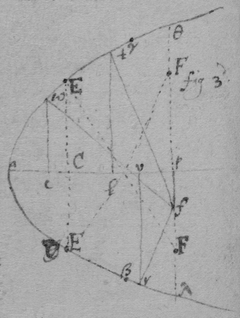By the 2d of these Equations 2 of ye valors of $\left(y\right)$ are united but not by ye increasing or diminishing ye Rad: of {illeg} ye ci valor of $d=fe$ &c. But 2 rootes one as {illeg} {illeg} first suppose ye circle soe little as noe where to intersect ye Parabola, it being increased gradually will fir{illeg}|st| touch ye Parab: at r (fig 3d) then ceasing to touch it it intersects it in 2 points g & k (fig 2d) wch two points growing more distant at last it untill it touch ye Parab: in t (fig 3d) wch being divided into two intersection points e & h (fig 2d) the points g & e draw neered|r| {sic} untill they conj{illeg}|o|yne in ye touch point w & soe ye circle ceaseth (by still increasing) to touch {o is} intersect ye Parab: or intersect it unlesse in h & k . Whence from one point f may be drawne 3 perpendiculars fr , fw , ft , to ye Parabola twar . And therefore in this 2d Equation $\left(y\right)$ must have 3 valors wc , tl , & vr , when $ap=d$, & $pf=-c$, are determined then also hath $\left(s\right)$ three valors fr , fw , & ft .

By ye 3d Equation Two of the {illeg} valors of $\left(y\right)$ in ye 2d Equation are united by incresing or diminishing ye length of $pf=-c$. For begining at ye point at the point p (from wch ye {illeg} \3 perpendiculars/ fall upon γ , a & β ) if ye point f doth gradually move from p , the perpendicular $\left\{\begin{array}{c}ft\\ fw\\ fr\end{array}\right\}$ moves from $\left\{\begin{array}{c}\gamma \\ a\\ \beta \end{array}\right\}$ towards $\left\{\begin{array}{c}a\\ \gamma \\ \lambda \end{array}\right\}$ Soe yt ye two perpendiculars = wf & tf will at last conjoyne into one {illeg}f EF , Which shall be ye Rad: of a Circle as crooked as ye Parab: at E .

This 3d operation might have beene done by making pf determined & by = increasing or diminishing $ap=d$ . That is by destroying ye term $-\frac{2dy}{r}$ in stead of $-c$ in ye 2d Equation. And so might ye 2d Operacon beene done otherwise by {illeg} determining ye circle egh , &|O|r taking c or d out of ye 1st Equation instead of $ss$ .

 There is another way of finding ye crookednesse in lines & yt is not by supposing {illeg}|t|wo perpendiculars ( wf & ft , or wf & fr ). but 3 intersections of a circle wth ye figure, (fig 2d h , e , g : or e , g & h ). And then shall $\begin{array}{c}x\\ y\end{array}\right\}$ have 3 equall valors $\left\{\begin{array}{cccccc}al& ,& ac& ,& as& .\\ lg& ,& fc& ,& eh\end{array}\right\}$ Or $\left\{\begin{array}{ccccc}ac& ,& al& ,& av\\ ce& ,& lg& ,& vk\end{array}\right\}$ . As if (in ye last example I had this equation $\begin{array}{cccccccccccccccc}{y}^{4}& \ast & -& 2dryy& +& rryy& -& 2cyrr& +& ccrr& +& ddrr& -& ssrr& =& 0\\ 3& & & 1& & 1& & 0& & -1& & -1& & -1& \end{array}$ . Supposing it to have 3 equall rootes by Huddenius his method tis $\begin{array}{cccccccccccccc}3{y}^{4}& \ast & -& 2dryy& +& rryy& -& ccrr& -& ddrr& +& ssrr& =& 0\\ 4& & & 2& & 2& & 0& & 0& & 0& \end{array}$ . (Which equation doth not determine ye perpendiculars to eag ) as $\frac{2{y}^{5}}{rr}\ast -\frac{2dy}{r}+y=c$ {illeg} doth for by { $12{y}^{4}-2dryy+rryy=0$ }. this I can find ye valor of c ( y being determined) {illeg} but by it I {illeg} {illeg} neither find ye valor of { c } nor {illeg}till one of ym is {illeg}|t|aken out of ye Equation). That Equation multiplyed {illeg} ye dimensions of y produceth $6{y}^{2}-2dr+rr$ Or {$\frac{3yy}{r}+\frac{r}{2}$ {illeg}$3x${illeg}{illeg}$=d$ }.

 The same may be done thus. If a circle touch a crooked line at one point & intersect it {illeg}er when two points come together yt circle {illeg} to {illeg} or {illeg}

As if $bc=y$. {$ac=\frac{yy}{r}$ $an=mt=d$. $pv=o${illeg} c{illeg}={illeg}$=\frac{r}{2}$. {illeg} {illeg} $-\frac{yy}{r}$ . $\frac{r}{2}:y\colon\colon \frac{dr-yy}{r}:\frac{2dry-{y}^{2}}{rr}=bq$}. {$\frac{r}{2}:${illeg} $+y\colon\colon$ {illeg} $=d-\frac{yy-2oy}{r}:\frac{2dry+2ro-2{y}^{3}-oyy}{rr}=pg$. {illeg} may ever bee {illeg}). Then {illeg}} {${bq}^{2}+{qt}^{2}${illeg} $6{y}^{4}-8dryyo+rryyo+2ddrro-d{r}^{3}o=0$ . Or $dd=\frac{8dryy}{2rr}$ {illeg}.} Or $d=$ {illeg} Or, {illeg} $d=$ {illeg} wch cannot {illeg} T{illeg} by ye {illeg} perpendiculars {illeg} by supposeing ye circle described by ye Rad: {illeg} pt & {illeg} to {illeg}

<33r>

##  Haveing found an Equa (by ye former rule) {illeg}|a|n Equation expressing\by which/ye quantity of crookednesse in any line may bee found to find ye greatest or least crookednes of any that line.

 In ye 3|4|d|t|h Example I had found $gf=qs=d=3x-r$ . And by a rule there shewed viz {$\begin{array}{c}as\\ ag\end{array}\right\}=c=\frac{xy+vy-dy}{v}$}: or writeing $3x-r$ in stead of d It was there found $\begin{array}{lllllll}4{x}^{3}& -& 8dxx& +& 4ddx& -& ddr\\ & -& 5r& +& 6dr& +& ccr\\ & & & +& 2rr& -& drr\\ & & & & & -& crr\end{array}=0$. {illeg}|N|ow by writeing {illeg} $3x-r$ in stead of d & ordering ye product according to ye letter c it is $\begin{array}{lllll}ccr& -& crr& +& 16{x}^{3}\\ & & & -& 12rxx\\ & & & -& 3rrx\end{array}=0$. Or extracting ye roote i{illeg}|t| is $c=\frac{r}{2}\phantom{\rule{0.5em}{0ex}}\stackrel{\cup }{O}\phantom{\rule{0.5em}{0ex}}\sqrt{12xx-3rx+\frac{rr}{4}-16\frac{{x}^{3}}{r}}=\left\{\begin{array}{cc}as& \text{.}\\ ag& \text{.}\end{array}$ Also by ye nature of ye line, $\begin{array}{c}kd\\ ed\end{array}\right\}=y=\frac{r}{2}\phantom{\rule{0.5em}{0ex}}\stackrel{\cup }{O}\phantom{\rule{0.5em}{0ex}}\sqrt{\frac{rr}{4}-rx}$. Therefore $kh=gl=\left\{\begin{array}{c}kd-sa\\ ga-ed\end{array}\right\}=\sqrt{\frac{rr}{4}-rx}+\sqrt{12xx-3rx+\frac{rr}{4}-\frac{16{x}^{3}}{r}}$ . Also $qh=le-fg=r-2x$ . And Since $\left\{\begin{array}{l}{he}^{2}+{gl}^{2}\\ {kh}^{2}+\end{array}$ ${he}^{2}+{gl}^{2}={qk}^{2}$ Therefore 
$\frac{3rr}{2}-8rx+16xx-\frac{16{x}^{3}}{r}+2\sqrt{12xx-3rx+\frac{rr}{4}-16\frac{{x}^{3}}{r}}×\sqrt{\frac{rr}{4}-rx}=qkqk=zz$; Supposing $qk=z$ The roote of ye Surde quantity extracted the Equation is $-\frac{16{x}^{3}}{r}+24xx-12rx+2rr=zz$. Or $16{x}^{3}-24rxx+12rrx-2{r}^{3}+rzz=0$. In wch Equation ye least valor of $z=fe$ is to {illeg} bee found & yt should happen when x hath 2 equall {illeg}|v|alors or rootes. But because $fe=z$ {illeg} being determined x can have but one valor $=ad$ ye other 2 {illeg} rootes being imaginary tis impossible yt it should have 2 equall rootes: Therefore I substitute {y}{ y } take away x out of ye Equation &|b|y substituting its valor $\frac{ry-yy}{r}$ in its stead & there results $\begin{array}{ccccccccccccccccc}16{y}^{6}& -& 48r{y}^{5}& +& 24rr{y}^{4}& +& 32{r}^{3}{y}^{3}& -& 36{r}^{4}yy& +& 12{r}^{5}y& -& 2{r}^{6}& +& {r}^{4}zz& =& 0\\ 6& & 5& & 4& & 3& & 2& & 1& & 0& & 0& \end{array}$. In wch equation z {illeg} or $ef=qk$ being {illeg}|d|etermined y hath 2 valors de & dk ye other foure being imaginary & when ef is {illeg} the longest or shortest that may bee then these two valors become one & then is ye line aek {illeg} more or least crooked. If therefore (yt y 's valors become equall) this Equation is multiplyed according to its dimensions there will result $8{y}^{5}-20{y}^{4}+8rr{y}^{3}+8{r}^{3}yy-6{r}^{4}y+{r}^{5}=0$ . wch is divisible by $y-\frac{r}{2}=0$ , or by $2y-r=0$ (for there results $4{y}^{4}-8r{y}^{3}+4{r}^{3}y-{r}^{4}=0$ ). And if $y=\frac{r}{2}$ , yn is $x=\frac{r}{4}$ . Therefore I take $ad=\frac{r}{4}$ & $de=\frac{r}{2}$ & |at| ye point e shall bee ye least crookednesse.

Here may bee noted Huddenius his mistake, yt if i{illeg} some quantity in an equation designe a maximum or minimu yt Equation hath two{illeg} equall rootes & {illeg} wch {illeg}|is| false in ye equation $16{x}^{3}-24rxx+12rrx-2{r}^{3}+rzz=0$. & in all other equation's {sic} which have but one roote.

Or because

## Another way. May 1665

 Or because ye lines fn & qn described by ye points f & q doe touch one another point's {sic} n from wch points onely {illeg} lines drawne perpendicular to ye croked {sic} line kea will bee perpendicular to ye \point of/ greatest or least point crookednesse: And also since all those are points of greatest or least crookedness f|t|o wch such {illeg} \perpendiculars/ are drawne: The difficulty will be to find ye point n . Now suppose yt $am=d$ be determined yn c hath two valors for $\begin{array}{c}mf\\ mq\end{array}\right\}=c$ . And alsoe y hath two valors for $\begin{array}{c}dk\\ de\end{array}\right\}=y$ . A{illeg}|l|soe (when am is not parallell to ye axis of ye line) x hath {illeg} two (or more) valors $\begin{array}{c}ad\\ ad\end{array}\right\}=x$ . wch valors of c , x , or y become equall if $am=an$ : {illeg}|b|y wch meanes ye point n may bee found: Excepting onely {illeg}|w|hen fm , mq , are parallel to ye crooked line at n \({ un }{)}/  yt is, parallell \perpendicular/ to ye streightest or most crooked ptes of ye line aek . But if $as=c$ be de{illeg}|t|ermined, then $d=\left\{\begin{array}{cc}st& \text{.}\\ sf& \text{.}\end{array}$ $x=\left\{\begin{array}{cc}av& \text{.}\\ ad& \text{.}\end{array}$ $y=\left\{\begin{array}{cc}rv& \text{.}\\ ed& \text{.}\end{array}$ (but if $\left(as\right)$ is parallel to ye axis of ye line ye two valors of y are equall & soe not usefull). Which valors of d , x, & y become equall if $as=\mathrm{b}\phantom{\rule{0.2em}{0ex}}un$ : excepting onely when as is perpendicular to ye most streight or crooke{illeg}|d| pts of ye line ake .

 As for example. In ye precedent example it was found $d+r-3x=0$ . But b{illeg}|e|cause an or x is parallell to ye axis of ye line, in yt {illeg}|E|quation x hath but one dimension. Therefore substitute either ye valors of d or of x into their stead. As if I substitute ye valor of $x=\frac{ry-yy}{r}$ into its place it will bee $d+r-3y+\frac{3yy}{r}=0$ or $\begin{array}{ccccccccc}3yy& -& 3ry& +& rr& +& dr& =& 0\\ 2& & 1& & 0& & 0& \end{array}$. wch must have 2 equall roots & therefore multiplyed according to y 's dimensions tis $6yy-3ry=0$ . Or $y=\frac{r}{2}$ as before. But if I had substitu{illeg}|t|ed d 's valor into its stead it would have beene $\begin{array}{ccccccccccc}16{x}^{3}& -& 12rxx& +& 3rrx& -& crr& +& ccr& =& 0\\ 3& & 2& & 1& & 0& & 0& \end{array}$ {illeg}|w|hich {illeg}ving 2 equall roots being rightly ordered is $48{x}^{3}-24r{x}^{2}+3rrx=0$ . Or $16{x}^{2}-8rx+rr=0$ . Or $4x-r=0$ . Or $x=\frac{r}{4}=0$ , as before.

{In ye first} Example of finding ye quantity of crookednesse in lines ye {illeg} found { $\begin{array}{c}4r{x}^{3}\\ \phantom{0}\end{array}$ {illeg} $\begin{array}{ccccccc}\frac{\phantom{00}xx+3rx}{q}& -& 3x& -& \frac{r}{2}& =& 0\\ 1& & 1& & 0& \end{array}$ }. wch {must} have 2 equall rootes & therefore by Hudde{nius} method it is {{illeg}$xx-4qxx-4qrx+4qqx+qqr-{q}^{3}=0$}. {illeg} \wch being divided by { $2x-q$ } it is/ $2rx-2qx-qr$ . Or, $4xx-4qx+qq=0$ .{illeg} That is $2x=y$ . or $x=$ {illeg} $=y$ Or {illeg} = {illeg} $=y$ {illeg} yt if I take { $us=\frac{r}{2}$ } & {illeg} $\right\}=$ {illeg} $=y$ {illeg} ye points {illeg} ye greatest or least crookednesse {illeg} ye line {illeg} {illeg} crookednesse wch {illeg} found

{These Equations} {illeg} superfluous rootes {illeg} often as {illeg} ye perpendiculars {illeg} {illeg}

<33v>

The points of greatest or least crookednesse may bee yet otherwise found by an equation of 4 equall rootes. As in ye example of ye 2d way of finding ye quantity of crookedness in lines it was found ${y}^{4}\ast -2dryy+rryy-2crry+ccrr+ddrr-ssrr=0$ . wch being compared wth an equation like it ${y}^{4}-4e{y}^{3}+beeyy-4{e}^{3}y+{e}^{4}=0$ . by ye 2d terme tis $4e{y}^{3}=0$ , or $y=0$ . In like manner by & $\frac{yy}{r}=\frac{00}{r}=0=x$ . Soe yt ye Parab at ye {illeg}|b|egining is most crooked (at a ).

<38r>

If ye body{illeg} b {illeg} {illeg}|m|ove {fro} ye line bd & from ye point {illeg}| d | two lines da , dc bee drawne ye motion of ye body b from ad is to its m{illeg}|ot|ion from dc as $ab\parallel dc$ is to $cb\parallel ad$.

 Coroll: 1. The body b receiving two divers forces from a & c & ye force from ba is to ye forc{e} from { bc } as ba to bc , yn draw $ad\parallel bc$ & $cd\parallel ab$ , ye body b shall bee moved in ye line {illeg} / bd \.

 2d Or if y{illeg}|e| body { b }{ d } is suspended by ye {thred} bd & is forced from a to {illeg} & from { c } towards f , yn draw $dc\parallel ab$ & $da\parallel${illeg}, & make $da:ab\colon\colon$ force from c to f ∷ force from {illeg} ye body {illeg} {illeg}nd in Equilibrio is b .

{illeg}Coroll: 3d. ye force of ye body b from d i{illeg}|s| to its force from a as bd to ba .

<38v>

vide pag: 15. But here observe yt unlesse ye reflecting line adn bee drawne from ye through ye point $\left(w\right)$ the center of motion in ye whole body aiwn ye determinacon of ye motion of adn will not be ye same wth ye determinacon of ye motion of g before reflection (as in ye first figure) but verge from it (as in ye 2d fig) yt is wl & gdi will not bee parrallell {sic}. For since ye cheife {sic} resistan{ce} is in ye line of ye body adn is from its center of motion (prop 32) from d w towards d , & not from i towards d , the body g will find more opposition on yt side towards ye center w , yn on ye other side towards a & therefore at its reflection it{illeg} must incline toward v {illeg} from ye \(ax 120)/ &{illeg} not returne in ye line dg . But if ye body awn presse g towards w yn g presseth ye body awn towards ye contrary pte \as/ from w towards l (ax 119) & not from w towards m, if $wm\parallel dg$. But if adn {illeg}|t|he line adn pass through ye point w (as in fig: 1st) yn

38 If ye superficies abr (fig 3d) circulate all its points in ye line cd move wth equall velocity from c towards d. For make $\angle sfr=tsr=\text{recto}$ . & $\angle srf=crt$ & draw $ta\perp dc$ than is ye motion of ye point e from c to ye motion of ye point f from c as ae to sf. but $ae=sf$ (for △ rsf similis △ ret therefore $\frac{er×sf}{fr}=et$. also aet similis △ erf therefore $er:fr\colon\colon et:ae$. or, $\frac{er×ae}{fr}=et=\frac{er×sf}{fr}$ & $ae=sf$ ) therefore ye motion of e from c is equall to ye motion of f from c.

39 If ye body g move reflect on ye immoveable surface \$\left(dv\right)$ / a{illeg}|t| its corner {illeg}| o | (fig 4th) its parallell motion (viz from d to v ) shall not bee hindered {illeg} by ye surface dv , (viz: if ye center of g 's motion were distant from ye perpendicular dm an inch at one minute before reflection it shall bee s{illeg}|o| farr {sic} distant from it one minute after reflection). For dv i{illeg}|s| noe ways opposed to motion parallell to it, & a body might $\left\{\begin{array}{c}\text{slide}\\ \text{move}\end{array}\right\}$ upon it wthout l{illeg}|o|oseing any motion, & if at ye first moment of contact ye body g should loose its ⊥ & onely keepe its ∥ motion it would (perhaps) continue to slide upon it & not reflect.

40 The body g reflecting on ye plaine vd at its cor{illeg}ner {illeg} o all its points in ye ⊥ line $op\perp vd$ shall move from ye plaine vd wth ye same velocity wch before reflection {illeg} \they/ moved fro to it. For ye point o (prop 9) moves wth yt velocity bacwards {sic} wch it before did forwards (vi{illeg}|z| to vd ) & all ye other points (prop 38) move wth ye same velocity from it.

<47r>

##  A Method for finding theorems concerning Quæstions de Maximis e{illeg}|t| minimis. And 1st Concerning ye invention of Tangents to crooked lines.

 Suppose $ab=x$. $eb=y$. $bd=v$. $bc=o$. $cf=z$. & $ed=df$. ye nature of ye line $ax+xx=yy$. Then is $ac=x+o$. $ax+ao+xx+2ox+oo=zz$. $vv+yy={ed}^{2}={fd}^{2}=zz+vv-2ov+oo$. Or $yy=oo-2ov+zz$ Or $yy=oo-2ov+ax+ao+xx+2ox+oo$. & since $ax=yy-xx$. Therefore $0=2oo-2vo+ao+2xo$. Or $2×o-2v+a+2x=0$. Now yt ed may bee perpendicular to ye line tis required yt ye points e , & f conjoyne, wch will hapen {sic} when $bc=o$ {illeg}|v|ani{illeg}sheth into nothing. Therefore in the equation $2×o-2v+a+2x=0$. Or \{illeg}/ $v=o+\frac{a}{2}+x$, those termes in wch $\left(o\right)$ is must be blotted out, & there remaines $v=x+\frac{a}{2}=bd$. wch determines ye perpendicular ed .

 Hence it appeares yt in such like operations those termes may be ever blotted out in wch $\left(o=b\right)$ is of more yn one dimension.

As if ye nature of ye line was ${x}^{3}+xxy+xyy=ayy$ . Then is {illeg} since $ac=o+x$ it is ${x}^{3}+3{x}^{2}o+3xoo+{o}^{3}+xxz+2xoz+ooz+xzz+ozz=azz$. That is ${x}^{3}+3{x}^{2}o+xxz+2xoz+ooz+x{z}^{2}+o{z}^{2}=a{z}^{2}$ . Also $vv+yy=vv-2vo+oo+zz$ . or $yy+2vo=zz$ . Therefore {illeg}
$ayy-xyy-xxy\left(={x}^{3}\right)+3xxo+xxz+2xoz+ozz\left(+xzz-azz=\right)+xyy+2vox-ayy-2voa=0$. That is $xxy+3xxo$ $xxy-3xxo-2xoz-ozz+2voa-2vox=xx\sqrt{yy+2vo}$. That is (both pts □ed {illeg}|&| those terms left out in wch o is of more yn one dimension) $x+yy+\stackrel{_}{2xxyin2voa-2vox-3xxo-2xoz-ozz}={x}^{4}yy+2vo{x}^{4}$ Or $yin2voa-2vox-3xxo-2xoz-ozz=voxx$. That is $-3xxy-2xzy-zzy=vxx+2vxy-2vay$. Now if $bc=o$ vanisheth yn is $z=y$. And consequently $\frac{-3xxy-2xyy-{y}^{3}}{xx+2xy-2ay}=v=\frac{3xxy+2xyy+{y}^{3}}{2ay-2xy-xx}$.

 Hence I observe yt if {illeg} in ye valor of y there be divers termes in wch x is then in ye valor of z there are those same termes & also those termes \each of ym/ multiplyed according to ye by so many units as x hath dimensions in yt terme & againe multiplyed by o & divided by x . As if ∼ ∼ ∼ ${x}^{3}+xxy+xyy-ayy=0$ . Then, ${x}^{3}+xxz+xzz-azz+\frac{3{x}^{3}o+2xxoz+xozz}{x}=0$. Which operacon may bee conveniently symbolized by (ordering ye equation according to ye dimensions of y ) &) making some letter \(as a . e . m . n . p )/ to signifie a terme, & ye same letter wth some marke (as $\stackrel{̈}{a}$, $\stackrel{̈}{c}$, $\stackrel{̈}{e}$, $\stackrel{̈}{g}$, $\stackrel{̈}{m}$, $\stackrel{̈}{n}$, $\stackrel{̈}{p}$ &c), to signifie ye same terme multiplyed according to ye dimensions of x in it as in ye former example (suposing {sic} $x-a=m$. $xx=n$. ${x}^{3}=p$ .) The nature of ye line is $\left\{\begin{array}{cccc}\text{in letters}\hfill & \phantom{\rule{1em}{0ex}}xyy-ayy+xxy+{x}^{3}=0& & xzz-a{z}^{2}+{x}^{2}z+{x}^{3}+\frac{xozz+2xxoz+3{x}^{3}o}{x}=0\text{.}\\ & & & \text{&}\\ \text{in their symbols}& \phantom{\rule{1em}{0ex}}myy+ny+p=0& & mzz+nz+p+\frac{\stackrel{̈}{m}zzo+\stackrel{̈}{n}zo+\stackrel{̈}{p}o}{x}=0\text{.}\end{array}$ Soe if $\begin{array}{ccc}\underbrace{{a}^{4}+a{x}^{3}+bb{x}^{2}-abbx}& =& {y}^{4}\text{.}\\ \phantom{{y}^{4}=}m={y}^{4}\text{.}\end{array}$ Then $\begin{array}{ccccc}\underbrace{3ao{x}^{2}+2bbox-abbo}& +& \underbrace{a{x}^{3}+bb{x}^{2}-abbx+{a}^{4}}& =& {z}^{4}\text{.}\\ \phantom{\phantom{\rule{1em}{0ex}}\sim \phantom{\rule{2em}{0ex}}\sim }\frac{\stackrel{̈}{m}o}{x}\phantom{\rule{2em}{0ex}}\sim \phantom{\rule{1em}{0ex}}\sim & +& \phantom{\sim }\sim \phantom{\rule{0.6em}{0ex}}\sim \phantom{\sim }\phantom{\rule{0.3em}{0ex}}m\phantom{\rule{0.3em}{0ex}}\sim \phantom{\rule{0.2em}{0ex}}\sim \phantom{\rule{0.2em}{0ex}}\sim \phantom{\rule{0.2em}{0ex}}\sim & =& {z}^{4}\text{.}\end{array}$ $\frac{\stackrel{̈}{m}o}{x}+m={z}^{4}$ .

A{illeg} And as any particular Equation may be thus symbolized so divers equations may bee represented by ye same caracters as $0=a+cy+yye$ may represent all equations in wch y is of one & two dimensions

Now if a generall Theoreme be required fo{illeg}|r| drawing tangents to such lines it may bee thus found. $eb=y$, $bd=v$, $ab=x$, $bc=o$, $fc=z$, by supposition, $a+cy+eyy=0$ . Then {illeg}/x\ \by observation ye 2d/, $a+cz+ezz+\frac{\stackrel{̈}{a}o+\stackrel{̈}{c}oz+\stackrel{̈}{e}ozz}{x}=0$. Or, $-cyx-eyyx\left(=xa\right)+czx+ezzx+\stackrel{̈}{a}o+\stackrel{̈}{c}oz+\stackrel{̈}{e}ozz=0$. Againe ${eb}^{2}+{bd}^{2}={cf}^{2}+{cd}^{2}$ that is. $yy+2ov=zz$. Which valor {illeg} of $zz$ put into its stead in ye termes $ezzx$ \& $czx$/ in ye former Equation the result is $+cyx-\stackrel{̈}{a}o-\stackrel{̈}{c}oz-\stackrel{̈}{e}ozz-2eovx=cx\sqrt{yy+2ov}$. And both pts squared it is (by ye first Observacon) $ccyy{x}^{2}-2cyx\stackrel{̈}{a}o-2cxy\stackrel{̈}{c}oz-2cxy\stackrel{̈}{e}ozz-4cxyeovx={c}^{2}xxyy+2ccxxov$. Wch rightly ordered is $-\stackrel{̈}{a}y-\stackrel{̈}{c}yz-\stackrel{̈}{e}yzz=2exyv+cxv$. And since ye points e & f conjoin{illeg}|e| to make ed a perpendicular therefore is $z=y$ & consequently $\frac{-\stackrel{̈}{a}y-\stackrel{̈}{c}yy-\stackrel{̈}{e}{y}^{3}}{cx+2exy}=v$ . Wch is ye Theorem sought for. As for example were it required to draw a perpendicular to ye line whose nature is $\begin{array}{cccccc}{x}^{3}& +& xxy& \begin{array}{ll}+& x\\ -& a\end{array}yy& =& 0\text{.}\\ a& +& \hfill cy& \begin{array}{l}\\ +& e\end{array}yy& =& 0\text{.}\end{array}$ Then is $\frac{-\stackrel{̈}{a}y-\stackrel{̈}{c}yy-\stackrel{̈}{e}{y}^{3}}{cx+2exy}=\frac{-3{x}^{3}y-2xxyy-x{y}^{3}}{{x}^{3}+2xxy-2axy}=v$ or $v=\frac{3xxy+2xyy+{y}^{3}}{2ay-2xy-xx}$.

In like manner to draw tangents to those lines in wch y is of 1, 2 & 3 dimensions suppose $a+cy+eyy+g{y}^{3}=0$. Then is {illeg} $yy+g{y}^{3}\left(=ax\right$ \by 2d observacon/ $-cyx-eyyx-g{y}^{3}x+\stackrel{̈}{a}o+czx+$ {illeg}/{illeg}\ $=0$ & by writeing ye valor of $z=\sqrt{yy+2vo}$) in its stead in th{illeg}|o|se te{illeg}rmes in wch {illeg} not (viz {illeg} $+g{z}^{3}x$ there results { $cyx+g{y}^{3}x-\stackrel{̈}{a}o-\stackrel{̈}{c}zo-e{z}^{2}o-g{z}^{3}o-2exvo=cx+gyyx-2vogx-\sqrt{yy+2vo}$ }. {illeg} {illeg} by {illeg} it is
{illeg}{$2go{z}^{3}=4eovx=cx+gyyxincx+gyy$ }{illeg}$inyy+2vo$. Or {illeg}{$+gyyovx+4vogxyy$ }. That is { $\frac{-\stackrel{̈}{a}y-\stackrel{̈}{c}yy-\stackrel{̈}{e}{y}^{3}-\stackrel{̈}{g}{y}^{4}}{cx+2exy+2gxyy}=v$ }.

By ye s{illeg}|a|me proceeding {illeg} of 1, 2, 3 {illeg} dimensions in {$a+cy+${illeg} $g{y}^{3}$ {illeg}} it would be found {illeg} $=v$ . &c {illeg} {illeg}

<47v>

 Having ye nature of a crooked line expressed in Algebraicall termes wch are not put one pte equall to another but all of ym equall to nothing, if each of ye {illeg} termes be multiplyed by soe many units as x hath dimensions in them. & then multiplyed by y & divided by x they shall be a numerator: Also if the \signes be changed &/ each terme be multiplyed by soe many units as y hath dimensions in yt terme{illeg} & yn divided by y they shall bee a denominator in ye valor of v .

Example 1st. If $rx+\frac{rxx}{q}-yy=0$. Then $\frac{\begin{array}{cccccc}& 1& & 2& & 0\\ \phantom{-}& rx& +& \frac{r}{q}xx& -& yy& in& \frac{y}{x}\end{array}}{\begin{array}{cccccccc}-& rx& -& \frac{r}{q}xx& +& yy& in& \frac{1}{y}\\ & 0& & 0& & 2\end{array}}=\frac{ry+2\frac{r}{q}xy}{2y}=\frac{r}{2}+\frac{r}{q}x=v$ . Example 2d. If ${x}^{3}+xxy+xyy-ayy=0$. Then $\frac{\hfill 3{x}^{3}+2xxy+xyy\phantom{\rule{1em}{0ex}}in\phantom{\rule{1em}{0ex}}\frac{y}{x}}{-xxy-2xyy+2a{y}^{2}\phantom{\rule{1em}{0ex}}in\phantom{\rule{1em}{0ex}}\frac{1}{y}}=v=\frac{3xxy+2xyy+{y}^{3}}{-2ay-2xy-xx}$. Exam: 3d. If ${x}^{3}+bxx-cdx+bcd+dxy=0$ . Then $\frac{3xxy-2bxy-cdy+dyy}{-dx}=\frac{2by}{d}-\frac{3xy}{d}+\frac{cy}{x}-\frac{yy}{x}=v$. And by taking y out of ye valor of v yn, $v=\frac{2{x}^{3}}{dd}-\frac{3bxx}{dd}+\frac{bbx}{dd}-\frac{2cx}{d}+\frac{2bc}{d}+\frac{bcc}{xx}-\frac{bbcc}{{x}^{3}}$ .

Note. That haveing $\left\{\begin{array}{c}x\\ y\end{array}\right\}$ given, it will be often {illeg} \more/ convenient to find $\left\{\begin{array}{c}y\\ x\end{array}\right\}$ by ye equation expressing ye nature of ye line & yn having x & y to find v by them both, Then to take $\left\{\begin{array}{c}y\\ x\end{array}\right\}$ out of v 's valor & soe to find it by $\left\{\begin{array}{c}x\\ y\end{array}\right\}$ alone.

## The Perpendiculars {illeg}|t|o crooked lines & also ye Theorems ∼ for finding them may otherwis{illeg} more conveniently \be/ found thus

 Supposing $ab=x$; $cb=o$, $db=v$, $eb=y$, $cf=z$. {illeg} And if ye fc bee supp distance twixt fc & fb , bee imagined to bee infinitely little, yt is if ye triangle efr is supposed to bee infinitely little then $be:bd\colon\colon bg:be\colon\colon re:fr\colon\colon y:v\colon\colon o:z-y$. That is $yz-yy=vo$. Or $z=y+\frac{vo}{y}$.

Now suppose ye nature of ye line bee $rx-\frac{rxx}{q}-yy=0$. Then is $rx+ro-rxx-2rox-rro-{z}^{2}${$=0$}

In wch equation instead of $rx\left(=\frac{rxx}{q}+yy\right)$ & $zz\left(=yy+2vo+\frac{vvoo}{yy}\right)$ write theire valos {sic} & ye result is $ro-\frac{\begin{array}{ccc}2rox& -& 2voq\\ & -& roo\end{array}}{q}-\frac{vvoo}{yy}=0$. Or $r-\frac{2rx-ro}{q}-2v-\frac{vvo}{yy}=0$. but these two termes $ro$ , $\frac{vvo}{yy}$ are infinitely little, yt is if compared to finite termes they vanish therefore I blot ym out & there rests $\frac{r}{2}-\frac{rx}{q}=v=db$ .

Suppose ye nature of ye line be $p+qy+ryy=0$ Then (by observation ye 2d) it is {illeg} $-qyx-ryyx\left(=px\right)+\stackrel{̈}{p}o+qzx+\stackrel{̈}{q}zo+rzzx+\stackrel{̈}{r}zzo=0$. Then writeing ye valor of $z\left(=y+\frac{vo}{y}\right)$ in its stead in these termes $qzx+rzzx$, There results $\stackrel{̈}{p}o+\frac{qxvo}{y}+\stackrel{̈}{q}zo+2rxvo+\stackrel{̈}{r}zzo=0$. Or because ye difference twixt z & y is infinitely little it is $\frac{\stackrel{̈}{p}y+\stackrel{̈}{q}yy+\stackrel{̈}{r}{y}^{3}}{-qx-2rxy}=v$.

 And though ye angle ebg made by intersection of x & y is not determined {illeg}|w|hither {sic} it acute obtuse or a right one, yet may ye line bg bee found as easily after ye same manner wch determines ye position of ye tangnt eg . For suppose {illeg}$=t$ . cb {illeg} $cb=y$, $fc=z$, $ab=x$, & yt $z\parallel y$. Then (supposing ye distance of fc & eb to be {infinit}ely little) it is, $t:y\colon\colon t+o:y+\frac{oy}{t}=z$. Now if ye nature of ye line is $p+qy${illeg}${y}^{3}=${0 } Then is $-qyx-r{q}^{2}x-s{y}^{3}x\left(=px\right)+\stackrel{̈}{p}o+qxz+\stackrel{̈}{q}oz+rx{z}^{2}+\stackrel{̈}{r}o{z}^{2}+sx{z}^{3}${illeg} And by putting ye valor of z into its stead in those terms in wch {illeg} results $\stackrel{̈}{p}o+\frac{qxoy}{t}+\stackrel{̈}{q}oz+\frac{2rxo{y}^{2}}{t}+\stackrel{̈}{r}o{z}^{2}+\frac{3sxo{y}^{3}}{t}+\stackrel{̈}{s}o{z}^{3}=0$. Or $t=$ {illeg}

Soe yt ye variation of ye angle ebg makes no variation.

Note yt th{illeg}|e| foundacon of this operacon of yt {illeg} {illeg} pag 131) {illeg} tangents {illeg} But since {illeg} Equation is the sa{illeg} {illeg}stons yt it would bee if {illeg} {illeg}

<50r>

## To draw perpendiculars to crooked lines in all other cases.

Although ye unknone {sic} quantitys x & y are not related to one another as in the precedent rules (yt{illeg} that is soe yt y move upon x in a given angle), y{illeg}|et| may there be drawne tangents to them by ye same metho{illeg}|d|.

 As if efm is an Elipsis {sic} described by ye thred aen (as is usuall) Then make $gb=v$. $en=y$. $an=a$. $x+y=${illeg} And let ye relation twixt x & y be $x+y=b$. $ab=z$ . Then is $bn=a-z$. ${eb}^{2}=xx-zz=yy-aa+2az-zz$ . And consequently $\frac{xx-yy+aa}{2a}=z=ab$ And. And $xx\phantom{\rule{1em}{0ex}}\frac{-{x}^{4}+2xxyy-2xxaa-{y}^{4}+2aayy-{a}^{4}}{4aa}={eb}^{2}=\frac{2xxyy+2xxaa+2aayy-{x}^{4}-{y}^{4}-{a}^{4}}{4aa}={ae}^{2}-{ab}^{2}$ . Againe suppose $af=s$, $fn=t$, $bc=o$. Then is $ac=x+o=o+\frac{xx-yy+aa}{2a}=\frac{ss-tt+aa}{2a}$. That is $2ao+xx-yy+tt=ss$. Alsoe $v:g\colon\colon v+o:g+\frac{og}{v}=fc$. And ${fc}^{2}=gg+\frac{2{g}^{2}o}{v}=\frac{2sstt+2ssaa+2ttaa-{s}^{4}-{t}^{4}-{a}^{4}}{4aa}$ {illeg} Making $eb=g$ . And consequently $\frac{2xxyy+2xxaa+2aayy-{x}^{4}-{y}^{4}-{a}^{4}}{4aa}+\frac{2{g}^{2}o}{v}=\frac{2sstt+2ssaa+2ttaa-{s}^{4}-{t}^{4}-{a}^{4}}{4aa}$ Or $2xxyy+2xxaa+2aayy-{x}^{4}-{y}^{4}-{a}^{4}+\frac{8aa{g}^{2}o}{v}=4ttao+2ttxx-2ttxx-2ttyy+{t}^{4}+4{a}^{3}{o}^{3}+2aaxx-2aayy+4aatt-{a}^{4}-{s}^{4}$ .  Or $4aoxx-4aoyy+\frac{8aaggo}{v}+4aayy-{t}^{4}-4{a}^{3}o-4aatt=0$ . Lastly by ye nature of ye line $b-t=s$. And $ba-2bt+tt=ss=2ao+xx-yy+tt$. Or $\frac{-2ao-xx+yy+bb}{2b}=t$. And { $4tt=\frac{4aoxx-4ao{y}^{2}-4abbo+Q:\phantom{\rule{0.5em}{0ex}}-xx+yy+bb\phantom{\rule{0.5em}{0ex}}:}{2bb}$ } wch valor substituted into ye Equation A, the result is {$-{t}^{4}-8{x}^{3}o+6axyy-{x}^{4}-{y}^{4}-{a}^{4}+2axyy-2xxaa=0$} $\frac{8aaggo}{v}-{x}^{4}-{y}^{4}-{a}^{4}+2xxyy-2xxaa+2aayy=0$.
{$\frac{8aaggo}{v}\phantom{\rule{1em}{0ex}}\frac{+4{a}^{3}bbo-4{a}^{3}bbo-{x}^{4}{a}^{2}+2xxyyaa+2aabbxx-aa{y}^{4}+2aabbyy-{b}^{4}a}{bb}$ }.

 As if

[Editorial Note 2]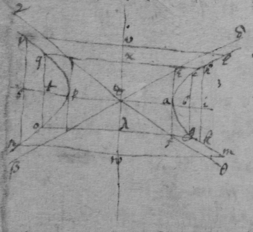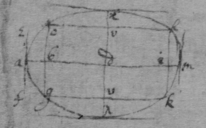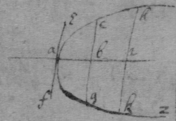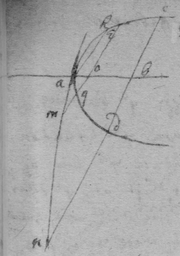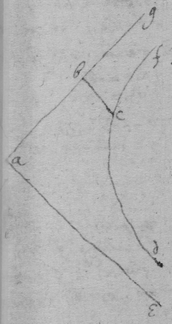October 1664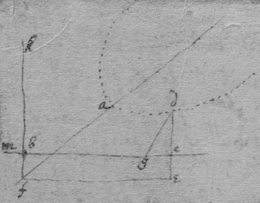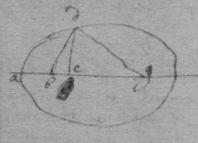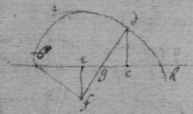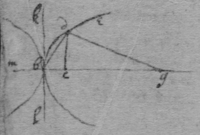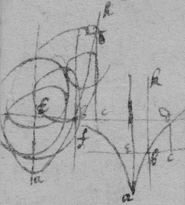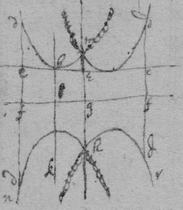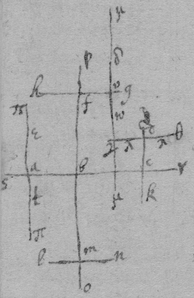For ye first equation of ye first sort

 For ye 2d

 For ye 3d

 For ye 4th

 For ye 5t

 For ye 6t &c

 For ye first Equation of ye seacond Sort

 For ye seacond

 For ye 3d.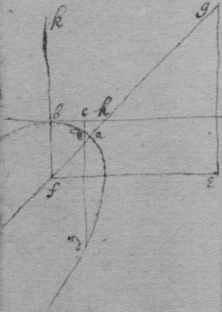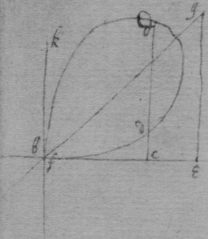November 1664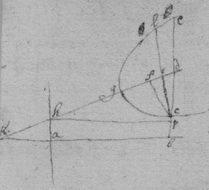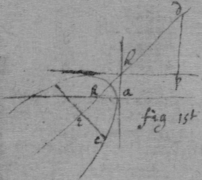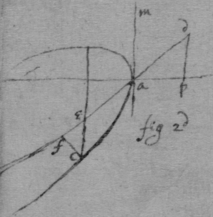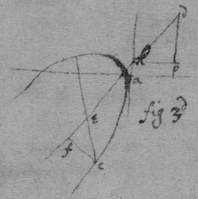November 1664

 A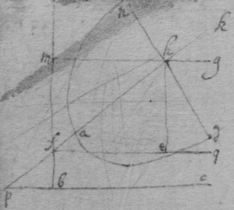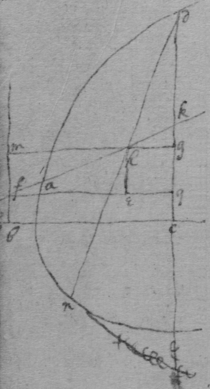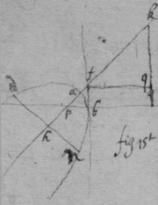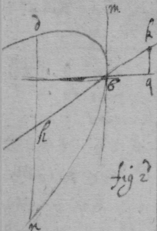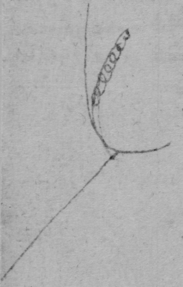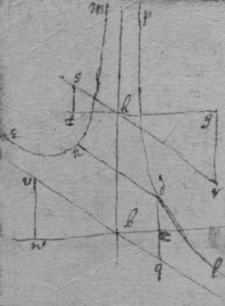B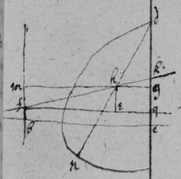This line is a streight one ye equation being divisible by $b=y=0$

 Endeavor not to find ye quantity d in these cases, but suppose it given[Editorial Note 1]

[Editorial Note 1] There is a line connecting the end of this note to the following one

 Or else C

 December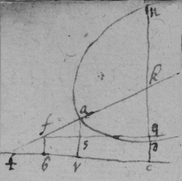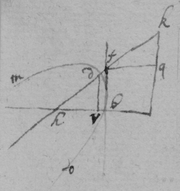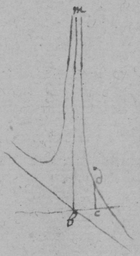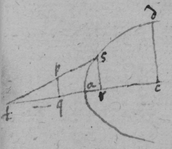F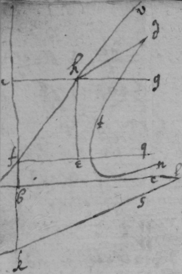G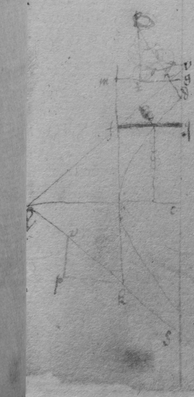December 1664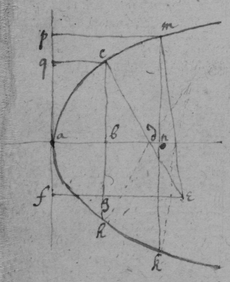Theorema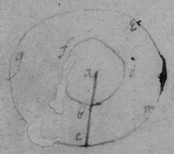December 1664.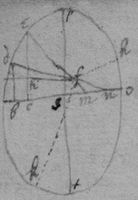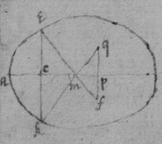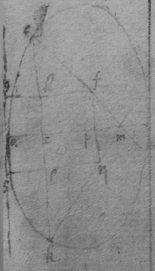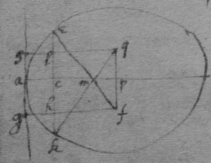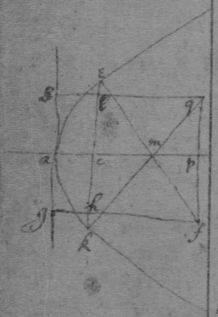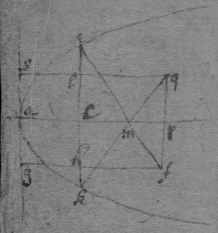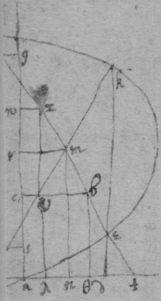Feb 1664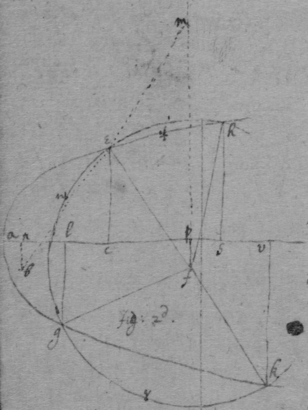Another way.December 1664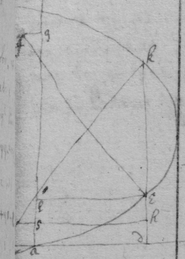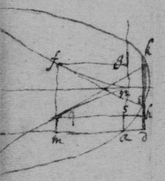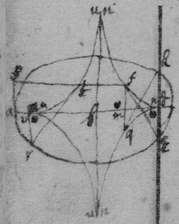Of compound force.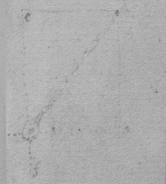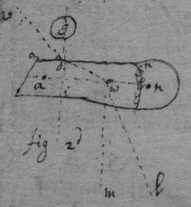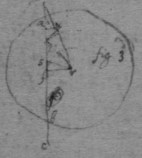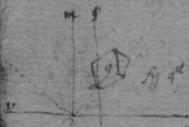March \May/ 20th 1665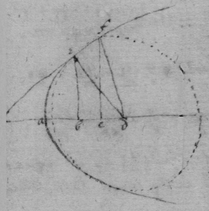Theoreme 1st Observation 1st

 Observacon 2d

 An universall theorem for tangents to crooked lines, when $y\perp x$.

 See Des Cartes his Geometry. booke 2d, pag 42, 46, 47. Or thus, $\begin{array}{ccccccccc}{x}^{3}& -& bxx& -& cdx& +& dyx& +& bcd\\ 2& & 1& & 0& & 0& & -1& \end{array}$ { $\frac{2xxy-bxy}{-dx}\phantom{\rule{0.5em}{0ex}}\frac{+bcdy}{dxx}=v$ }. And $\frac{bcy}{xx}+\frac{by}{d}-\frac{2xy}{d}=v$ .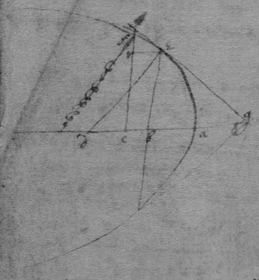An universall theo{illeg}|r|em for drawing tangents to crooked lines when x & y intersect at any determined angleA=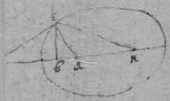[Editorial Note 2] The rest of the page is damaged.